Allen's Whiteboard 2019-06-17T04:11:38.139Z http://allenfrostline.com/ Allen Frostline Hexo Hybrid Sentiment-Momentum Strategy in Cryptocurrency Market http://allenfrostline.com/2019/06/09/crypto-news/ 2019-06-09T16:04:00.000Z 2019-06-17T04:11:38.139Z

This is an exploratory research trying to implement a simple yet robust sentiment analysis mechanism in the cryptocurrency market. We call it a hybrid sentiment-momentum strategy as it applies context analysis (or more specifically, word analysis) into traditional momentum strategies and adjusts position automatically over time using both factors. The hybrid strategy is implemented with backtesing period from Dec. 17th 2017 to May 2nd 2019. It yields cumulative return of 229% and a remarkable Sharpe ratio of 2.68 with acceptable maximum drawdown in out-of-sample backtest, which is a huge improvement from classical momentum strategies in our analysis.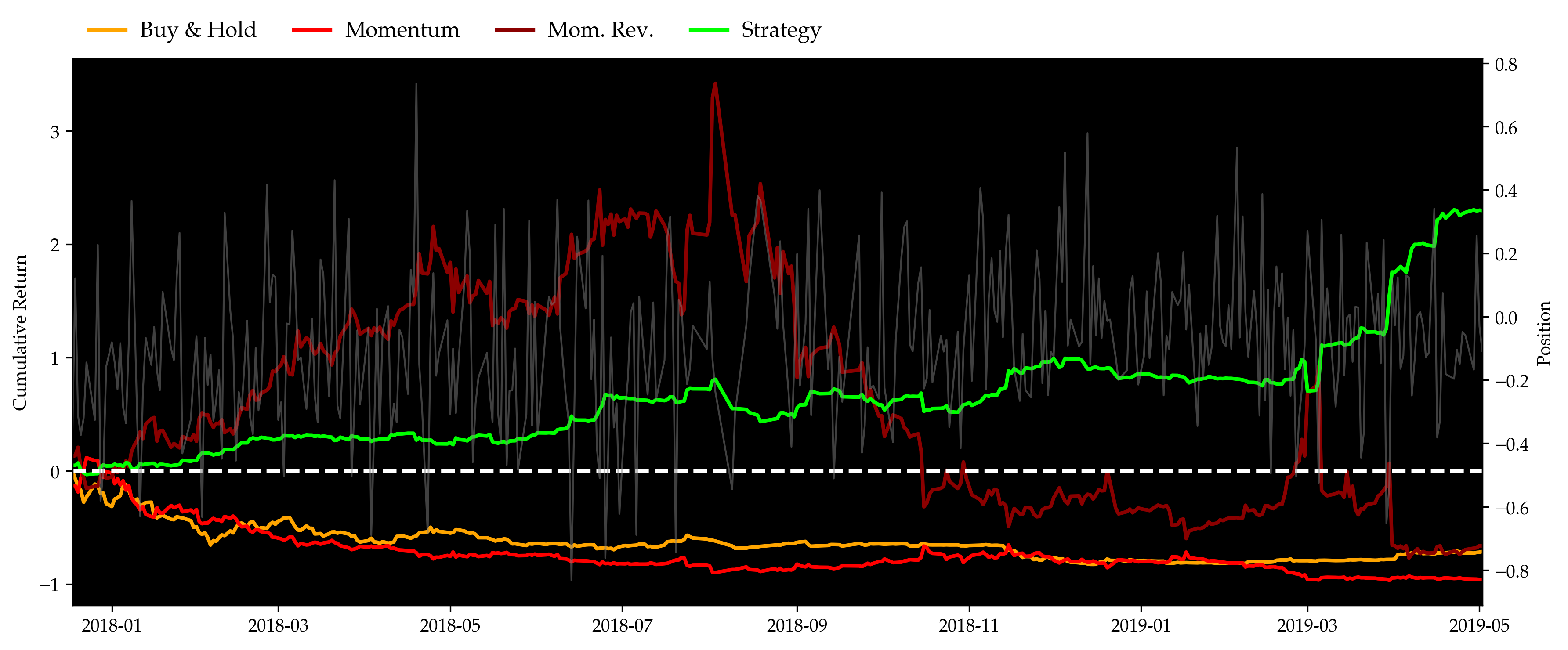## Introduction ↩︎

Cryptocurrency has been a hot topic ever since the creation of Bitcoin in 2009. Unlike stocks which are backed by real companies, cryptocurrency has no intrinsic value, causing its price to be heavily reliant on market information. The decentralization of exchanges, the inconsistency of prices, the lack of tangible entity and assets all present serious analytics challenges that are difficult to solve with traditional tools. Therefore, we want to introduce news data into our strategy.

There are many reasons to believe that momentum strategy will perform well in this market. Compared to other markets, there are fewer participants in the cryptocurrency market; thus, information is both diffused and acted on slowly. In addition, a smaller number of participants leads to a lack of liquidity, forcing large investors to spread out transactions over time. Last but not least, the limited information source makes the decision of investors largely dependent on recent performance, which further drives the herd mentality. However, there are no less reasons to think that momentum strategy will fail. We need something else to help us decide when we should use momentum strategy and when we may want to go opposite. So we go one step further and develop a new strategy that we call hybrid sentiment-momentum strategy.

On the other hand, the cryptocurrency market is mostly driven by the overall investors' fanaticism, which suggests that momentum strategy would perform extremely well when this market is optimistic and bullish. However, when fanaticism is dampened by negative news, people go back to a more rational and conservative investing style, driving a momentum reversal strategy to gain.

In the following sections, we showcase our hybrid sentiment-momentum strategy step by step. First of all, we introduce how we use web crawling to get news data, coin prices and market capitalization and how we process them. After that, we illustrate our model construction and the underlying mathematical support. We then present our evaluation on strategy performance through comparing it with benchmark strategies including buy-and-hold, momentum and momentum reversal. Before wrapping up with our conclusions, we demonstrate our analysis on model sensitivity to multiple parameters.

## Data ↩︎

In this section, we are going to discuss our source of data as well as how we prepare our data for the sentiment model.

### Data Crawling ↩︎

News Data

We crawl all posts from CoinDesk. The crawling scripts are included in Appendix A. We have news data from 2013-04-15 to 2019-05-02 (latest by the time of drafting). Entries are title, abstract and contents.

datetitleabstractcontent
2013-04-15‘Zerocoin’ widget promises Bitcoin privacyResearchers at Johns Hopkins University are pr...Researchers at Johns Hopkins University are pr...
2013-04-16OpenCoin aims to build a better digital currencyDigital currency promises to solve some proble...Digital currency promises to solve some proble...
2013-04-16Meet the money behind bitcoin competitor OpenCoinAt first glance, especially to a digital curre...At first glance, especially to a digital curre...
2013-04-17$500k in funding paves way for bitcoin tradingNew York City-based bitcoin startup Coinsetter...New York City-based bitcoin startup Coinsetter... 2013-04-22Argentina trades$50k of bitcoinsA record $50,000 of bitcoins were traded in Ar...A record$50,000 of bitcoins were traded in Ar...
............
2019-05-01CoinMarketCap Forms Alliance to Tackle Concern...Crypto data provider CoinMarketCap is working ...Cryptocurrency data provider CoinMarketCap is ...
2019-05-01Techstars-Backed Alkemi Enters DeFi Race With ...This new startup wants to plug exchanges, fund...A new type of decentralized finance (DeFi) app...
2019-05-02When Tether Warnings Are Marketing ToolsStablecoin issuers are taking advantage of Tet...“We’re in a scary place, because if Tether’s p...
2019-05-023 Price Hurdles That Could Complicate a Bitcoi...Bitcoin is on the offensive, having defended k...Bitcoin (BTC) is on the offensive, having defe...
2019-05-02Diamond Standard Launches Blockchain-Powered T...A new startup is looking to make diamonds as a...As a store of value, diamonds have their advan...

EOD Price & Market Cap Data

Also, we crawl the CoinMarketCap for historical EOD data of over 10 major coins. Only daily close prices and market caps are kept. See Appendix B for codes. Cryptocurrency market is 24/7, for the purpose of this research, we define daily close price as the price at 24:00:00 UTC.

### Data Preparation ↩︎

Step 0: Group up post (news) data by dates.

datetitleabstractcontent
2013-04-15‘Zerocoin’ widget promises Bitcoin privacyResearchers at Johns Hopkins University are pr...Researchers at Johns Hopkins University are pr...
2013-04-16OpenCoin aims to build a better digital curren...Digital currency promises to solve some proble...Digital currency promises to solve some proble...
2013-04-17$500k in funding paves way for bitcoin tradingNew York City-based bitcoin startup Coinsetter...New York City-based bitcoin startup Coinsetter... 2013-04-22Argentina trades$50k of bitcoins Bitcoin pric...A record $50,000 of bitcoins were traded in Ar...A record$50,000 of bitcoins were traded in Ar...
2013-04-23Mt Gox CEO on Bitcoin’s future Alt-currency fi...Mark Karpeles gives a great explanation of how...Mark Karpeles gives a great explanation of how...
............
2019-04-26How Crypto Markets Are Reacting to the Tether-...The crypto markets endured a loss of as much a...The cryptocurrency markets endured a loss of a...
2019-04-29US Stock Broker E*Trade to Launch Bitcoin and ...Online stock brokerage E*Trade is preparing to...Online stock brokerage E*Trade Financial is sa...
2019-04-30Want to Understand Bitfinex? Understand Mt. Go...People interested in understanding Bitfinex ar...Daniel Cawrey is chief executive officer of Pa...
2019-05-01Where Crypto Exchanges Are Beating the Bear Ma...Turkish exchanges OKEx and BtcTurk are onboard...With the Turkish lira dropping to a six-month ...
2019-05-02When Tether Warnings Are Marketing Tools 3 Pri...Stablecoin issuers are taking advantage of Tet...“We’re in a scary place, because if Tether’s p...
Researchers at Johns Hopkins University are proposing a cryptographic extension to bitcoin that could enable fully anonymous transactions on the network. The extension, called Zerocoin, works – as NewScientist explains it – by “allowing bitcoin users to leave their coins floating on the network for someone else to redeem, on the condition that they can redeem the same amount of bitcoin, similarly left floating on the network, at an arbitrary time in the future.”

Step 1: Get everything in its lowercase (if possible).

datetitleabstractcontent
2013-04-15‘zerocoin’ widget promises bitcoin privacyresearchers at johns hopkins university are pr...researchers at johns hopkins university are pr...
2013-04-16opencoin aims to build a better digital curren...digital currency promises to solve some proble...digital currency promises to solve some proble...
2013-04-17$500k in funding paves way for bitcoin tradingnew york city-based bitcoin startup coinsetter...new york city-based bitcoin startup coinsetter... 2013-04-22argentina trades$50k of bitcoins bitcoin pric...a record $50,000 of bitcoins were traded in ar...a record$50,000 of bitcoins were traded in ar...
2013-04-23mt gox ceo on bitcoin’s future alt-currency fi...mark karpeles gives a great explanation of how...mark karpeles gives a great explanation of how...
............
2019-04-26how crypto markets are reacting to the tether-...the crypto markets endured a loss of as much a...the cryptocurrency markets endured a loss of a...
2019-04-29us stock broker e*trade to launch bitcoin and ...online stock brokerage e*trade is preparing to...online stock brokerage e*trade financial is sa...
2019-04-30want to understand bitfinex? understand mt. go...people interested in understanding bitfinex ar...daniel cawrey is chief executive officer of pa...
2019-05-01where crypto exchanges are beating the bear ma...turkish exchanges okex and btcturk are onboard...with the turkish lira dropping to a six-month ...
2019-05-02when tether warnings are marketing tools 3 pri...stablecoin issuers are taking advantage of tet...“we’re in a scary place, because if tether’s p...
researchers at johns hopkins university are proposing a cryptographic extension to bitcoin that could enable fully anonymous transactions on the network. the extension, called zerocoin, works – as newscientist explains it – by “allowing bitcoin users to leave their coins floating on the network for someone else to redeem, on the condition that they can redeem the same amount of bitcoin, similarly left floating on the network, at an arbitrary time in the future.”

Step 2: Remove trivial abbreviations starting with ' e.g. 's, 'll. Join all \n (paragraphs).

datetitleabstractcontent
2013-04-15‘zerocoin widget promises bitcoin privacyresearchers at johns hopkins university are pr...researchers at johns hopkins university are pr...
2013-04-16opencoin aims to build a better digital curren...digital currency promises to solve some proble...digital currency promises to solve some proble...
2013-04-17$500k in funding paves way for bitcoin tradingnew york city-based bitcoin startup coinsetter...new york city-based bitcoin startup coinsetter... 2013-04-22argentina trades$50k of bitcoins bitcoin pric...a record $50,000 of bitcoins were traded in ar...a record$50,000 of bitcoins were traded in ar...
2013-04-23mt gox ceo on bitcoin future alt-currency firm...mark karpeles gives a great explanation of how...mark karpeles gives a great explanation of how...
............
2019-04-26how crypto markets are reacting to the tether-...the crypto markets endured a loss of as much a...the cryptocurrency markets endured a loss of a...
2019-04-29us stock broker e*trade to launch bitcoin and ...online stock brokerage e*trade is preparing to...online stock brokerage e*trade financial is sa...
2019-04-30want to understand bitfinex? understand mt. go...people interested in understanding bitfinex ar...daniel cawrey is chief executive officer of pa...
2019-05-01where crypto exchanges are beating the bear ma...turkish exchanges okex and btcturk are onboard...with the turkish lira dropping to a six-month ...
2019-05-02when tether warnings are marketing tools 3 pri...stablecoin issuers are taking advantage of tet...“we in a scary place, because if tether pretty...
researchers at johns hopkins university are proposing a cryptographic extension to bitcoin that could enable fully anonymous transactions on the network. the extension, called zerocoin, works – as newscientist explains it – by “allowing bitcoin users to leave their coins floating on the network for someone else to redeem, on the condition that they can redeem the same amount of bitcoin, similarly left floating on the network, at an arbitrary time in the future.”

Step 3: Keep only alphabetical symbols.

datetitleabstractcontent
2013-04-15zerocoin widget promises bitcoin privacyresearchers at johns hopkins university are pr...researchers at johns hopkins university are pr...
2013-04-16opencoin aims to build a better digital curren...digital currency promises to solve some proble...digital currency promises to solve some proble...
2013-04-17k in funding paves way for bitcoin tradingnew york citybased bitcoin startup coinsetter ...new york citybased bitcoin startup coinsetter ...
2013-04-22argentina trades k of bitcoins bitcoin prices ...a record of bitcoins were traded in argentina...a record of bitcoins were traded in argentina...
2013-04-23mt gox ceo on bitcoin future altcurrency firm ...mark karpeles gives a great explanation of how...mark karpeles gives a great explanation of how...
............
2019-04-26how crypto markets are reacting to the tetherb...the crypto markets endured a loss of as much a...the cryptocurrency markets endured a loss of a...
2019-04-29us stock broker etrade to launch bitcoin and e...online stock brokerage etrade is preparing to ...online stock brokerage etrade financial is sai...
2019-04-30want to understand bitfinex understand mt gox ...people interested in understanding bitfinex ar...daniel cawrey is chief executive officer of pa...
2019-05-01where crypto exchanges are beating the bear ma...turkish exchanges okex and btcturk are onboard...with the turkish lira dropping to a sixmonth l...
2019-05-02when tether warnings are marketing tools pric...stablecoin issuers are taking advantage of tet...we in a scary place because if tether pretty s...
researchers at johns hopkins university are proposing a cryptographic extension to bitcoin that could enable fully anonymous transactions on the network the extension called zerocoin works  as newscientist explains it  by allowing bitcoin users to leave their coins floating on the network for someone else to redeem on the condition that they can redeem the same amount of bitcoin similarly left floating on the network at an arbitrary time in the future

Step 4: Remove all prepositions and other auxiliary words (aka "stop words" in NLP).

datetitleabstractcontent
2013-04-15zerocoin widget promises bitcoin privacyresearchers johns hopkins university proposing...researchers johns hopkins university proposing...
2013-04-16opencoin aims build better digital currency me...digital currency promises solve problems real ...digital currency promises solve problems real ...
2013-04-17funding paves way bitcoin tradingnew york citybased bitcoin startup coinsetter ...new york citybased bitcoin startup coinsetter ...
2013-04-22argentina trades bitcoins bitcoin prices yoyo ...record bitcoins traded argentina second week a...record bitcoins traded argentina second week a...
2013-04-23mt gox ceo bitcoin future altcurrency firm biz...mark karpeles gives great explanation foot bit...mark karpeles gives great explanation foot bit...
............
2019-04-26crypto markets reacting tetherbitfinex allegat...crypto markets endured loss much billion aroun...cryptocurrency markets endured loss much billi...
2019-04-29us stock broker etrade launch bitcoin ether tr...online stock brokerage etrade preparing launch...online stock brokerage etrade financial said p...
2019-04-30want understand bitfinex understand mt gox bit...people interested understanding bitfinex wells...daniel cawrey chief executive officer pactum c...
2019-05-01crypto exchanges beating bear market digging d...turkish exchanges okex btcturk onboarding thou...turkish lira dropping sixmonth low dollar last...
2019-05-02tether warnings marketing tools price hurdles ...stablecoin issuers taking advantage tethers tr...scary place tether pretty systematically embed...
researchers johns hopkins university proposing cryptographic extension bitcoin enable fully anonymous transactions network extension called zerocoin works newscientist explains allowing bitcoin users leave coins floating network someone redeem condition redeem amount bitcoin similarly left floating network arbitrary time future

Step 5: Convert dataframe entries into lists (or "bags") of words

datetitleabstractcontent
2013-04-15[bitcoin, privacy, promises, widget, zerocoin][anonymous, bitcoin, cryptographic, enable, ex...[allowing, amount, anonymous, arbitrary, bitco...
2013-04-16[aims, behind, better, bitcoin, build, competi...[among, beyond, bitcoin, currency, currency, d...[abound, accepts, according, actually, advanta...
2013-04-17[bitcoin, funding, paves, trading, way][bitcoin, citybased, closing, coinsetter, firs...[barry, ben, bitcoin, bitcoin, bitcoin, bitcoi...
2013-04-22[argentina, bitcoin, bitcoins, bites, bitfloor...[account, alternative, ancient, announcing, ap...[able, abroad, according, account, account, ac...
2013-04-23[altcurrency, bitcoin, bizx, ceo, firm, future...[alternative, bitcoin, bitcoin, bizx, buzz, co...[accelerator, access, account, account, accoun...
............
2019-04-26[allegations, bitfinex, cfo, crypto, funds, ma...[allegations, around, attorney, billion, bitfi...[access, according, according, according, acco...
2019-04-29[aims, bid, bitcoin, bitcoin, bounce, broker, ...[according, bitcoins, bleak, bloomberg, broker...[abort, access, according, according, accordin...
2019-04-30[adding, bitcoin, bitfinex, bitfinex, closes, ...[according, adding, aspiring, bitcoin, bitcoin...[able, able, acceptance, acceptance, access, a...
2019-05-01[alkemi, alliance, april, bear, beating, bigge...[ago, aimed, april, argues, bitcoin, bitwise, ...[access, access, accessible, according, accord...
2019-05-02[backed, bitcoin, blockchainpowered, complicat...[advantage, alternates, attractive, bitcoin, c...[able, aborted, access, accompanied, according...
['allowing', 'amount', 'anonymous', 'arbitrary', 'bitcoin', 'bitcoin', 'bitcoin', 'called', 'coins', 'condition', 'cryptographic', 'enable', 'explains', 'extension', 'extension', 'floating', 'floating', 'fully', 'future', 'hopkins', 'johns', 'leave', 'left', 'network', 'network', 'network', 'newscientist', 'proposing', 'redeem', 'redeem', 'researchers', 'similarly', 'someone', 'time', 'transactions', 'university', 'users', 'works', 'zerocoin']

## The Hybrid Model ↩︎

In this part we'll introduce and implement the so-called hybrid model. We mainly follow the algorithm introduced in Ke, Kelly and Xiu (2019). To keep consistency, we use the same notations as used in the paper. In their paper, the underlying assets are stocks; and news data for stocks, unlike them for cryptocurrency, are abundant. To tailor the model without compromising the virtues of it, we keep the algorithm of the model but modify the definitions slightly. In the paper, $$p$$ is defined as the sentiment score of an article; in our research, we define $$p$$ to be the sentiment score of all the articles in a day. $$\bs{O}^+$$ and $$\bs{O}^-$$ are the distributions of the positive and negative sentiment topics respectively in the paper. We instead use them to represent the positive and negative sentiment topics in a day.

First let's take a look at the price data.

dateBTCDOGEFTCIXCLTCMECNMCNVCNXTOMNIPPCXPMXRP
2013-12-24665.580.0006860.2728880.12246717.640.4961104.13000012.0500000.038054107.163.3200002.2200000.022575
2013-12-25682.210.0005870.2865280.11598921.150.5344574.63000012.9300000.064458132.423.4600002.3400000.022042
2013-12-26761.980.0006020.3657500.12992524.760.8383305.33000014.3600000.084504186.703.9800002.7500000.024385
2013-12-27735.070.0005220.3381320.12657923.270.8526154.84000013.6400000.071800181.663.8100002.5700000.027076
2013-12-28727.830.0004590.3348020.12007722.560.8166544.81000013.4300000.060045170.104.2500002.5800000.027303
..........................................
2019-05-167884.910.0031680.0233430.02276695.590.0082620.3848130.8085490.0360802.770.3925100.2460490.419707
2019-05-177343.900.0029620.0199410.02276689.000.0088410.3844930.7551830.0326422.620.3833510.2315940.386193
2019-05-187271.210.0030050.0203080.02276686.700.0072750.5478070.7458420.0321212.350.3901820.2300640.372736
2019-05-198197.690.0031780.0228180.02276695.320.0083610.6598690.8409550.0343742.470.4421250.2653290.417700
2019-05-207978.310.0030410.0217860.01515091.490.0078670.5830190.8231030.0329772.320.4241230.2371320.398003

And below is the market cap data.

dateBTCDOGEFTCIXCLTCMECNMCNVCNXTOMNIPPCXPMXRP
2013-12-248.1e+099.076e+067.476e+062.115e+064.275e+081.063e+073.133e+076.412e+063.805e+076.638e+076.962e+078.238e+061.765e+08
2013-12-258.305e+098.194e+067.885e+062.005e+065.131e+081.146e+073.515e+076.898e+066.446e+078.203e+077.252e+078.73e+061.723e+08
2013-12-269.28e+098.837e+061.01e+072.249e+066.014e+081.798e+074.046e+077.681e+068.45e+071.157e+088.341e+071.03e+071.906e+08
2013-12-278.955e+098.017e+069.38e+062.193e+065.661e+081.83e+073.684e+077.309e+067.18e+071.125e+087.986e+079.642e+062.117e+08
2013-12-288.87e+097.374e+069.328e+062.082e+065.496e+081.754e+073.662e+077.216e+066.005e+071.054e+088.917e+079.746e+062.135e+08
..........................................
2019-05-161.396e+113.787e+085.543e+064.796e+055.908e+093.142e+055.671e+061.889e+063.604e+071.555e+069.953e+066.795e+061.768e+10
2019-05-171.3e+113.541e+084.737e+064.796e+055.502e+093.363e+055.666e+061.764e+063.261e+071.47e+069.722e+066.399e+061.627e+10
2019-05-181.288e+113.593e+084.827e+064.796e+055.361e+092.768e+058.073e+061.742e+063.209e+071.321e+069.895e+066.359e+061.57e+10
2019-05-191.452e+113.801e+085.426e+064.796e+055.895e+093.181e+059.724e+061.964e+063.434e+071.387e+061.121e+077.336e+061.76e+10
2019-05-201.413e+113.637e+085.183e+063.192e+055.66e+092.993e+058.592e+061.923e+063.294e+071.304e+061.076e+076.56e+061.676e+10

### Model Construction ↩︎

Now we construct the momentum strategy with $$n=3$$ coins for both long and short sides, and a look-back window of $$d=60$$ days to determine the best- and worst-performing coins. This type of classical strategies was popularized before Richard Donchian, i.e. mid 20th century, and got rather prevalent around 2000 (Antonacci, 2014). Due to the diminishing market cap of some coins, we limit our momentum strategy to hold only top 10 coins considering market caps. For more detailed analysis on the efficiency of momentum strategies in the cryptocurrency market, see Rohrbach, Suremann & Osterrieder (2017).

The selected coins in the long and short positions are listed below (training part only), together with a histogram illustrating the empirical distribution of our momentum strategy. The table below the figure, which contains three entries, words (list of words on that day), r (momentum return) and p (rank score of r), is the result of our preliminary data preparation (training part only).

datecoin1coin2coin3coin4coin5coin6
2014-02-22DOGENXTOMNILTCNVCXRP
2014-02-23DOGENXTOMNILTCNVCXRP
2014-02-24DOGENXTOMNINVCLTCXRP
2014-02-25DOGENXTPPCMECXRPNVC
2014-02-26DOGENXTPPCMECXRPLTC
.....................
2019-05-16IXCBTCFTCNMCPPCXPM
2019-05-17IXCFTCBTCNMCPPCXPM
2019-05-18IXCBTCFTCNMCPPCXPM
2019-05-19IXCBTCFTCPPCXPMNMC
2019-05-20IXCBTCFTCPPCXPMNVC
Benchmark strategy has mean return 0.30% with std 0.12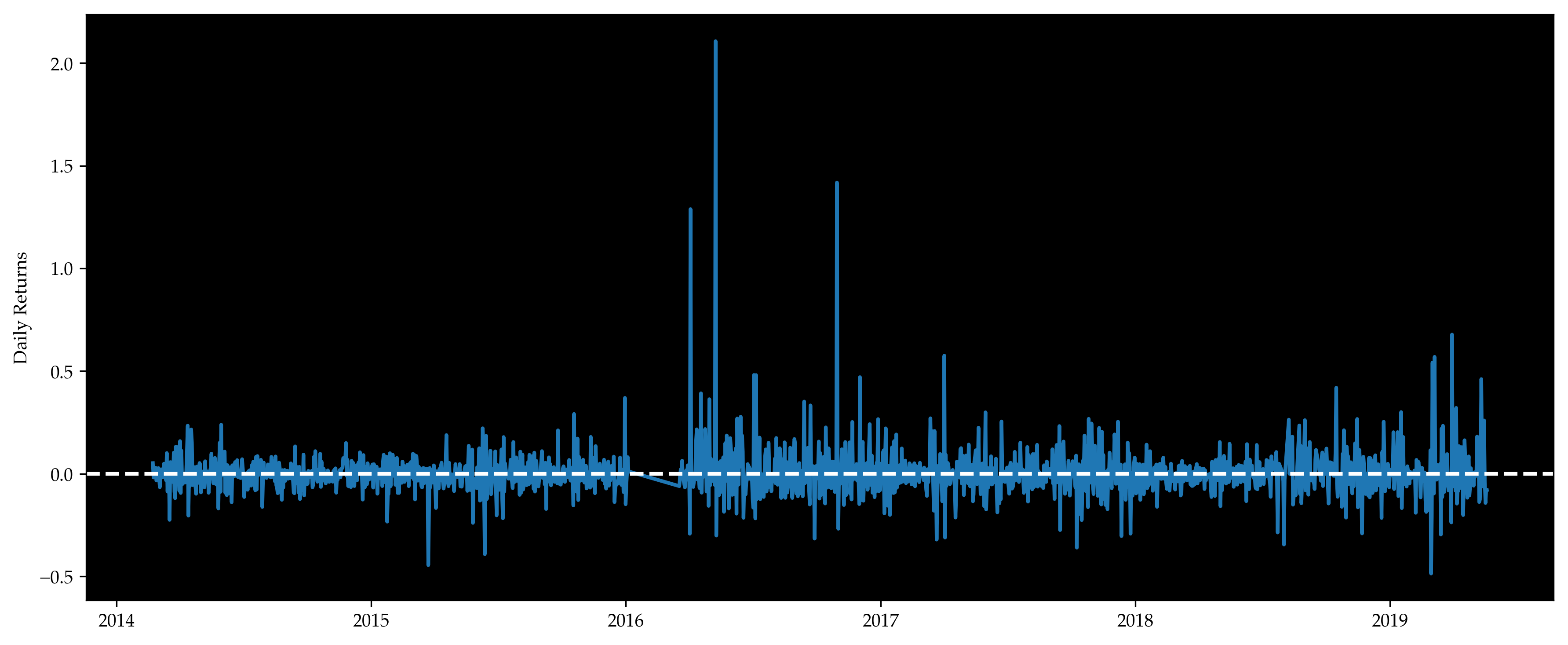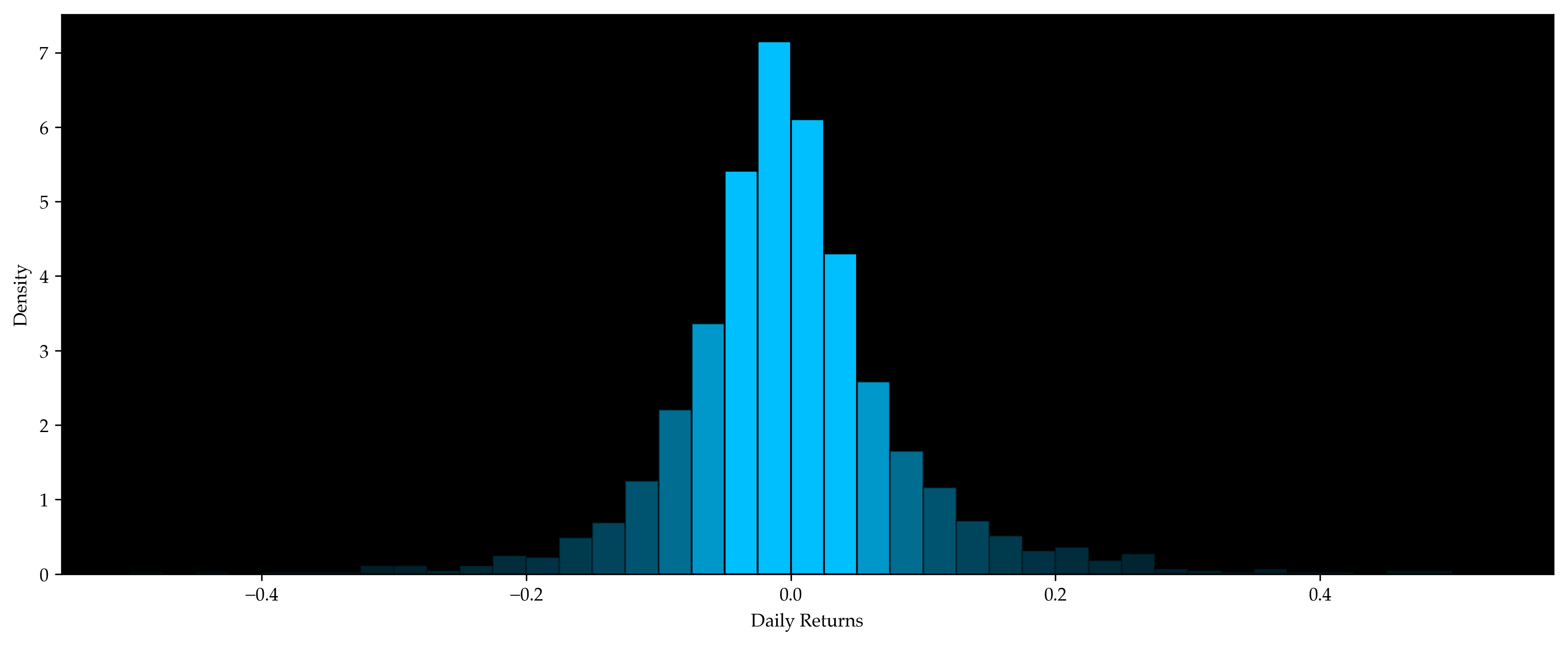datewordsrp
2014-02-24[able, able, abolished, absence, accept, accep...-0.0050150.650951
2014-02-25[abiding, abiding, able, able, abruptly, accel...0.0127760.842586
2014-02-26[abandoned, able, able, able, able, abrupt, ac...0.0289320.525475
............
2017-12-12[able, access, access, access, accessibility, ...-0.3037560.006084
2017-12-13[abort, accept, accept, accepts, according, ac...0.0082220.453232
2017-12-14[according, according, accounts, acknowledge, ...-0.0977810.657795
2017-12-17[accepted, accepting, access, accounts, accumu...0.0456900.218251

Daily returns are positive for about half of the time and negative for the rest of the time. They follow a normal distribution with mean near 0.

Relative Strength Index (RSI) is a momentum indicator that measures the magnitude of recent price changes. Typically, RSIs above 70 or below 30 are considered overbought or oversold. The plot below shows RSIs of the coins, the values lie in between 30 and 70 most of the time. From our understanding, it does not imply strong momentum, which also agrees with the return results above. Because of the dissatisfying return results, we are motivated to make use of news data to help us determine our positions in the portfolio formed by the momentum strategy.

dateBTCDOGEFTCIXCLTCMECNMCNVCNXTOMNIPPCXPMXRP
2014-02-2149.05892565.15668153.86209447.65772852.32511754.57070751.52160845.43396462.57456756.64880658.89383951.25664654.260447
2014-02-2249.52263764.59625353.46066648.03272252.78299556.67639551.59107045.00918362.06117557.09129458.42803051.13105354.255182
2014-02-2348.94537465.15146552.94146748.02557149.75273555.93832750.25713343.98155958.73226956.15698458.26090050.58173254.383604
2014-02-2445.48189765.83661549.93064246.87676946.76359848.20532848.23158942.01833157.44933053.39000656.61238047.97432350.894997
2014-02-2545.93416966.23641549.85953545.47823146.92895849.00676949.34394142.25040157.22446953.05403056.70391548.37762448.364130
..........................................
2019-05-1661.09126361.70276960.62652960.11983868.90886957.45171439.88225361.21946852.23564352.79205145.62913664.85557665.275916
2019-05-1757.88740861.33156559.91796359.27601367.44765358.05723340.32233559.04964050.48091553.29269845.26645364.39553662.578782
2019-05-1858.80867761.53214758.77337659.72852168.15176456.26493346.89927260.01012750.11385453.57957445.82841865.14112962.913106
2019-05-1961.60019061.39768259.77334859.82107669.69291357.51497748.67390462.44607150.53195052.96219549.98564765.18035965.565234
2019-05-2060.82270760.24991859.63788058.08759969.29162457.01320947.24322362.01282350.48778053.77719949.51287964.34597264.806905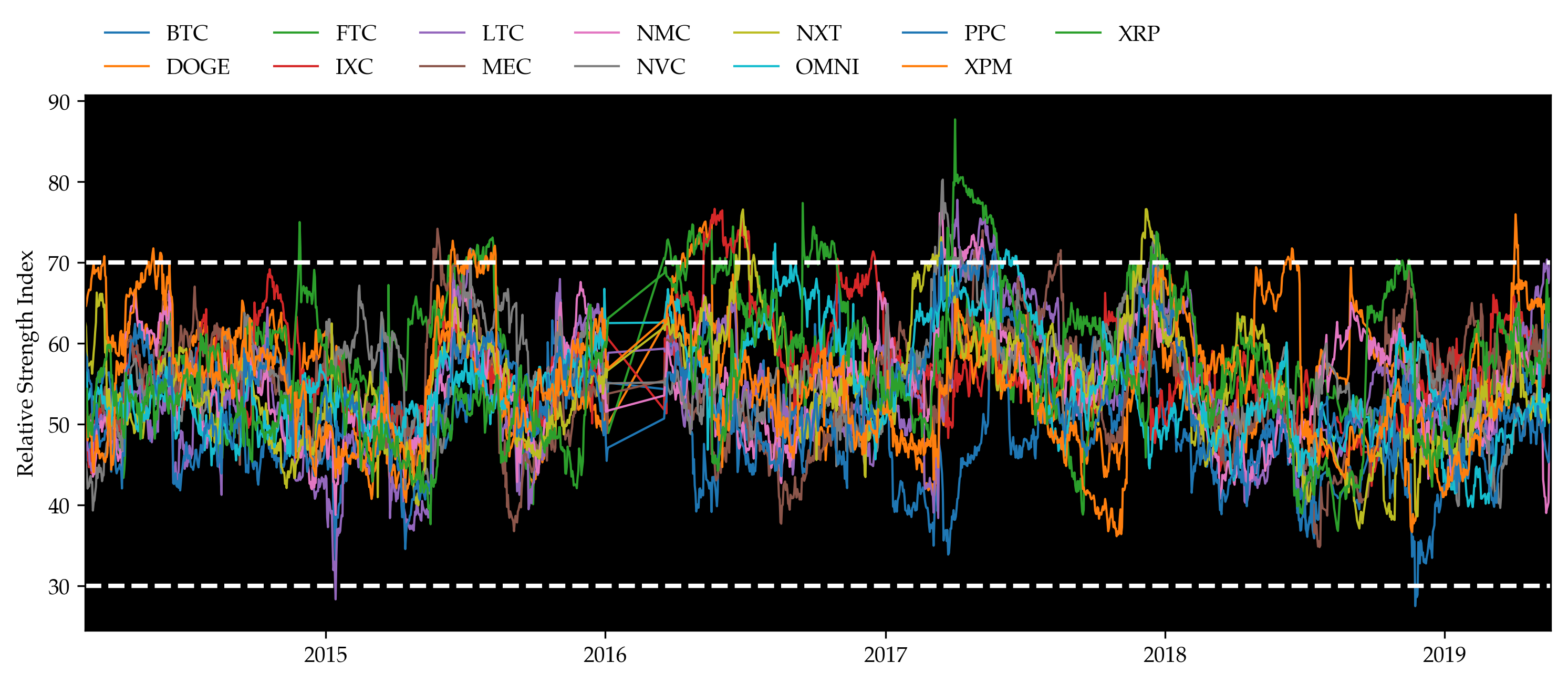Now we may finally start to implement the hybrid model. The algorithm is illustrated below. Instead of title or abstract, here we test on content directly. Contents contain more information than titles or abstracts only, thus enlarging our sample set.

Step 0: Calculate the percentage of positive returns on each word $$f_j$$. Filter words occurring too infrequently. Keep only the top $$\alpha_+$$ and bottom $$\alpha_-$$ as the sentiment-charged words. Assume we have in total $$k$$ such words.

wordalphapointkoreanmastersargentinawilson...mccalebatlascoinjarmintpalsteem
r0.1176470.1625000.1780820.1785710.187500...0.7547170.7966100.8169010.8269230.96
k51.00000080.00000073.00000056.00000064.000000...53.00000059.00000071.00000052.00000050.00
p0.0000000.0005160.0010320.0015480.002064...0.9979360.9984520.9989680.9994841.00

Step 1 (Training): For each date $$\newcommand{\bs}{\mathbf}\bs{d}_i = (d_{i,1}, d_{i,2},\ldots,d_{i,k})'$$ where $$d_{i,j}$$ is the count of sentiment-charged word $$w_j$$ in this day, assume model

$\bs{d}_i \sim \text{Multinomial}\left[k, \textstyle{\sum_{j=1}^{k}} d_{i,j}, p_i \bs{O}^+ + (1 - p_i) \bs{O}^-\right].$

Where $$\bs{O}^+$$ is a "positive sentiment day", and describes expected counts of words in a maximally positive sentiment day (one for which $$p_i$$ = 1). Likewise, $$\bs{O}^-$$ is a "negative sentiment day" that describes the distribution of word probabilities in maximally negative days (those for which $$p_i$$ = 0).

Calculate the rank scores of all days as $$p_i$$, then estimate $$\bs{O}^+$$ and $$\bs{O}^-$$.

There are 194 positive words, 194 negative words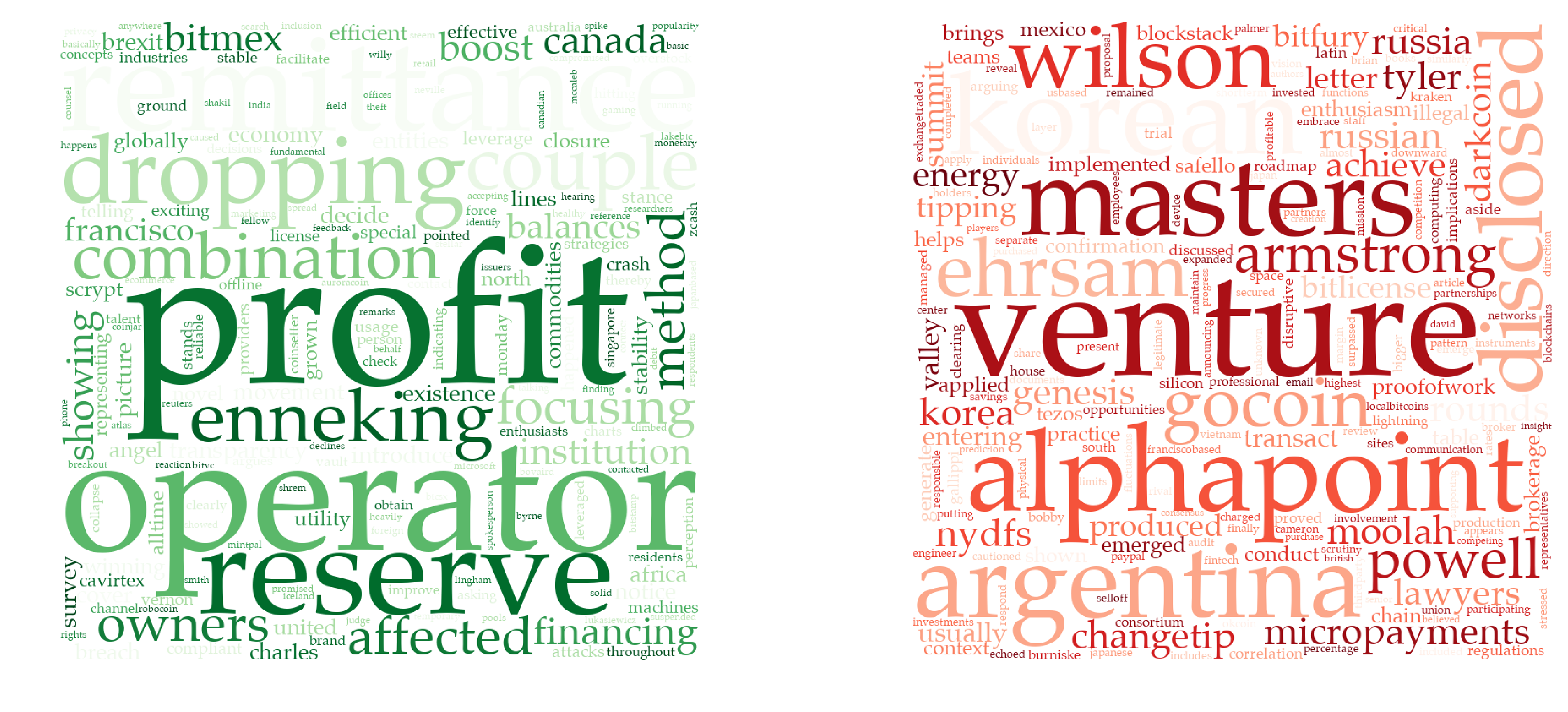The generated positive and negative word sets are shown above. The bigger the word appears, the more positive or negative it is. Let's look at some examples:

The left green diagram gives all the positive words with $$p_i > 1 - \alpha$$. The biggest word in the middle is "profit". Most articles containing it express an optimistic view. Examples include "generate a 150,000 profit in bitcoins", and "taking profit from legitimate miners". Other positives words include "boost", "financing", and etc. "Venture", which usually indicates risk, occupies the most space of the right red diagram. We cannot deny the fact that the relationship between words and returns may be specious. Problems also occur when words such as "not" negate the meaning of sentences. But overall, the classification of words works well with sufficient rationale behind. We can then estimate $$\bs{d}$$. datewordsrpd 2014-02-22[address, aggregators, along, amount, antinucl...0.0514960.961217[0, 0, 0, 0, 0, 0, 0, 0, 0, 0, 0, 0, 0, 0, 0, ... 2014-02-23[according, additional, akin, allocated, along...-0.0184200.236502[0, 0, 0, 0, 0, 0, 0, 0, 1, 0, 0, 0, 0, 0, 0, ... 2014-02-24[able, able, abolished, absence, accept, accep...-0.0050150.650951[0, 0, 0, 0, 0, 0, 0, 0, 0, 0, 0, 0, 1, 0, 0, ... 2014-02-25[abiding, abiding, able, able, abruptly, accel...0.0127760.842586[0, 1, 0, 0, 0, 3, 0, 0, 0, 0, 0, 0, 0, 0, 1, ... 2014-02-26[abandoned, able, able, able, able, abrupt, ac...0.0289320.525475[0, 2, 0, 0, 0, 0, 0, 0, 0, 0, 0, 0, 8, 0, 3, ... ............... 2017-12-12[able, access, access, access, accessibility, ...-0.3037560.006084[0, 1, 0, 0, 1, 0, 0, 0, 0, 0, 0, 0, 0, 0, 0, ... 2017-12-13[abort, accept, accept, accepts, according, ac...0.0082220.453232[0, 1, 0, 0, 0, 0, 0, 2, 0, 0, 1, 0, 0, 0, 0, ... 2017-12-14[according, according, accounts, acknowledge, ...-0.0977810.657795[0, 0, 0, 0, 0, 0, 0, 1, 1, 0, 0, 0, 0, 0, 0, ... 2017-12-15[action, ahead, already, altcoins, alternative...-0.0587290.178707[0, 0, 0, 0, 0, 0, 0, 0, 0, 0, 0, 0, 0, 0, 0, ... 2017-12-17[accepted, accepting, access, accounts, accumu...0.0456900.218251[0, 0, 0, 0, 0, 0, 0, 0, 0, 0, 0, 0, 1, 0, 0, ... Now we estimate $$\newcommand{\d}{\text{d}}\bs{O}^{+/-}$$ using $$\bs{d}$$ and $$p$$. Estimation by MLE: The likelihood is given by $L(\bs{d};\bs{O^{+/-}}) \propto \textstyle{\prod_i\prod_{j=1}^k} [p_i O_j^+ + (1 - p_i) O_j^-]^{d_{i,j}}$ and thus the log-likelihood is $LL(\bs{d};\bs{O^{+/-}}) = C + \textstyle{\sum_i \sum_{j=1}^k} d_{i,j} \log[p_i O_j^+ + (1 - p_i) O_j^-]$ where $$C$$ is not a function of $$\bs{O}^{+/-}$$. Therefore, for each keyword $$w_h$$, $\frac{\partial LL}{\partial O_h^+} =\textstyle{\sum_i \sum_{j=1}^k} \frac{d_{i,j}p_i\mathbf{1}\{j=h\}}{p_i O_j^+ + (1 - p_i) O_j^-} =\textstyle{\sum_i} \frac{d_{i,h}p_i}{p_i O_h^+ + (1 - p_i) O_h^-} > 0$ and $\frac{\partial LL}{\partial O_h^-} =\textstyle{\sum_i \sum_{j=1}^k} \frac{d_{i,j}(1-p_i)\mathbf{1}\{j=h\}}{p_i O_j^+ + (1 - p_i) O_j^-} =\textstyle{\sum_i} \frac{d_{i,h}(1-p_i)}{p_i O_h^+ + (1 - p_i) O_h^-} > 0.$ The optimization problem, therefore, is \begin{align*}\max_{\bs{O}^{+/-}}\ & LL(\bs{d};\bs{O}^{+/-})\\\text{s.t. } & \textstyle{\sum_{j=1}^k} O_j^+ = \textstyle{\sum_{j=1}^k} O_j^- = 1\\ & O_j^+ \ge 0 ,\quad O_j^- \ge 0,\quad \forall 1 \le j \le k.\end{align*} Instead of solving this problem using the Karush-Kuhn-Tucker Conditions, we may as well do the following transform: let $O_j^{+/-}:=\exp(Q_j^{+/-})$ so that the unknowns are now $$Q_j^{+/-}\in\mathbb{R}$$ and we no longer need any inequality constraints. The Lagrangian is $f(\bs{Q}^{+/-}) = LL\!\left[\bs{d};\exp(\bs{Q}^{+/-})\right] + \lambda^+\!\left[1 - \textstyle{\sum_{j=1}^k} \exp(Q_j^+)\right] + \lambda^-\!\left[1 - \textstyle{\sum_{j=1}^k} \exp(Q_j^-)\right]$ and the FOCs are $$2k$$ equations $0 = \frac{\partial f}{\partial Q_j^{+/-}} =\frac{\partial LL}{\partial O_j^{+/-}}\frac{\d O_j^{+/-}}{\d Q_j^{+/-}} - \lambda^{+/-} \frac{\d O_j^{+/-}}{\d Q_j^{+/-}}. \quad (1 \le j \le k)$ The FOCs can be simplified into $\frac{\partial LL}{\partial O_1^{+/-}} =\frac{\partial LL}{\partial O_2^{+/-}} =\cdots =\frac{\partial LL}{\partial O_k^{+/-}} = \lambda^{+/-}$ which is still extremely hard to solve analytically. Estimation by Regression: This method is proposed in Ke, Kelly and Xiu (2019). Assume there're in total $$\newcommand{\T}{\mathsf{T}}n$$ days. Define $\bs{W} = \begin{bmatrix}p_1 & p_2 & \cdots & p_n\\1 - p_1 & 1 - p_2 & \cdots & 1 - p_n\\\end{bmatrix}^\T$ and $$\bs{D} = \left(\hat{\bs{d}}_1, \hat{\bs{d}}_1, \cdots, \hat{\bs{d}}_n\right)^{\!\T}$$ where $$\hat{\bs{d}}_i = \bs{d}_i / \textstyle{\sum_j} d_{i,j}$$. Then $$\bs{O}^{+/-}$$ would be estimated by regressing $$\bs{D}$$ on $$\bs{W}$$, namely $\hat{\bs{O}}^{+/-} = (\bs{W}^\T \bs{W})^{-1} \bs{W}^\T \bs{D}.$ See proof of accuracy in the appendix of the paper. W has shape (921, 2): [0.96121673 0.03878327] [0.23650190 0.76349810] [0.65095057 0.34904943] ... [0.65779468 0.34220532] [0.17870722 0.82129278] [0.21825095 0.78174905] D has shape (921, 388): [0.00000000 0.00000000 0.00000000 ... 0.00000000 0.06666667 0.00000000] [0.00000000 0.00000000 0.00000000 ... 0.00000000 0.00000000 0.00000000] [0.00000000 0.00000000 0.00000000 ... 0.00000000 0.00000000 0.00000000] ... [0.00000000 0.00000000 0.00000000 ... 0.00000000 0.00000000 0.00000000] [0.00000000 0.00000000 0.00000000 ... 0.00000000 0.00000000 0.00000000] [0.00000000 0.00000000 0.00000000 ... 0.00000000 0.00000000 0.01219512] O± has shape (388, 2): [0.00188745 0.00293239] [0.00111679 0.00332143] [0.00349693 0.00450815] ... [0.00396731 0.00174460] [0.00407812 0.00318298] [0.00753035 0.00733650] Now that we have already estimated $$\bs{O}^{+/-}$$, we can start predicting out-of-sample $$p$$, which indicates the expected rank of performance of a certain day. We're solving $$p$$ from the following optimization problem $\hat{p} = \arg\!\max_{p\in[0,1]} \left\{\sum_{j=1}^k \hat{d}_j \log\!\left[p O_j^+ + (1 - p) O_j^-\right] + \lambda \log\left[p(1-p)\right] \right\}$ where $$\hat{\bs{d}}=\bs{d} / \textstyle{\sum_j}d_j$$ like defined above, and $$\lambda>0$$ is a hyper-parameter for tuning. Let's call it the penalized log-likelihood pen_ll. Tune $$\lambda$$ using training set: 3.6398125000000046 Step 2 (Testing): For each date $$\bs{d}_i$$ observed and $$\bs{O}^{+/-}$$ fixed, estimate $$p_i$$ as our final indicator. Specifically, we here use the best $$\lambda$$ we just estimated above to predict the testing set. datewordsrpp_hatd 2017-12-18[according, according, according, according, a...-0.1275510.2342210.313379[0, 0, 0, 0, 0, 0, 0, 0, 0, 0, 1, 0, 0, 0, 0, ... 2017-12-19[ability, able, able, able, abrupt, absolutely...-0.0052380.5612170.560449[1, 0, 0, 0, 0, 0, 1, 2, 0, 1, 1, 0, 0, 0, 1, ... 2017-12-20[able, able, abruptly, abruptly, accelerate, a...-0.0644550.5741440.343652[0, 0, 0, 1, 0, 1, 1, 0, 0, 2, 0, 0, 0, 0, 0, ... 2017-12-21[able, according, according, according, accoun...0.1515030.1718630.313184[0, 0, 0, 0, 0, 0, 0, 0, 0, 0, 0, 1, 0, 0, 0, ... 2017-12-22[able, abound, acceptance, accepting, acceptin...0.0833990.7680610.340234[4, 1, 0, 0, 0, 0, 0, 0, 0, 0, 2, 0, 0, 2, 0, ... .................. 2019-04-26[access, according, according, according, acco...-0.0587120.4669200.470312[1, 0, 0, 0, 0, 1, 0, 0, 0, 0, 0, 0, 0, 0, 0, ... 2019-04-29[abort, access, according, according, accordin...-0.0410620.9634980.416309[0, 0, 0, 0, 0, 0, 0, 0, 0, 1, 0, 0, 0, 0, 0, ... 2019-04-30[able, able, acceptance, acceptance, access, a...-0.0110060.5330800.628125[0, 1, 0, 0, 0, 0, 1, 1, 0, 0, 0, 0, 0, 0, 0, ... 2019-05-01[access, access, accessible, according, accord...-0.0586200.9794680.484668[1, 1, 0, 0, 0, 0, 1, 0, 0, 2, 0, 0, 0, 0, 0, ... 2019-05-02[able, aborted, access, accompanied, according...0.0066810.3741440.445898[0, 0, 0, 0, 0, 0, 3, 0, 0, 0, 1, 0, 0, 0, 0, ... ### Backtesting Results ↩︎ Finally, given the predicted p_hat values, we can trade based on certain sizing functions and calculate the cumulative return as well as all other trading related statistics of our strategy. We will also calculate these for benchmark strategies. A short description of benchmark strategies and our hybrid sentiment-momentum strategy: • Buy-and-hold: This strategy simply buys Bitcoin and hold for the whole trading period. Bitcoin is the most commonly traded coin in the cryptocurrency market and is representative of the market. Thus, we choose Bitcoin as the asset we buy and hold. • Momentum: As explained above, the momentum strategy takes long and short positions separately in the top n and bottom n coins. Here, by "top" and "bottom", we mean the best- and worst-performing coins in the look-back window of d trading days. Specifically, we take n=3 and d=60. • Momentum Reversal: The momentum reversal strategy does exactly the opposite of the momentum strategy. It takes short positions in the top n coins and long positions in the bottom n coins. The definition and values of n and d remain the same. • Hybrid Sentiment-Momentum: Our hybrid sentiment-momentum strategy combines sentiment analysis and momentum trading strategy. Using the core idea of momentum, the strategy ranks all the coins based on their previous price changes and then forms a portfolio that enters long positions in coins that headed up and short positions in those that went down. This step is the same as a usual momentum trading strategy. Taking advantage of the sentiment analysis results on present da's news, the strategy then decides on how many units of the portfolio to buy or sell.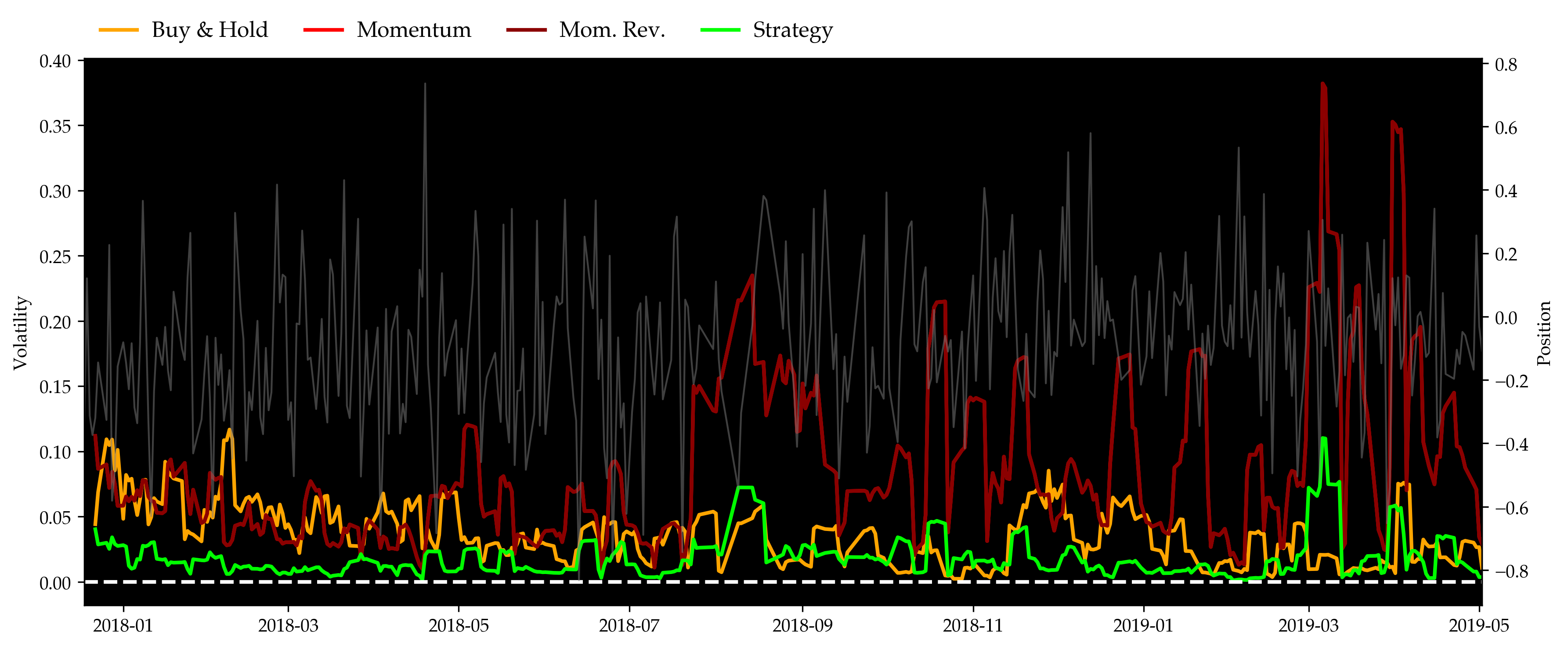StatisticsBuy & HoldMomentumMom. Rev.Strategy Win%0.5240510.4379750.5620250.536709 Sharpe-0.975205-0.6053310.6053312.677082 MDD0.8264190.9709560.9479630.206906 As can be seen in the figure above, the momentum strategy performed almost as poorly as a simple buy & hold throughout the bearish year of 2018. The momentum reversal strategy, on the other hand, performed extremely well at the first half year and then collapsed with the market. The partially reflects the existence of price overreactions as suggested by Caporale and Plastun (2018). Our hybrid strategy, on the other hand, outperformed all of them by over 100% cumulative returns by the end of backtest, and gave a Sharpe ratio of 2.68 when best of the rest, which is given by momentum reversal, is merely 0.60 or so. Volatility plot also shows that by adding sentiment analysis to determine position sides and sizes of the portfolio, the hybrid strategy significantly reduces the risks that existed in the momentum strategy. Also, considering the maximum drawdowns, all of the three benchmarks gave gigantic drawdowns in this unfortunate year, while our strategy's was well limited under 20% most of the time. ### Model Sensitivity to Parameters ↩︎ The following parameters are included in our model. We want to test how the model reacts towards changes in them. • lookback_window is the number of days we feed our model for training, • minimum_occurrence is the minimum number of occurrences for a word to be considered sentiment-charged, • minimum_length is the minimum acceptable length of a word to be considered sentiment-charged, • alpha is the top (and bottom) percentile for sentiment-charged word selection • size is a univariate function mapping $$[0,1]\subset\mathbb{R}$$ to $$[-1,1]\subset\mathbb{R}$$.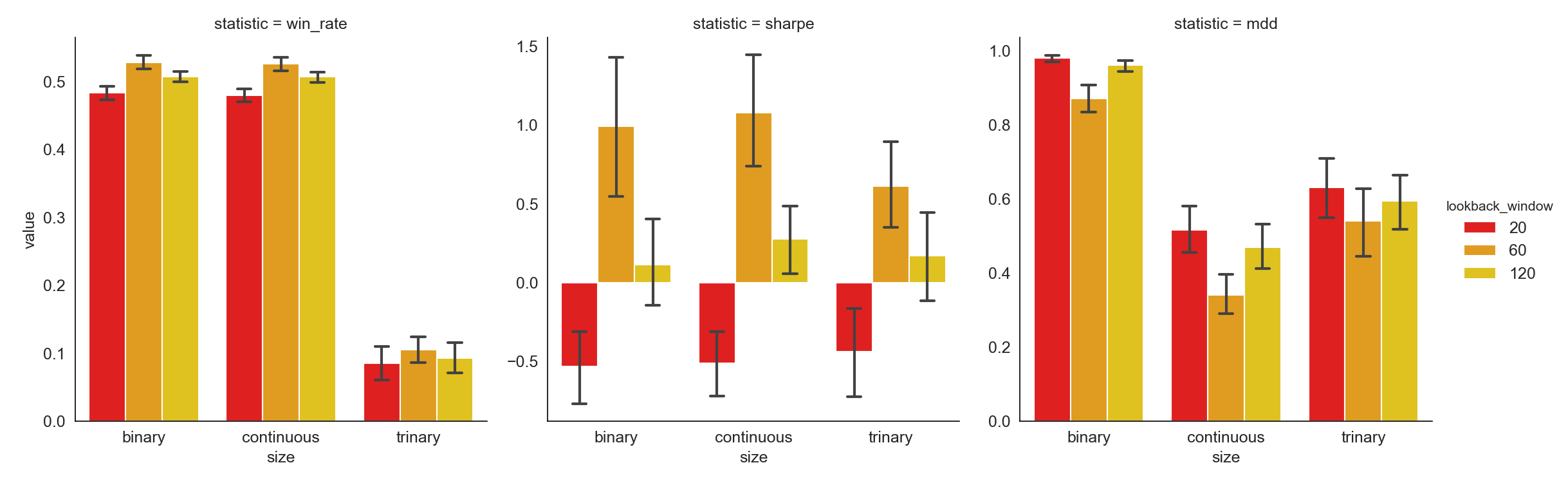A longer look-back window provides more information in the past that can be used to help decision-making on forming the portfolio. However, the longer the window, the more stale information is included. The empirical result tells us using past 60 day' information to determine the best and worst performing coins eventually leads to the highest Win rate and Sharpe Ratio together with the lowest Maximum Drawdown.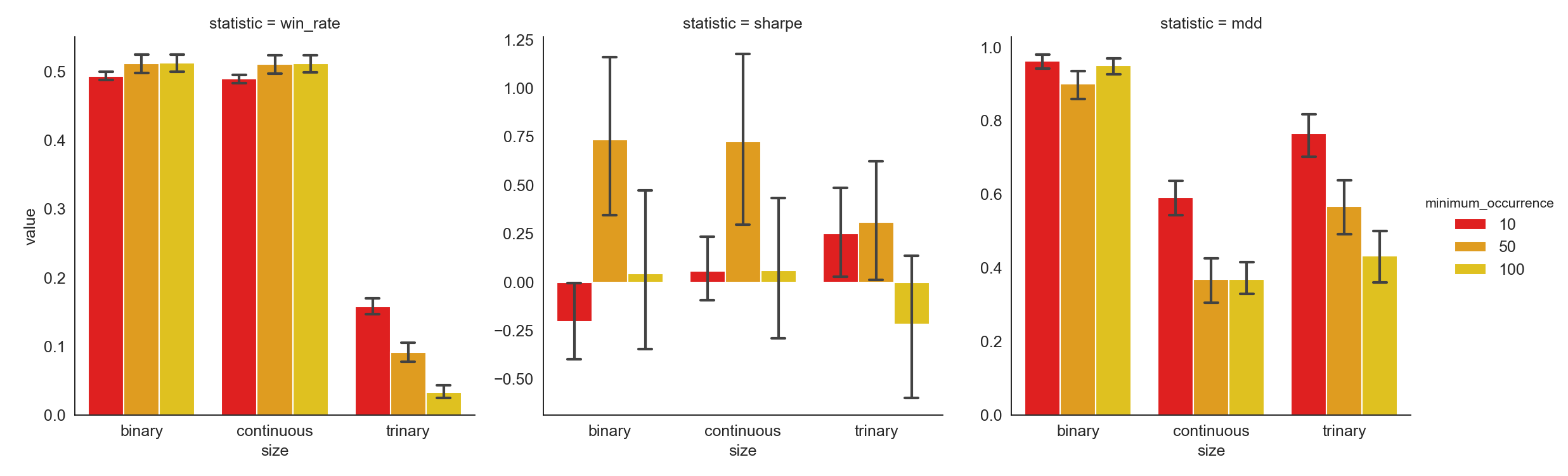Higher minimum occurrence implies a stricter constraint on selecting sentiment-charged words. While noises coming from words which do not show up frequently are diminished, if minimum occurrence is set too high, some useful information is left out. Moreover, a very small pool of sentiment-charged words will cause the training and predicting to be extremely hard and even impossible. From the results, we can conclude that setting minimum occurrence equal to 50 generates better Sharpe Ratio and Maximum Drawdown than setting it to 10 or 100.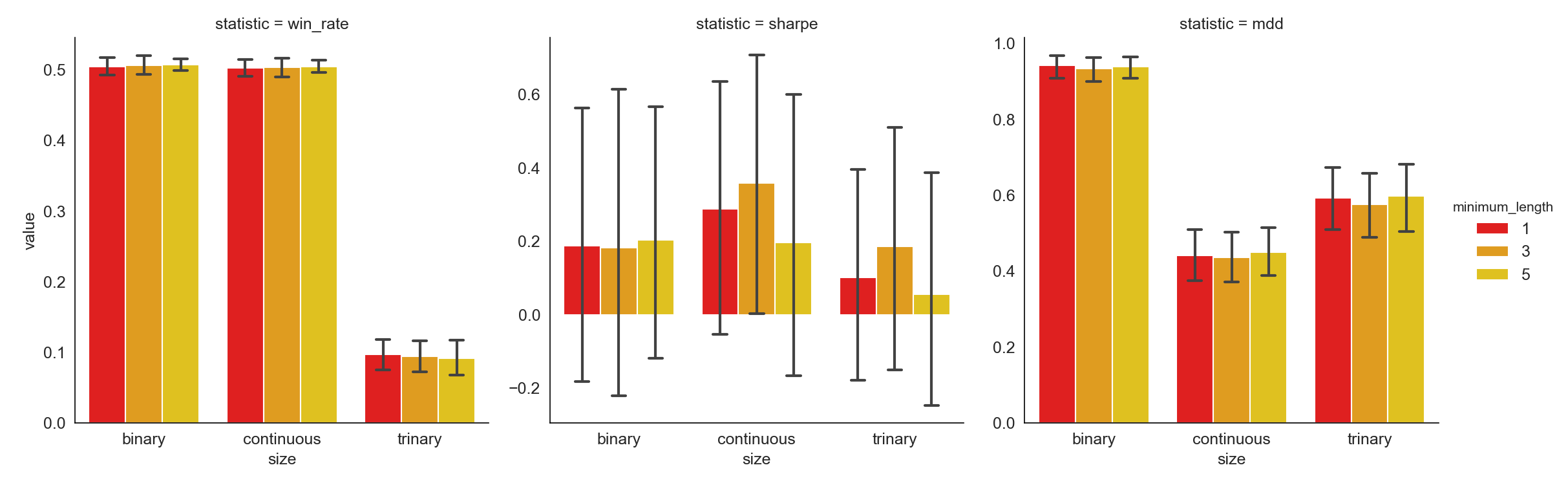Many of the short words, those whose lengths are 2 or 3, are not really informative, thus conveying nearly no sentiment. For instance, words such as "a", "is", and "the" show up in almost every piece of news multiple times. Whereas they don't offer much help in understanding the sentiment of the news, so they can be eliminated without hurting the results. Of course, this simple criterion is arbitrary, thus needs to be tested. Based on the results we get, different minimum length values don't impact the strategy statistics significantly.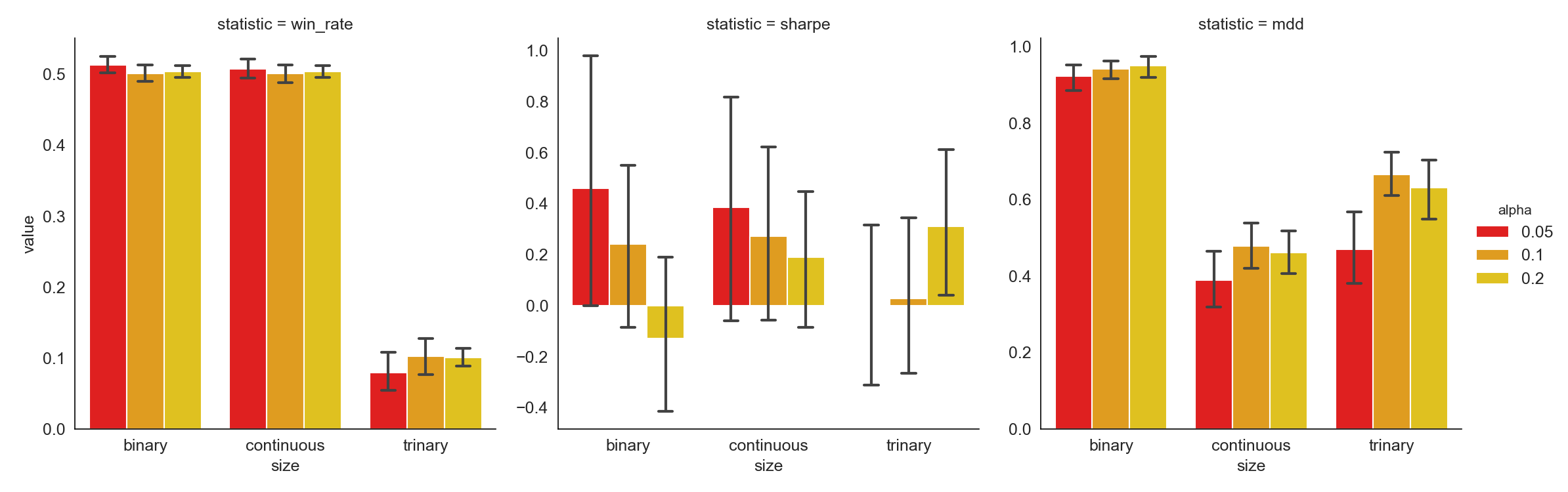Alpha is used to define the percentage of sentiment-charged words that are positive or negative. Similar to minimum occurrence, a strategy with a larger alpha includes more information into the analysis and vice versa. An alpha of 0.05 gives the best results as shown above. For sizing function: • Binary: if $$p$$ is greater than $$0.5$$, we take $$1$$ long position; otherwise, we take $$1$$ short position. • Continuous: we always take $$2p-1$$ positions, since $$p\in[0,1]$$, we know $$2p-1\in[-1,1]$$. • Trinary: if $$p$$ is greater than ⅔, we take $$1$$ long position in the portfolio; it $$p$$ is less than ⅔ but greater than ⅓, we don't take any position; otherwise, we take $$1$$ short position. Trinary has much worse performance than binary and continuous as both Win rate and Sharpe ratio are worse for Trinary than for the other two. While continuous and binary are similar on Win rate and Sharpe ratio, continuous always has smaller Maximum Drawdowns. Therefore, it is a more stable strategy and is thus best for risk-averse investors. For a more comprehensive practice of hyper-parameter exploration, we summarized the scripts above into a local module backtest for convenient backtesting. The general usage is summarized as below: See Appendix C for the complete code and the detailed parameter testing outputs. ### Risk Management ↩︎ Momentum trading strategy is essentially buying on gainers and selling on losers. Due to the low liquidity and slow information dissemination nature of cryptocurrency trading, trading on momentum is, theoretically speaking, profitable. However, there is no guarantee that what makes gains will continue being profitable and what generates losses will keep losing money. Therefore, a risk management technique to prevent the strategy from taking huge amount of unexpected losses is a must-have with no doubt. One of the most common approaches to controlling risks is to include a stop-loss feature. A usual way to apply a stop-loss is to calculate returns, determine if it falls below the threshold and then exit the position if it does. Here is where our strategy differs. It does not need an additional stop-loss as a stop-loss feature is embedded in the strategy already. The reason is that the underlying momentum strategy invests in a dynamic portfolio, meaning that all the positions the strategy enters on the previous day will be closed today no matter how the performance is on the previous day. In other words, the momentum strategy does not pick the same set of coins to invest from day to day, previous losses will not accumulate in following days. ## Conclusion ↩︎ In this research paper, we tested momentum and momentum reversal strategies in the cryptocurrency market and improved the traditional momentum strategy by introducing a brand-new sentiment-based model. The model significantly improved the general strategy scenario. It turned out that our hybrid model can handle massive market collapses e.g. mid-2018 while taking valid non-zero positions, and can grasp profits at potential opportunities. There are, however, still several limitations in this research. First, due to the limited history of cryptocurrency, our dataset is rather short compared with stock data, with only 2014 - 2019 making up both training and testing sets. This limitation does not only occur w.r.t. time, but also troubles us during dynamic coin selection. Since we need a long period of historical data, our choices of coins became considerably restricted (less than 20), which further causes us to hold less (to be precise, 6 in this case) coins at each day. Idiosyncratic risks may not be well diversified and there is non-negligible likelihood that our strategy fails us because of failure in one certain coin -- though luckily, we didn't encounter this issue. Last but not least, more rigorous backtests could be implemented to perform against our strategies. We may use intra-day data, if possible, for better accuracy. Furthermore, we can take order book into account to cope with micro-structure related concerns. By and large, our hybrid strategy satisfyingly outperformed all benchmark strategies in the same market and we deem this as a strong and good result. ## References ↩︎ • Antonacci, Gary (2014). Dual Momentum Investing: An Innovative Approach for Higher Returns with Lower Risk. New York: McGraw-Hill Education, 13–18. ↩︎ • Rohrbach, J., Suremann, S., & Osterrieder, J. (2017). Momentum and Trend Following Trading Strategies for Currencies Revisited-Combining Academia and Industry. ↩︎ • Ke, Z. T., Kelly, B. T., & Xiu, D. (2019). Predicting Returns With Text Data. Available at SSRN. ↩︎ • Caporale, G. M., & Plastun, O. (2018). Price Overreactions in the Cryptocurrency Market. ↩︎ ## Appendix ↩︎ We include all miscellaneous scripts (mostly for data crawling) as below. ### Appendix A: News Data ↩︎ ### Appendix B: EOD Data ↩︎ Now we starting generating new features from these posts. First let's take a look at the data. ### Appendix C: Backtest Engine and Hyper-Parameter Testing ↩︎ In this section, we give the full code of our backtest engine as well as the full list of hyper-parameter testing results. The scripts for engine is as below. The hyper-parameter testing, using the backtest engine above, is listed as follows. #lookback_windowminimum_occurrenceminimum_lengthalphasizestrategywin_ratesharpemdd 0201010.05binaryBuy & Hold0.527094-0.4417380.831663 1201010.05binaryMomentum0.423645-1.6869620.998461 2201010.05binaryMom. Rev.0.5763551.6869620.781847 3201010.05binaryStrategy0.482759-0.5216450.988099 4201010.05continuousBuy & Hold0.527094-0.4417380.831663 .............................. 96712010050.20continuousStrategy0.494709-0.8310740.582280 96812010050.20trinaryBuy & Hold0.526455-0.9714760.789994 96912010050.20trinaryMomentum0.433862-0.0603130.925483 97012010050.20trinaryMom. Rev.0.5661380.0603130.987388 97112010050.20trinaryStrategy0.047619-0.9886540.665146 #lookback_windowminimum_occurrenceminimum_lengthalphasizestatisticvalue 0201010.05binarywin_rate0.482759 1201010.05continuouswin_rate0.470443 2201010.05trinarywin_rate0.172414 3201010.10binarywin_rate0.514778 4201010.10continuouswin_rate0.512315 ........................ 72412010050.10continuousmdd0.376395 72512010050.10trinarymdd0.407608 72612010050.20binarymdd0.996307 72712010050.20continuousmdd0.582280 72812010050.20trinarymdd0.665146 ]]> <p>This is an exploratory research trying to implement a simple yet robust sentiment analysis mechanism in the cryptocurrency market. We call it a hybrid sentiment-momentum strategy as it applies context analysis (or more specifically, word analysis) into traditional momentum strategies and adjusts position automatically over time using both factors. The hybrid strategy is implemented with backtesing period from Dec. 17th 2017 to May 2nd 2019. It yields cumulative return of 229% and a remarkable Sharpe ratio of 2.68 with acceptable maximum drawdown in out-of-sample backtest, which is a huge improvement from classical momentum strategies in our analysis.</p> <p><img src="/images/cryptonews_56_0.png" style="width: 100%;"></p> Stock Price Simulation http://allenfrostline.com/2019/05/10/stock-price-simulation/ 2019-05-10T19:17:22.000Z 2019-05-11T05:21:22.723Z In this post, I'll introduce four stochastic processes commonly used to simulate stock prices. Formulation and Python implementation are presented one by one, with brief comments afterwards.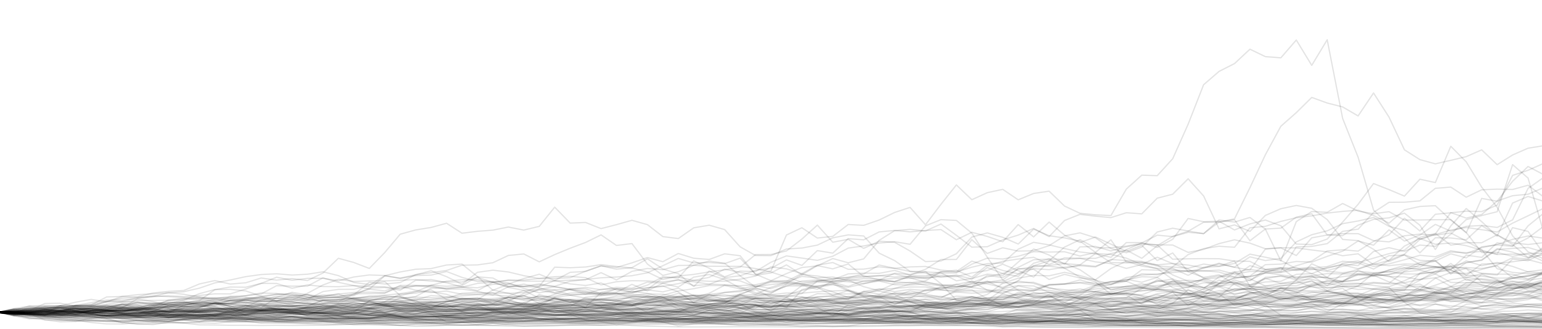Before introducing the four methods, we first define a handy function to prepend a zero before a numpy array: # Brownian Motion Brownian motion (BM) was initially exhibited and modeled in gas or liquid particle movements. The random motion was the solution to massive number of tiny collisions of particles in certain medium. Brownian motion was named after the botanist, Robert Brown, in 1827, and after almost a century introduced into the financial markets. People usually call a standard Brownian motion as a Wiener process, which has the following properties: • $$W_0\overset{\text{a.s.}}{=}0\newcommand{\d}{\text{d}}$$ • $$W$$ has independent increments $$\forall t > 0$$ • $$W$$ has Gaussian increments: $$W_{t + \d t} - W_t \sim \mathcal{N}(0,\d t)$$ • $$W_t$$ is continuous in $$t$$ with probability $$1$$ A general BM follows SDE: $\d S_t = \mu \d t + \sigma \d W_t$ which directly gives solution by take integrals on both sides: $S_t = S_0 + \mu t + \sigma W_t.$ With all of these given, we can easily simulate the process as below. Comments: One may notice that we don't have any constraint posed on $$W$$ — it can be however positive — and however negative. Therefore, while BM might give a simple and easy-to-implement solution for a short run, we don't really want to use it as it potentially gives us negative prices. # Geometric Brownian Motion Geometric Brownian Motion (GBM) was famous as been used in Fisher Black and Myron Scholes's 1973 paper, The Pricing of Options and Corporate Liabilities. The process is by definition positive and thus gives a fix for what we doubted in BM. The corresponding SDE is $\d S_t = S_t(\mu \d t + \sigma \d W_t)$ which gives solution $S_t = S_0\exp\left\{\left(\mu-\frac{\sigma^2}{2}\right)t + \sigma W_t\right\}.$ Comments: The GBM is good enough for most simulations. However, it is also well-known that the Black-Scholes model cannot give fat tails as is inspected empirically in stock markets. # Merton's Jump Diffusion Process Robert C. Merton, who shared the 1997 Nobel Price with Scholes (Black had passes away unfortunately), was one of the first academics to address some of the limitations in the GBM. In his 1976 paper, Option Pricing when Underlying Stock Returns are Discontinuous, he superimposed a "jump" component on the diffusion term so that the model can now simulate sudden economic shocks, i.e. jumps in prices. The jump $$J$$ is given by the exponential of a compound Poisson process $$N$$ with normal underlyings. The SDE is as follows. \begin{align*}Y_i&\overset{\text{i.i.d.}}{\sim}\mathcal{N}(\gamma, \delta^2)&\text{(Jump Magnitude)}\\\d N_t & \sim \text{Pois}(\lambda \d t)&\text{(Poisson Process)}\\J_t &= \textstyle{\sum_{i=1}^{N_t}}Y_i&\text{(Jump)}\\\d S_t &= S_t (\mu \d t + \sigma \d W_t + \d J_t).\\\end{align*} Merton's jump diffusion SDE has a closed-form solution: $S_t = S_0 \exp\left\{\left(\mu -\frac{\sigma}{2}\right)t + \sigma W_t + J_t\right\}.$ Comments: Merton's jump process solved the kurtosis mismatch problem in empirical financial data by minimally changing the GBM. However, with the discontinuous (and usually negative, corresponding to market clashes) jumps introduced in the model, we may witness frequent slumps and in general a decline in the total drift. On the other, the jump process still did not solve the constant volatility issue. # The Heston Stochastic Volatility Process In the early 1990's Steven Heston introduced this model where volatilities, different from the original GBM, are no longer constant. In the Heston model, volatilities evolve according to the Cox-Ingersoll-Ross process with a mean-reverting essense. As there're now two stochastic processes, we need two (potentially correlated) Wiener processes. The SDE is now \begin{align*}\d W_t^S\d W_t^V &= \rho\d t & \text{(Correlated Wiener)}\\\d V_t &= \kappa (\theta - V_t) \d t + \xi \sqrt{V_t} \d W_t^V &\text{(Cox-Ingersoll-Ross)}\\\d S_t &= \mu S_t \d t + \sqrt{V_t}S_t \d W_t^S & \text{(Heston)}\end{align*} Comments: The Heston model is one of the most popular stochastic volatility models in finance. In case one need even more freedom, he may opt for time-varying parameters e.g. $$\mu\to\mu_t$$ and $$\xi\to\xi_t$$. # Plots Finally, let's take a look at all the simulated price processes. The Brownian motion is shifted s.t. $$S_0=1$$ like the rest models. Mutual parameters like $$\mu$$ and $$\sigma$$ are set to the same values. Each figure shows $$1000$$ paths.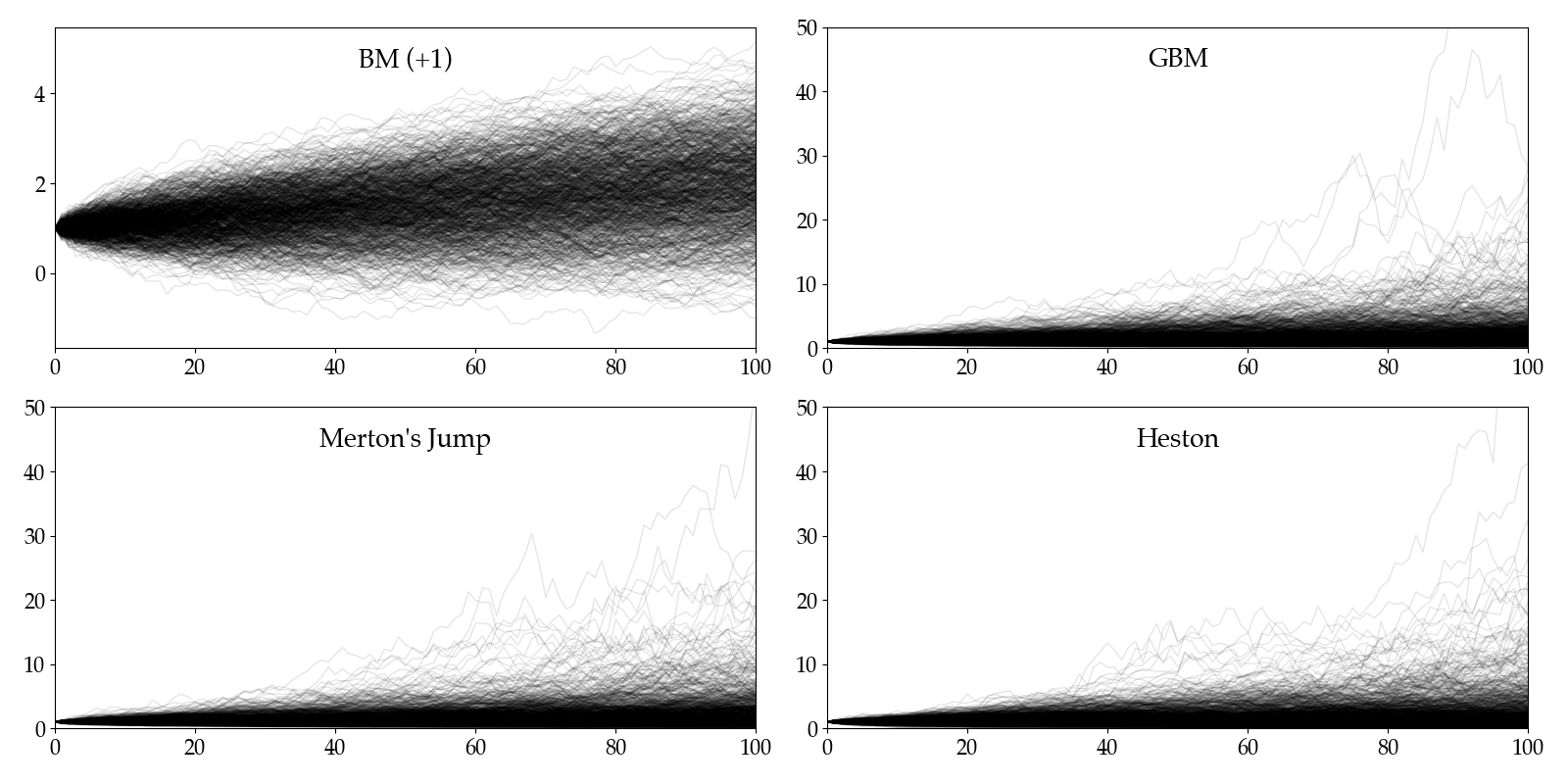]]> <p>In this post, I'll introduce four stochastic processes commonly used to simulate stock prices. Formulation and Python implementation are presented one by one, with brief comments afterwards.</p> <p><img src="/images/price_sim1.png"></p> Content Filtering with Hexo Tipue-Search Engine http://allenfrostline.com/2019/05/03/content-filtering-tipue-search/ 2019-05-03T18:40:57.000Z 2019-05-03T18:58:55.550Z It's always been a headache to me that I cannot have my blog's search engine to show content I want — there're always something you don't want 'em to show up in a search result, like password protected posts (shown as encrypted codes) and random pages for a certain project (some even don't have a title, and this tipue-search would still show them in the searching result — with a blank title and a bunch of html raw codes). Even worse, it seems there's no offical way to set this sort of content filters. This feels bad. This terrible feeling has tortured me for months till I made up my mind and fixed it from source codes today. The fix turned out, well, quite straightforward. First, we locate the node package folder hexo-generator-tipue-search-json. The package structure shows node_modules└───hexo-generator-tipue-search-json ├───index.js ├───LICENSE ├───package.json ├───README.md └───node_modules └───... The file we need to edit is index.js. Below I've attached the full codes after modification: Note the second line of the definition of postsContent and the lines we comment out. These modifications are made such that encrypted posts and standalone pages won't be searched. ]]> <p>It's always been a headache to me that I cannot have my blog's search engine to show content I want — there're always something you don't want 'em to show up in a search result, like <a href="https://www.npmjs.com/package/hexo-blog-encrypt" target="_blank" rel="noopener">password protected</a> posts (shown as encrypted codes) and random pages for a certain project (some even don't have a title, and this tipue-search would still show them in the searching result — with a blank title and a bunch of html raw codes). Even worse, it seems there's no offical way to set this sort of content filters. This feels bad. This terrible feeling has tortured me for months till I made up my mind and fixed it from source codes today.</p> Revert GitHub Commit History? http://allenfrostline.com/2019/05/03/revert-github-commit/ 2019-05-03T18:28:04.000Z 2019-05-03T18:40:17.606Z There is a piece of nicely given advice I'd like to share with you: do never post anything too large on your Hexo blog. The suggestion given above was a joke to me until yesterday. I thought I can just wait for some more minutes and then everything will be fine. Pages will end up posted with probability one in the long run — well, they didn't make it this time. The html files were so big that GitHub returned a file oversize error and rejected my push from Hexo. Everything got messy and however I tried, deployment was always rejected. If you also encounter this problem, well, lucky you, cause I've managed a fix to it. The first step would be locating the .git folder in our hexo directory. Here I used the builtin command under the hexo folder. The corresponding location was hexo/.deploy_git. Then we enter this directory and go onto our step 2, which is basically git commit history reversion. First go to the GitHub website and find an earlier commit and copy its SHA codes. Then, in the terminal we opened, enter: and then make a regular push. ]]> <p>There is a piece of nicely given advice I'd like to share with you: <strong>do never post anything too large on your Hexo blog.</strong></p> Animation in Matplotlib? http://allenfrostline.com/2019/04/25/animation-matplotlib/ 2019-04-25T23:27:01.000Z 2019-04-25T23:42:28.075Z In this tiny piece of post I'm gonna post how you can make animations using the matplotlib module in Python. Things get much more intuitive when they move, don't they? We're here trying to plot two (thick) sine curves which have different offsets on the x-axis direction, and those offsets increases with an indicator called frame_no. The plot is altered bit by bit as frame_no changes with frames. You can also try different fps configurations by changing the interval argument. Also, repetition is set true by default, but you may still forbid it by specifying repeat as false in FuncAnimation. Finally, you would have a plot as follows: Lovely, isn't it? ]]> <p>In this tiny piece of post I'm gonna post how you can make animations using the <code>matplotlib</code> module in Python. Things get much more intuitive when they move, don't they?</p> How to Get Variable Name as a String in Python http://allenfrostline.com/2019/04/23/var-name-python/ 2019-04-23T23:36:40.000Z 2019-04-23T23:47:04.862Z Although it's not recomended, people sometimes need the variable names. For example, you want to automate the process of generating a dictionary with variable names as keys, or use variable names as columns names in a pandas dataframe. How are we gonna implement this in Python? There is a nasty workaround provided somewhere on Stackoverflow (sorry but I forgot the actual thread): foofarvarName The method utilized the fact that Python stores all variables in the global() dictionary where keys are corresponding id values. Enjoy coding 🙃 ]]> <p>Although it's not recomended, people sometimes need the variable names. For example, you want to automate the process of generating a dictionary with variable names as keys, or use variable names as columns names in a pandas dataframe. How are we gonna implement this in Python?</p> The Perfect Market Maker: Is it Possible? http://allenfrostline.com/2019/04/23/perfect-market-maker/ 2019-04-23T06:02:36.000Z 2019-04-23T06:34:27.833Z In this research report we try to simulate and explore difference scenarios for a "perfect" market maker in the Bitcoin market. By "perfect" we refer to the capability to capture all spreads on the right side of any trade, i.e. there will be no spread loss at all. Although this setting is too perfect to be considered comparable with real trading, our analysis w.r.t. model parameters are believed to be insightful still. # Highlights • Self-explanatory backtest engine: the backtest engine is well encapsulated with limited public functions for people with little coding knowledge. • High-speed simulation: simulation per each set of parameters costs only ~2 ms without altering trading details. • Illustrated analysis: most analysis after parameter tuning is carried out with multiple (yet necessary) figures and elaborate explanation. Certain figures are made into animation for better understandability. # Dependencies Import necessary modules and set up corresponding configurations. In this research notebook, we are using the following packages: • numpy: mathematical tools & matrix processing • pandas: data frame support • matplotlib: plotting • ipython: statistical analysis • numba: accelerating pure numerical calculation • ffmpeg: animation support (unnecessary for the rest of codes) # Auxiliary Functions In this section, several useful functions are introduced for later use during the backtest. • cumsum: It's a modified version of the original cumulative summation function, the summation now handles two boundaries during calculation. Calculation is accelerated by JIT. • sharpe_ratio: It's a handy function that calculates the annualized Sharpe ratio based on high-freq returns (returns are defined in percentage). • sortino_ratio: Similar as above, the function gives the annualized sortino ratio. # Backtest Engine A backtest engine is designed for this problem. ### Parameters We have the following parameters (the first two are datasets) for simulation: • $$s$$: target transaction size • $$j$$: max long position (in Bitcoin) • $$k$$: max short position (in Bitcoin) Trade are participated only if: • The trade price is at the current best bid or offer price, • Trade quantity $$q>4s$$ • The new position $$x$$ satisfies $$−k \le x \le j$$ ### Usage Specially, the class provides a great feature that prints the whole backtest process in an animation. In order to activate the feature, run command below The BacktestEngine has been accelerated largely thanks to the vectorization and JIT features. Per each loop the calculation performance is shown as below (~2 ms per be.run). 2.11 ms ± 106 µs per loop (mean ± std. dev. of 7 runs, 100 loops each) # Preparatory Analysis In this part we try to load a small dataset and compare our results against the one in reference. The order book is as below. timeask1priceask1sizeask2priceask2sizeask3priceask3sizebid1pricebid1sizebid2pricebid2sizebid3pricebid3size 2018-04-08 17:08:00.2467035.5566.0623397035.560.57035.571.505877035.545.5829177035.530.001427035.50.011361 2018-04-08 17:08:01.4267035.5566.0623397035.560.57035.571.505877035.545.5843177035.530.001427035.50.011361 2018-04-08 17:08:08.2937035.5565.9589397035.560.57035.571.505877035.545.5843177035.530.001427035.50.011361 2018-04-08 17:08:08.4377035.5565.9589397035.560.57035.571.505877035.545.5708607035.530.001427035.50.011361 2018-04-08 17:08:08.4857035.5565.9589397035.560.57035.571.505877035.545.5912427035.530.001427035.50.011361 The trade data is as below. timepricesize 2018-04-08 17:08:08.2937035.550.1034 2018-04-08 17:08:13.4727035.540.3900 2018-04-08 17:08:19.1057035.550.1502 2018-04-08 17:08:20.8587035.540.0630 2018-04-08 17:08:23.0877035.540.1030 Now, the backtest result is as below. Here we use $$s=0.01$$, $$j=0.055$$ and $$k=0.035$$ like in the reference. We conclude that our model is valid as the result coincides with the one given. timetradecashposition 2018-04-08 17:08:08.293-0.0170.3555-0.01 2018-04-08 17:08:13.4720.010.00010.00 2018-04-08 17:08:19.105-0.0170.3556-0.01 2018-04-08 17:08:20.8580.010.00020.00 2018-04-08 17:08:23.0870.01-70.35520.01 2018-04-08 17:08:42.7700.01-140.71060.02 2018-04-08 17:08:47.4150.01-211.06600.03 2018-04-08 17:08:49.4130.01-281.42140.04 2018-04-08 17:08:51.6630.01-351.77680.05 2018-04-08 17:08:54.890-0.01-281.42130.04 2018-04-08 17:09:07.259-0.01-211.06580.03 2018-04-08 17:09:10.2590.01-281.42120.04 2018-04-08 17:09:14.0270.01-351.77660.05 2018-04-08 17:09:53.208-0.01-281.48660.04 # Parameter Tuning In this section, we opt for a simple grid search to find the best parameters of our strategy. There are several things to consider before we actually start searching. ### Should we force $$j=k$$? I believe the answer is yes. There is little reason we want to find the inter-relationship between the upper and lower bounds of our positions. Since we assume a short position yields direct cash out, we don't distinguish between a long and a short trade really. Of course market may have its trend, but theoretically we don't care about the result from searching on a $$(j,k)$$ grid. ### Which metrics should we consider? Like in most backtest scenarios, we use Sharpe and Sortino ratios as metrics. Besides these two, we also consider the final P&L as a crucial statistic here. Hence, we run simulation on a $$100\times 100$$ grid of $$(s,j=k)$$ grid and keep track of outstanding results. Then we filter these results by the three metrics and keep only the best $$10$$ in all of the three. Running 10000 simulations: best_pnl=366.5568, best_sr=14.1144, best_st=136.7319 | 100.00% finished, ETA=0 s  (Record 0) s=0.006, j=0.780, k=0.780 | pnl=10.1482, sr= 0.0645, st=0.0905(Record 1) s=0.005, j=0.690, k=0.690 | pnl=15.4882, sr= 5.8961, st=12.0982(Record 2) s=0.006, j=0.830, k=0.830 | pnl=27.7178, sr= 5.4487, st=11.4175(Record 3) s=0.005, j=0.790, k=0.790 | pnl=51.7044, sr= 5.9154, st=12.0906(Record 4) s=0.002, j=0.520, k=0.520 | pnl=97.8652, sr=14.1144, st=136.7319 The best parameters, together with their corresponding performance metrics, are plotted as below. The left plot shows the relative performance from record $$0$$ up to record $$4$$ (we filtered away most records as they give negative returns), which is a monotonic-like one and we have record $$4$$ an undoubtable winner. The right plot shows how our best $$5$$ sets of parameters differ from each other. Despite the total P&L is increasing, the Sharpe ratios hardly changes -- this implies our search converged -- or more possibly, end up overfitted.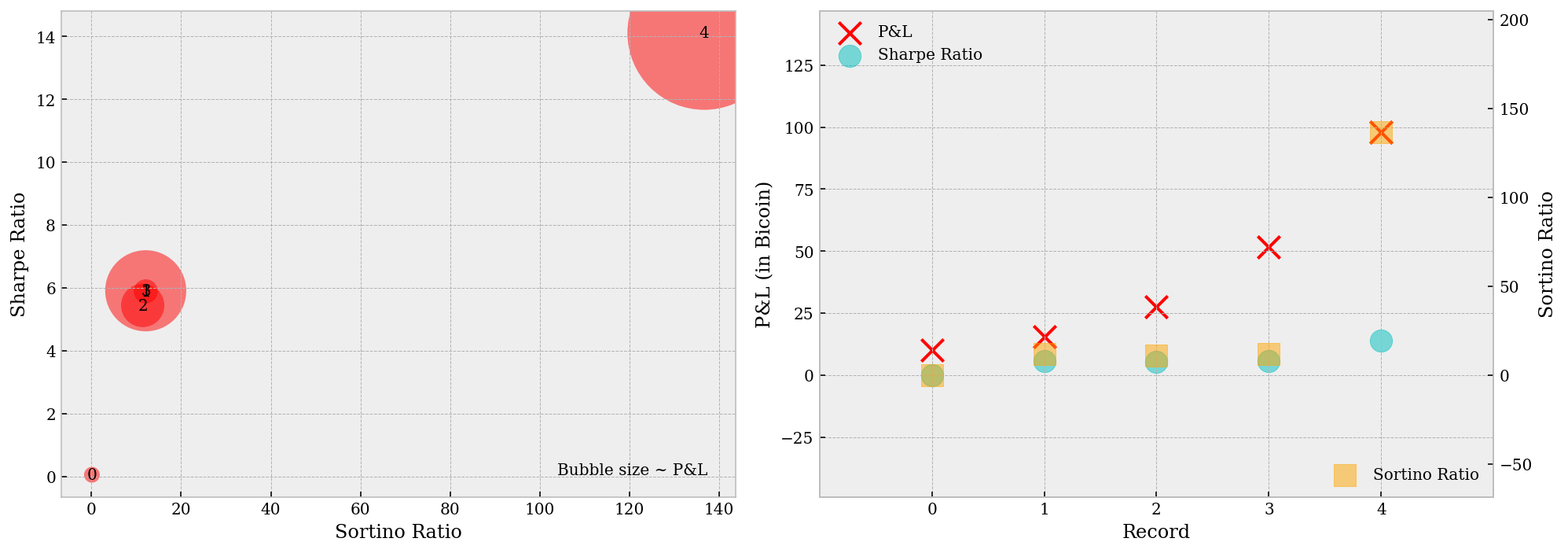# Backtest Result Before a thorough parameter analysis, we can also view the backtest performance in animation (ffmpeg required on your computer). It can be seen that our P&L has a rather similar trajectory comparing with the Bitcoin price, only that it's direction of movement is opposite to the second. This implies we're probably holding short positions most of the time. # Parameter Analysis In this section, we try to take an overall look on the whole parameter grid as well as the outputs. Here are several questions we intend to answer by the end of this part: ### Is is true that performance is monotonic w.r.t. $$j$$ (and $$k$$)? As we found in the previous section, with larger $$j$$ (and $$k$$) values we have higher P&L and ratios. We will investigate into this issue here. The two plots below gives some insight on this question. As we can tell from the left figure below, the best performance from larger $$j$$ (and $$k$$) values are indeed greater than those from smaller values, however, so are the worst results. This result coincides with the intuitive that larger position range means larger risk exposure over time, and therefore, more uncertainty in performance.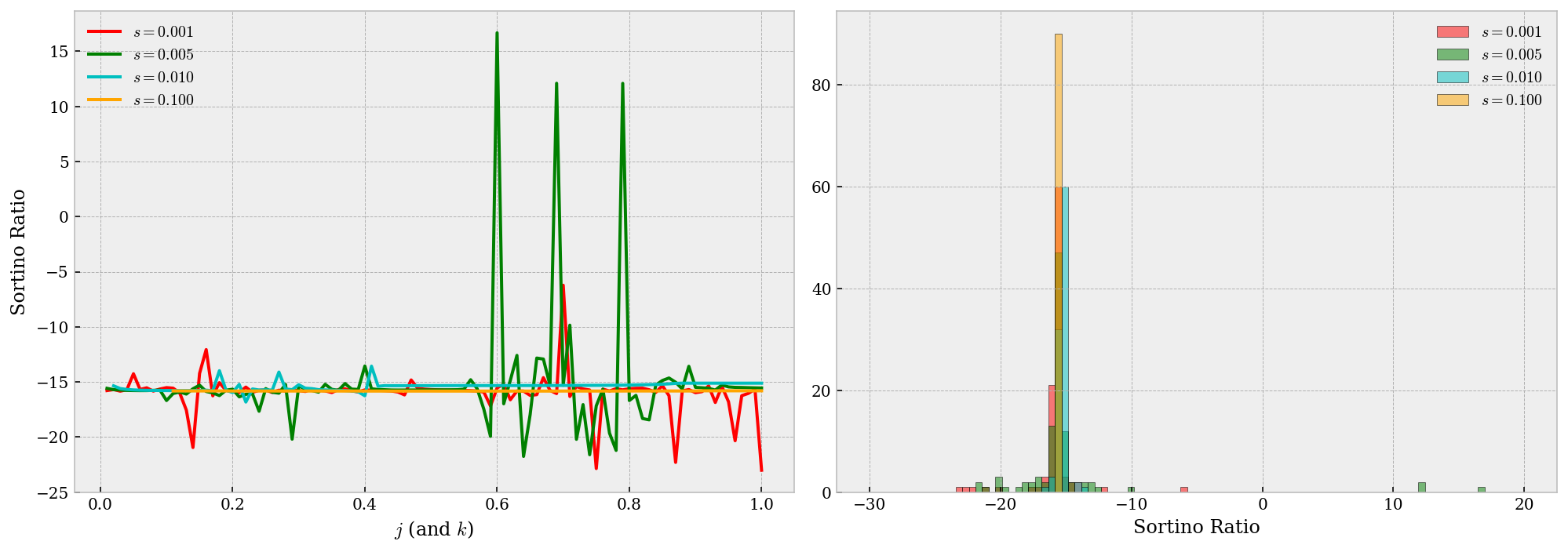### Does smaller $$s$$ yields better performance? Similar as above, this guess is suggested from our grid search. First, from the right figure above we may tell that smaller $$s$$ yields a more volatile performance -- by volatile, it means we have more chance to attain better results. In the contrast, larger values give significantly more robust (yet around a negative Sortino ratio) performance and thus we conclude smaller $$s$$ are more preferable. ### Potential problems in the backtest? There could be a lot of problems in fact, e.g. we are never a "perfect" market maker. But more severely, we may encounter some problem that we could've avoided, e.g. are we significantly biased to one side of trade, or, are we overfitting our model?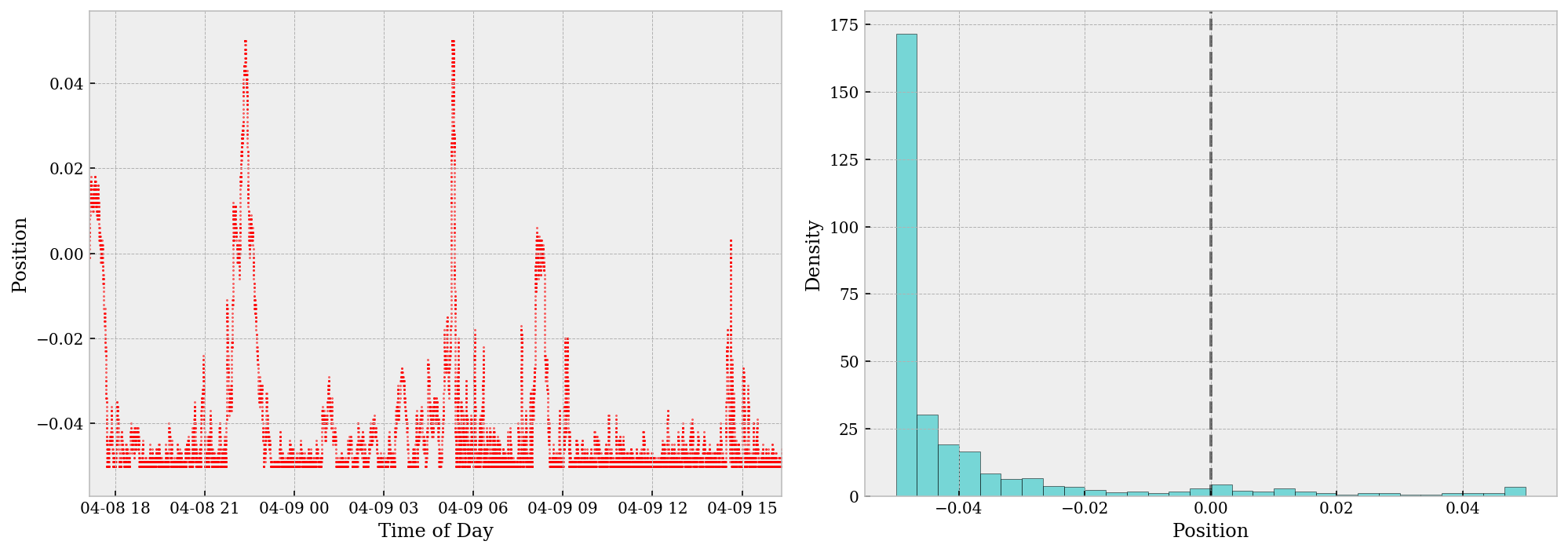The two figures above shows the progress of our position over time. The position is, by and large, negative throughout the day. This can be infered either from the left scatter plot or from the right histogram (which is extremely biased to the left). The positive skewness in our position is a ruthless indication that we've overfit the model, mostly due to limitation of data. On such a small dataset, overfitting is highly risky and likely without cross-validatin methods etc. A potential cure for this may be using a larger dataset, or try to k-fold the timespan for CV. In the meantime, let's take a step back and analyze why the grid search gives us short position most time of the day. As far as I'm concerned, this is mainly because the profit we can obtain from taking a short position most of the time overwhelms that we can achieve from dynamic adjusting our side of trade and maintaining a neutral position. In a particular market like this given one where the general tendency of price is declining, a simple grid search ends up like this and we should've been aware of this before the whole analysis. Numerically, the market making profit in this particular example is the price difference from each matched bid/ask and the corresponding mid price, which we used to calculate position market values. Under this setting, every trade we made we obtain a certain piece of revenue at no cost. The buy-and-hold profit, on the other hand, comes from holding a short position (in our story) and wait for the price to decline. We know the second profit is significantly larger than the first. Theoretically, in order to fix this problem in its essence, we need to add one more parameter into our model, a parameter that rewards neutral positions or punishes holding a outstanding one. Available candidates include time-dollar product of a lasting position and moving averages of positions. # Conclusion In this research, we tried to wrap up a simple backtest engine with a very special "perfect" market making setting. The setting is proved to be unrealistic but still provided a number of insights after detailed analysis. In the meantime, we may improve the model in a variety of ways based on the last sector Parameter Analysis. ]]> <p>In this research report we try to simulate and explore difference scenarios for a "perfect" market maker in the Bitcoin market. By "perfect" we refer to the capability to capture all spreads on the right side of any trade, i.e. there will be no spread loss at all. Although this setting is too perfect to be considered comparable with real trading, our analysis w.r.t. model parameters are believed to be insightful still.</p> Spread Trading: XOP and DRIP http://allenfrostline.com/2019/04/23/spread-trading-etf/ 2019-04-23T05:32:04.000Z 2019-04-23T06:20:00.488Z In this strategy we try to do spread trading based on the M-day (adjusted) returns of two highly related ETFs (exchange-traded funds). The intuition is to hedge the one-sided risks of buy-and-holding one specific ETF with (in expectation) increasing returns, by holding an opposite position of another ETF with decreasing returns. Once we have that the two ETFs' returns are highly correlated, we can trade and make profit by this sort of pair trading. Apart from M, we define trading thresholds g and j, together with stop-loss threshold s. Total capital limit K is assumed to be twice of N, namely the 15-day rolling median volume (of the less liquid ETF). Specifically, we first calculate the array of daily minimums of the two (adjusted) volume series, and then calculate the 15-day rolling median of this series as N. Apart from the capital limit, we also define daily position value (if any) based on N, which is N/100. Specifically, for each trading day, we have workflow as below.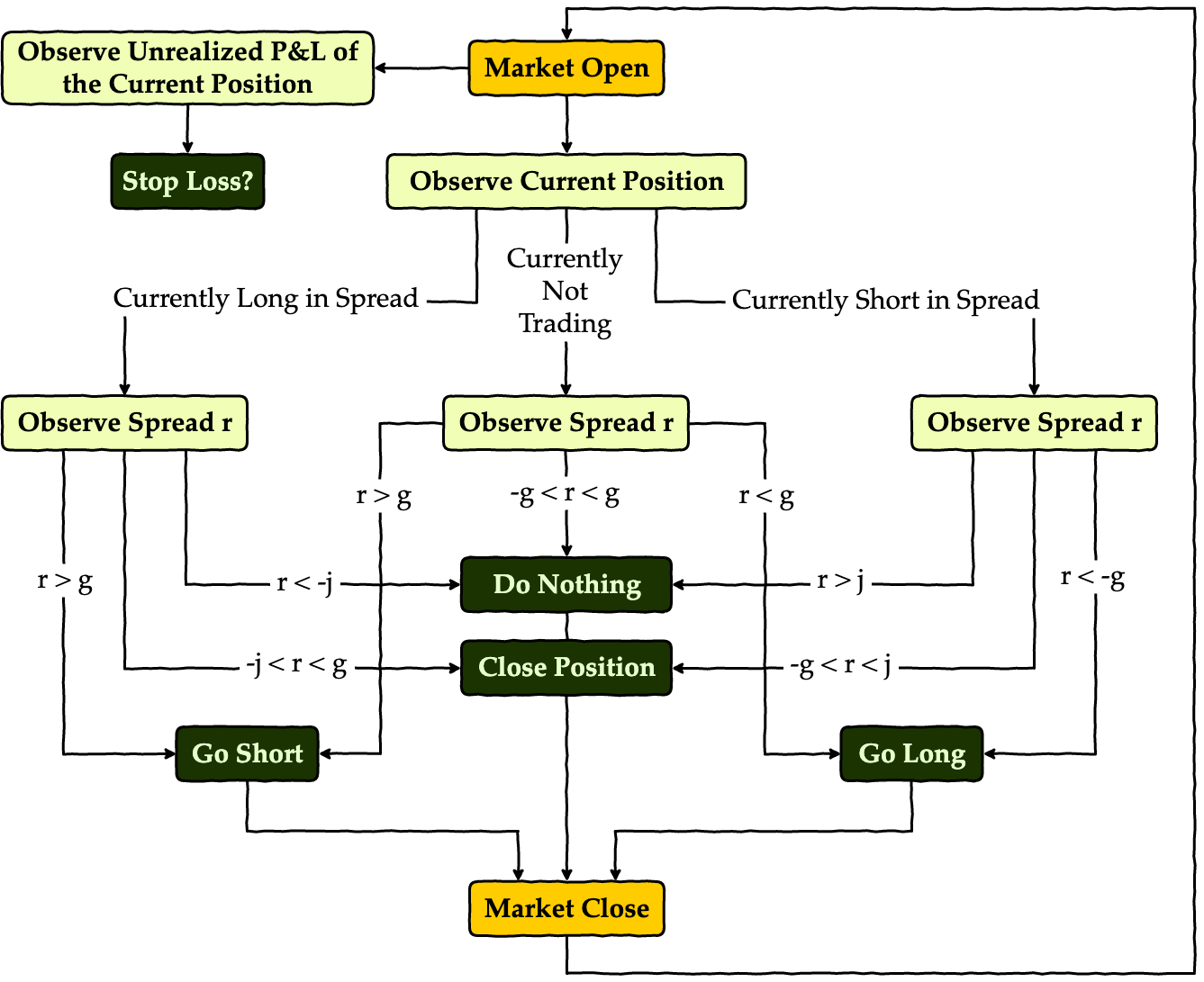Apart from this process, we also keep track of our risk exposure with a stop-loss threshold, and try to do trading only within a month's time, i.e. we start trading only when it's the start of a new month, and kill any position every time it's the end of a month. # Dependencies Import necessary modules and set up corresponding configurations. In this research notebook, we are using the following packages: • Quandl: source of financial data • NumPy: mathematical tools & matrix processing • Pandas: data frame support • Matplotlib: plotting • SciPy: statistical analysis • StatsModels: statistical analysis # Preparatory Analysis In this part, we will try to analyze the economic and statistical features in the data. Here the two ETFs we're using are XOP and DRIP. Data is retrieved from Quandl from 2015-12-02 to 2018-12-31. We'll use only data from 2015-12-02 to 2016-12-01 for this section, as we don't want to include future information during the backtest. Also, while it's always better to have longer historical data for analysis, due to limited length of ETF data on Quandl (specifically for these two ETFs) we're unfortunately restrained to this short timespan. ### Definition of XOP The SPDR S&P Oil & Gas Exploration & Production ETF (XOP) seeks to provide investment results that, before fees and expenses, correspond generally to the total return performance of the S&P Oil & Gas Exploration & Production Select Industry Index. See here for more detailed description. ### Definition of DRIP The Direxion Daily S&P Oil & Gas Exp. & Prod. Bull and Bear 3X Shares (DRIP) seek daily investment results, before fees and expenses, of 300% of the inverse, of the performance of the S&P Oil & Gas Exploration & Production Select Industry Index. See here for more detailed description. ### Relationship of XOP and DRIP By definition of the two ETFs, we expect DRIP to track -300% the daily return of XOP. This means the spread we should be tracking is, instead of the return difference between the two, the difference of -300% of M-day returns of XOP and the M-day returns of DRIP. Also, we are supposed to hold, if any, positions of these two ETFs in a ratio of XOP:DRIP = -3, no matter we're long or short in the spread. A peek into the data (assume M is 5.): • DRIP: price of DRIP • XOP: price of XOP • rDRIP: 5-day return of DRIP • rXOP: 5-day return of XOP • rXOPn3: -300% of 5-day return of XOP • spread: spread of rXOPn3 from rDRIP ( spread = rDRIP - rXOPn3 ) The first few entries of our data reads: DateDRIPXOPrDRIPrXOPrXOPn3spread 2015-12-0993.22840631.4818580.300551-0.0918740.2756210.024930 2015-12-1087.78360032.0336610.175401-0.0634020.190207-0.014806 2015-12-11100.66099730.4653770.247456-0.0849080.254725-0.007269 2015-12-14107.98247129.7199580.113006-0.0415240.124571-0.011565 2015-12-15101.68575730.3104850.065597-0.0285450.085635-0.020037 2015-12-16108.53806329.6328310.164217-0.0587330.176199-0.011983 2015-12-17118.71157728.6163500.352321-0.1066790.3200360.032284 2015-12-18125.55166128.1547310.247272-0.0758450.2275350.019737 2015-12-21130.26789927.8628710.206380-0.0624860.1874590.018921 2015-12-22125.00829128.2033740.229359-0.0695180.2085530.020806 Also we may plot the histogram of the spread. Here we plot it against the fitted normal and t distributions. Apparently the t distribution matches our spread data better, which coincides with our expectation as financial data is commonly seen with fat tails. Also, we may notice that the spread is well centered around zero, which reassures us that we can assume symmetrical thresholds for trading.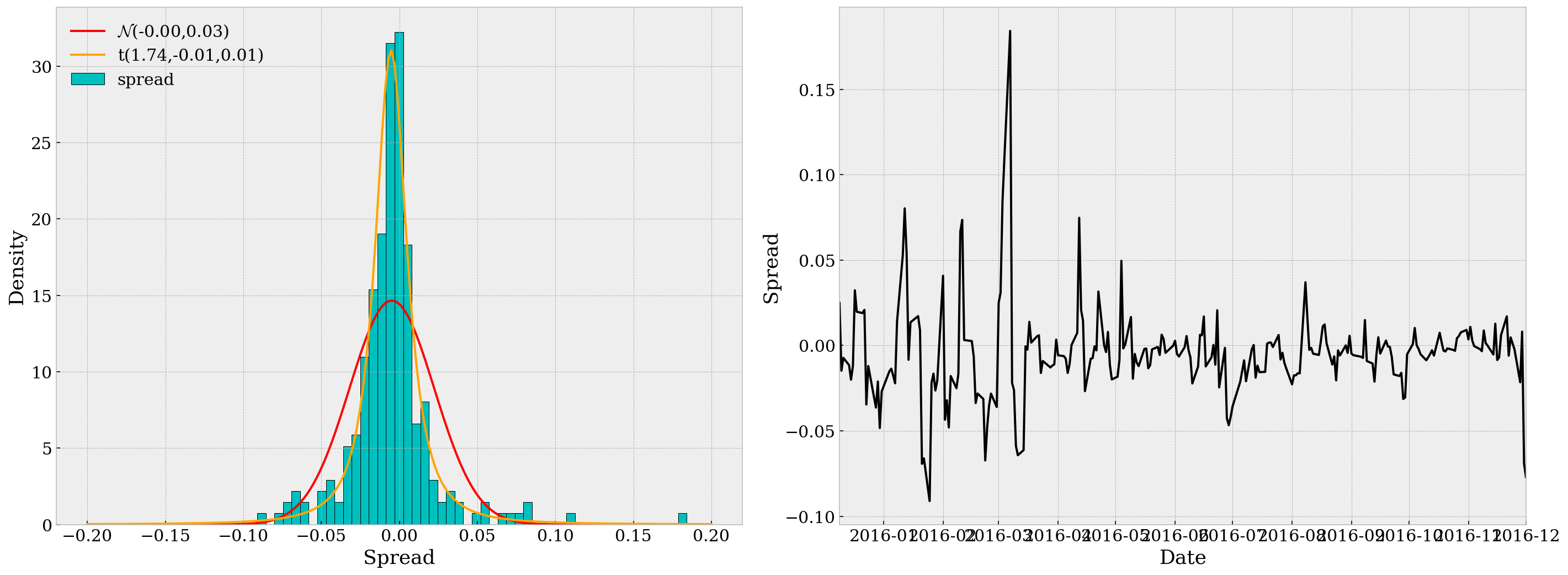In the second subplot, we can see that the spread series is quite "stationary" over time, but we'd better not stop just observing by eye. (Also it's a bit heteroskedastic, but we're not focusing on that in this research.) ### Statistical Tests Below are some statistical tests we need to run through before actual pair traing. For detailed reasoning please refer to this post. • Test for Unit Root: Here we use the Augmented Dickey-Fuller test. The p-value is 6.35e-14 $$\ll$$ 0.05, so we may safely reject the null. ADF Statistic: -8.614239430241229p-value: 6.353844261802846e-14Critical Values: 1%: -3.458 5%: -2.874 10%: -2.573 • Test for Strong Stationarity: Here we opt for the Hurst Exponent. By definition, Hurst value H < 0.5 indicates the time series is mean-reverting, and our H value for the spread is -0.0390, so assumption is confirmed. H: -0.0390 Based on the previous two test results we conclude our spread is mean-reverting and the strategy is reasonable. # Backtest Engine In this part we design a simple backtest engine that takes ETF symbols, backtest timespan and the theoretical return ratio. It then provides an interface to run backtest against different parameters. I've encapsulated private methods/variables in the class BacktestEngine and there are only three attributes available: • BacktestEngine.symbols: tuple of ETF symbols • BacktestEngine.run: run backtest (returns Sortino ratio, Sharpe ratio, maximum drawdown and YoY return) • BacktestEngine.df: stores the data from backtest (trade log) The basic usage of this engine would be and if you want to check the tradelog during the timespan, call be.df. Note in this data frame, we denote the two ETFs by X and Y, and instead of the original M-day return of Y, we denote rY as ratio times the original M-day returns. The positions of X and Y are also reported in be.df together with daily and cumulative returns (in percentages of K). An example of this be.df would be DateXYrXrYspreadNpXpYdaily_rtncum_rtn 2016-12-2212.26748041.323160-0.019732-0.017567-0.0021651.549400e+07000.00.0 2016-12-2312.20821741.450761-0.017488-0.015711-0.0017781.210184e+07000.00.0 2016-12-2712.00079641.666701-0.018578-0.016343-0.0022351.064284e+07000.00.0 2016-12-2812.44527041.1661120.0031850.004286-0.0011019.867562e+06000.00.0 2016-12-2912.69220040.9010940.0174190.0171800.0002399.607867e+06000.00.0 Here for illustration, we make a test run with parameters M=5, g=0.010, j=0.005 and s=0.01. The timespan, as required throughout the analysis, is set from 2016-12-02 to 2018-12-31 inclusive. The special meta parameter ratio is set to -3.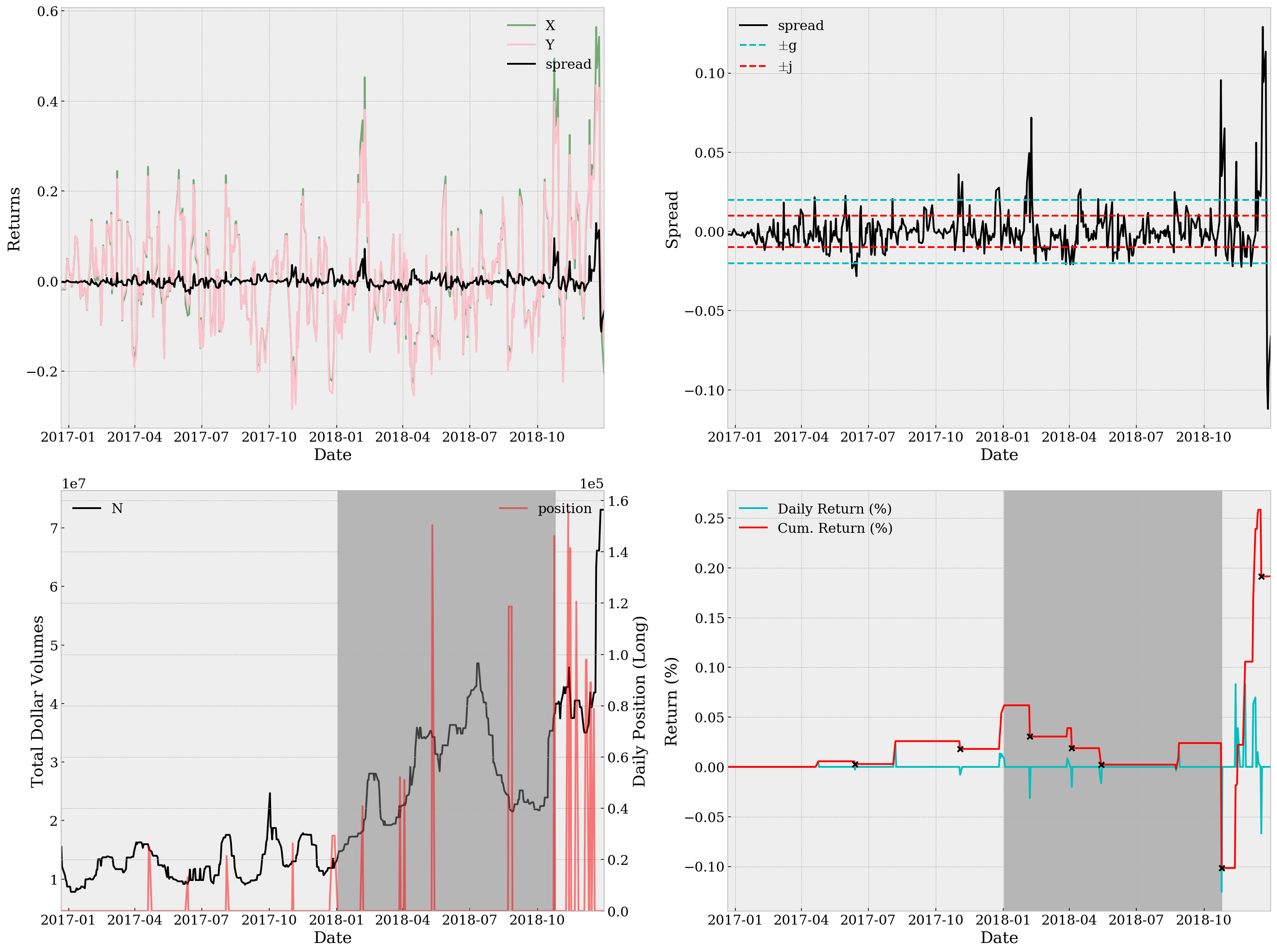Sortino Ratio=0.0574, Sharpe Ratio=0.0387, Maximum Drawdown=1.118e-11, YoY Return=0.09% With only a Sortino ratio of 0.0574, a Sharpe ratio of 0.0387 and YoY return of 0.09%, it's definitely not a good strategy. Not to mention the unsatisfactory return plots. The top right subplot together with the bottom left one suggests that we might be using too wide thresholds. In case of detailed analysis, we can also take a look at be.df and specifically the trading days when we have non-zero positions, which turns out rather few (and supports our worry about wideness of thresholds): DateXYrXrYspreadNpXpYdaily_rtncum_rtn 2017-04-2019.07287034.4139810.1919750.1789840.0129911.533244e+07-25014-46430.0000000.000000 2017-04-2118.84569434.5320050.0971820.100743-0.0035611.496846e+07-25014-46430.0000110.000011 2017-06-1221.52241532.279704-0.076695-0.055866-0.0208299.166219e+061312229840.0000000.000056 2017-08-0422.74718830.8275850.1429280.143423-0.0004951.754893e+07-21483-58270.0000000.000029 2017-11-0215.31953534.463445-0.210285-0.2276370.0173521.302198e+07-26349-37340.0000000.000259 2017-12-2611.39828737.260895-0.221848-0.2494960.0276491.189655e+07-29352-32640.0000000.000180 2017-12-2711.64521736.963916-0.200136-0.2180410.0179051.351392e+07-29352-32640.0001350.000315 2017-12-2811.41804137.241096-0.152493-0.1631170.0106241.189655e+07-29352-32640.0000920.000406 2017-12-2911.71435736.805528-0.054226-0.042553-0.0116731.246303e+07-29352-32640.0001310.000537 2018-02-0514.33181534.0238300.3573430.3078330.0495101.823786e+07-40842-50610.0000000.000619 2018-03-2813.19379533.9788940.0979420.103973-0.0060312.054748e+075222765790.0000000.000305 2018-04-0312.63004234.3260230.0450080.062801-0.0177922.304128e+075109367030.0000000.000390 2018-05-117.14087040.931377-0.120585-0.1257260.0051413.427444e+07-150390-84640.0000000.000188 2018-08-236.23751241.241724-0.144022-0.1550540.0110332.413513e+07-118650-58980.0000000.000023 2018-08-246.03949541.778234-0.157459-0.1775820.0201232.205453e+07-118650-5898-0.000041-0.000018 2018-08-275.96028941.877588-0.146099-0.1525800.0064812.155575e+07-118650-58980.0000980.000081 2018-10-249.09636835.5002950.4942900.3987850.0955053.827526e+07-146172-99130.0000000.000239 2018-11-129.10629934.9530650.1681530.1669350.0012174.281623e+07156027118250.000000-0.001016 2018-11-149.80143634.1073460.3248320.2808040.0440284.281623e+07-141351-134730.000000-0.000185 2018-11-159.36449334.6147780.1361450.1334800.0026654.050823e+07-141351-134730.000016-0.000169 2018-11-2311.21157232.3363110.1972430.197471-0.0002284.050823e+07120567120810.0000000.000222 2018-12-0611.79747331.5204410.1113190.125227-0.0139083.503895e+0797920107630.0000000.001057 2018-12-0711.90670931.3811470.1473680.155996-0.0086283.503895e+0797920107630.0006350.001692 2018-12-1212.98913730.4757300.2099910.1916260.0183654.187946e+07-89073-129880.0000000.002391 2018-12-1313.18774830.2866870.1178450.1174240.0004213.936253e+07-89073-129880.0001480.002539 2018-12-1716.37544928.0778680.2482970.2260810.0222154.187946e+07-78804-136000.0000000.002583 # Parameter Tuning As mentioned above, in this section we try to fit the best set of parameters from 2015-12-02 to 2016-12-01, i.e. the training set. As the focus of this report is not about efficient optimization, we opt for a simple grid search here. The parameter grids are defined as • M_grid: 5, 10, 15, 20 (4 in total) • g_grid: 0.001, 0.003, ..., 0.011 (6 in total) • j_grid: -0.010, -0.008, ..., 0.010 (11 in total) • s_grid: 1e-3, 5e-3, 1e-2, 5e-2, 1e-1 (5 in total) So no more than 1320 simulations are run. Note here parameter combinations where -g < j < g does not hold are neglected. Below are a selection of outstanding parameter sets during simulation. (Record 0) M=15, g=0.007, j=-0.004, s=0.05000, st=1.4542 sr=0.3393, md=1.934e-10, rt= 8.99%(Record 1) M=15, g=0.011, j=-0.010, s=0.05000, st=1.4591 sr=0.3430, md=1.673e-10, rt= 9.17%(Record 2) M=20, g=0.007, j=-0.006, s=0.05000, st=1.5146 sr=0.3540, md=2.076e-10, rt= 9.47%(Record 3) M=20, g=0.007, j= 0.006, s=0.05000, st=1.6001 sr=0.3534, md= 1.7e-10, rt= 9.33%(Record 4) M=15, g=0.011, j=-0.004, s=0.05000, st=1.4680 sr=0.3452, md=1.673e-10, rt= 9.15% From the two plots below, we can tell that Record 3, or the parameter set M=20, g=0.007, j=0.006, s=0.05000, is a good choice as it has both large Sortino ratio/YoY return and a relatively small maximum drawdown. Record 2, or M=20, g=0.007, j=-0.006, s=0.05000 is also playing well among all outstanding parameter sets, with slightly better Sortino ratio and YoY returns but larger maximum drawdown. We'll test on both sets.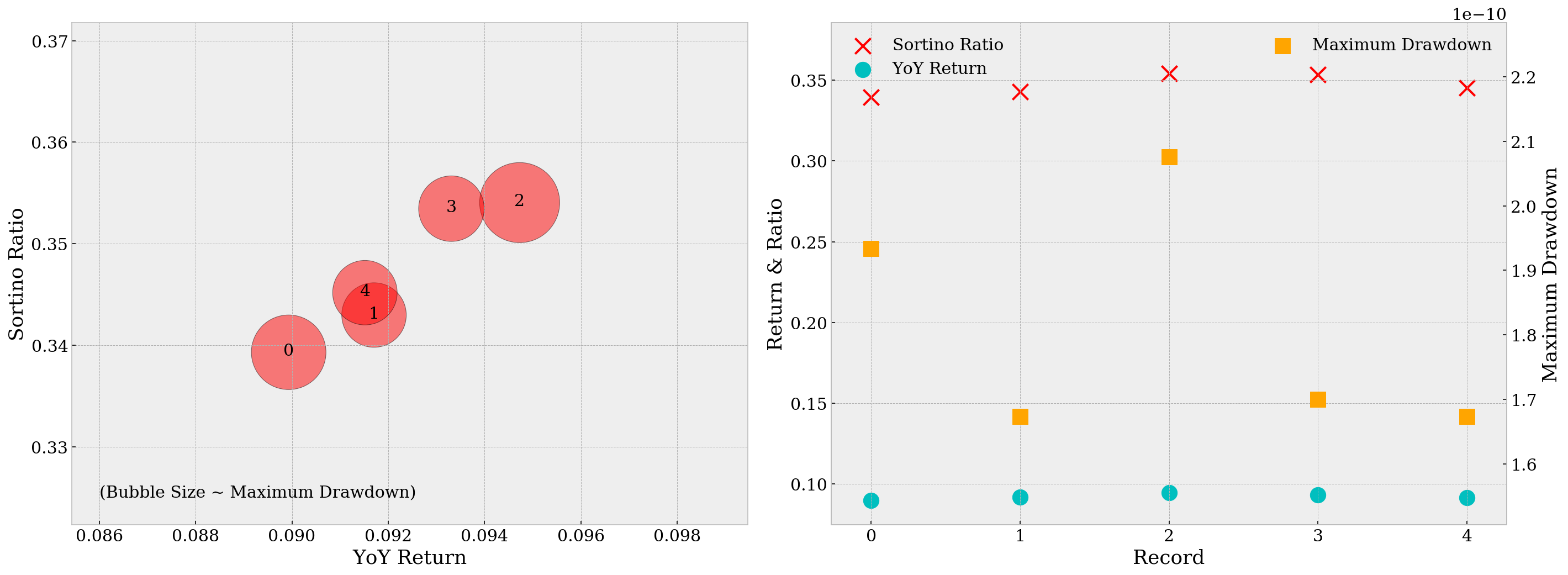Using the parameters from Record 3, we run backtest against the test set, i.e. from 2016-12-02 to 2018-12-31. The plots are as below.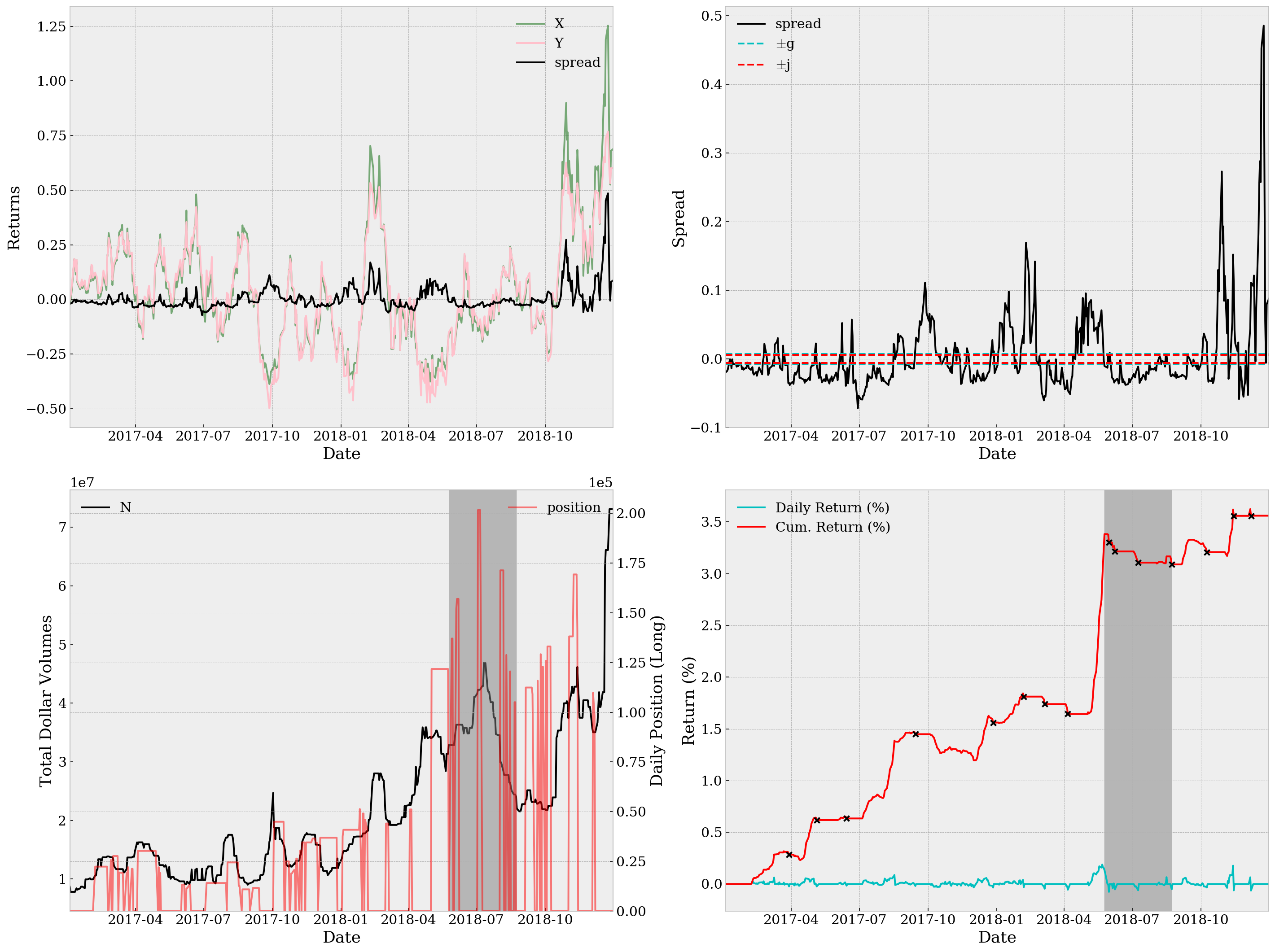Sortino Ratio=0.7407, Sharpe Ratio=0.2447, Maximum Drawdown=2.005e-11, YoY Return=1.76% Using the parameters from Record 3, the backtest result is as below.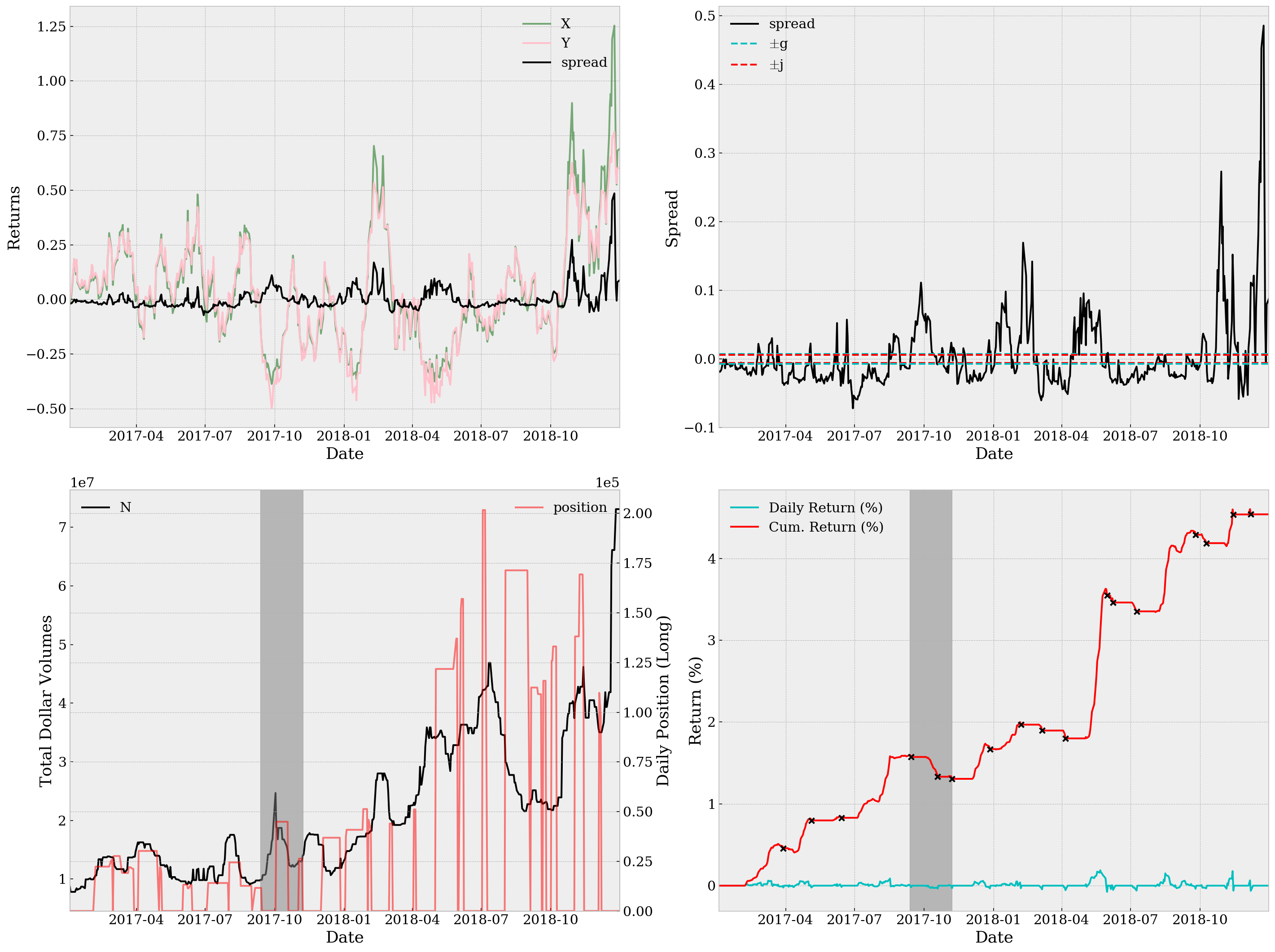Sortino Ratio=0.9394, Sharpe Ratio=0.2872, Maximum Drawdown=1.997e-11, YoY Return=2.24% # Conclusion Both results are amazingly great (especially compared with our result using random parameters before any tuning). Considering here we're not utilizing any future data in backtest, the performance is satisfactory despite we're neglecting a lot executional details in our analysis, like transaction costs and market impacts. There are also several comments on the processing of data: • We are using the first M days to calculate the rolling median of N, which causes a loss in data. Perhaps we should use M days of further previous historical data to make up this loss. • Thresholds change, over time. To be more specific, the "ideal" thresholds change all the time, partly due to market regime shifting and partly other unknown reasons. We may, therefore, never have the "best" parameters for trading. Should we go for a dynamic version of spread trading? I doubt so. But it's worth thinking if there's any remedy for this problem. ]]> <p>In this strategy we try to do spread trading based on the <code>M</code>-day (adjusted) returns of two highly related ETFs (exchange-traded funds). The intuition is to hedge the one-sided risks of buy-and-holding one specific ETF with (in expectation) increasing returns, by holding an opposite position of another ETF with decreasing returns. Once we have that the two ETFs' returns are highly correlated, we can trade and make profit by this sort of pair trading.</p> Bad Interpreter Error of Jupyter and its Solution http://allenfrostline.com/2019/04/17/bad-interpreter-error-jupyter/ 2019-04-17T19:56:13.000Z 2019-04-17T20:04:27.850Z After messing up with my Python virtualenv my computer finally started going nuts. Jupyter notebook threw me the following error every time I start it: It turns out that the cause was that along with reinstallation of Python, the homebrew symlinks to Jupyter are now broken. A simple solution would be Then the notebook starts just fine. ]]> <p>After messing up with my Python <code>virtualenv</code> my computer finally started going nuts. Jupyter notebook threw me the following error every time I start it:</p> <figure class="highlight bash"><table><tr><td class="gutter"><pre><span class="line">1</span><br></pre></td><td class="code"><pre><span class="line">zsh: /usr/<span class="built_in">local</span>/bin/jupyter: bad interpreter: /usr/<span class="built_in">local</span>/opt/python/bin/python3.7: no such file or directory</span><br></pre></td></tr></table></figure> Texas Hold 'em Series: Poker Game (w/ GUI) http://allenfrostline.com/2019/04/16/texas-holdem-series-5/ 2019-04-17T04:10:00.000Z 2019-04-17T16:31:33.231ZI wrote a poker game. This is a simple Texas Hold 'em game running on Mac OS. All scripts are written in pure Python. The main GUI is written using the Python module PySimpleGUI, and hand evaluation is done by refering to a hand value table pre-calculated together with Monte Carlo simulation. See here for detailed explanation of hand evaluation. Together with the GUI version, I also include here a primitive commmandline version with ColorPrint support, which you may download and include from this repo. The two versions are supposed to work identically. ### Usage You don't need any Python or module dependencies installed on your Mac in order to just play the game. The app itself is standalone with everything packed inside it already. There're just two steps: 1. Download the app from here. 2. Double click it.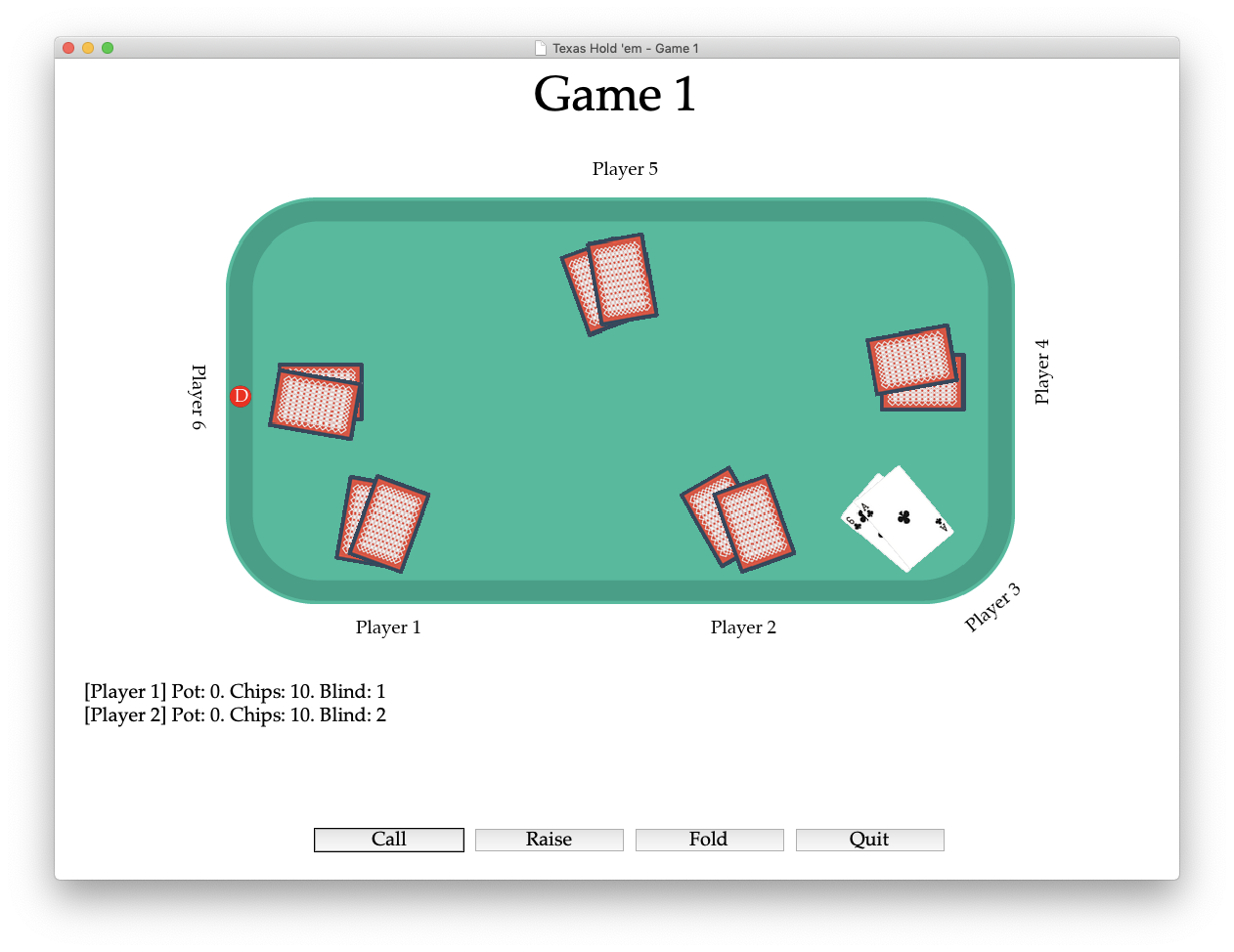Or rather, if you'd like to pack it yourself: 1. Download this repo. 2. Pack it using PyInstaller in --onefile mode. 3. Double click the app you just generated. ### To-Do's There're several things to work on in plan: • Fix bugs (please, don't again). • Write up AI agents of different strategies (in progress): • Expected hand value optimization (Greedy); • Game theory optimal (GTO); • Evolutionary algorithm (EA); • Deep Q-Learning (DQN). • Build a server-based version so multiple people can play on different divices. • Build an Android version (proposed by author of PySimpleGUI, MikeTheWatchGuy). • Fix GUI problems: • Resolution of image is extremely low and it looks ugly on Retina screens. • Button styles and other design-related issues. ### Known Bugs Here are some bugs I'm trying to fix: • Side pot. It's tricky and I havn't found a way to implement it simple. • Freeze at start. The app starts and then freezes for a sec. This is because Python is openning the hand value table. Perhaps I should try threading for this particular task (no need for the rest of the game). I'll also try to compress the table in a better way (like functionize it). Working on the animated Popup element in PySimpleGUI. ### Acknowledgement I appreciate suggestions and encourage from anyone throughout the development (which may still continue for a long time, considering the considerable time I spent just on writing this primitive game). Special thanks to my friends who ever tried to play the game and found bugs starting from the commmandline version. Also, credit for MikeTheWatchGuy who wrote the PySimpleGUI module and helped me fix several bugs. Also, credit to Freepik from www.flaticon.com, who made this fantastic icon. Finally, I wanna give credit to myself for the nights I stayed up after lectures. There is nothing more fulfilling than realizing an impulse right away. ]]> <p><img src="/images/holdem-icon.png" width="80%"></p> <p>I wrote a poker game.</p> Texas Hold 'em Series: Hand Evaluation http://allenfrostline.com/2019/04/09/texas-holdem-series-4/ 2019-04-09T23:12:00.000Z 2019-04-12T09:33:52.720Z In the previous post, we considered the probabilities of making one specific hand with the turn/river card. This can be rather useful in specific situations, but still cannot apply thoughout a game. Poker is essentially an incomplete information game. Different from Go, where you can see all stones placed on the chessboard and thereby "solve" an optimal move, you never know you opponents' pocket cards until showdown (yet even then, people mucks). Also, you have little clue on the unshown community cards. Therefore, in order to evaluate a hand during a poker game, we'd better opt for a online evaluation algorithm instead of considering this as a DP-like problem. # Hand Values For the sake of convenience, the table of hand values is shown again below. NameDescriptionExample High CardSimple value of the card. Lowest: deuce; highest: ace.As 4s 7h Td 2c PairTwo cards with the same value.As 4s 7h Td Ac Two PairsTwo pairs where each pair of cards have the same value.As 4s 4h Td Ac Three of a KindThree cards with the same value.As 4s 4h 4d 2c StraightFive cards in consecutive values (ace can precede deuce or follow up king).9s Ts Jh Qd Kc FlushFive cards of the same suit.Ah 4h 7h Th 2h Full HouseThree of a kind with the rest two making a pair.As 4s 4h 4d Ac Four of a KindFour cards of the same value.As 4s 4h 4d 4c Straight FlushStraight of the same suit.9h Th Jh Qh Kh Royal FlushStraight flush from ten to ace.Th Jh Qh Kh Ah # Evaluation Our ultimate goal is to be able to evaluate the probability of a win (and tie), i.e. the relative strength of our hand. However, let's just try to get one step back and consider evaluating the absolute strengths. Here we denote it as the hand value. Ideally, there are $$\binom{52}{5}=2,598,960$$ possible hands but much less valid values. An intuitive idea to match all these hands to their values, which is also what I did, is to first generate a sparse mapping from hands to values, and then condense it. First we need a function that identifies hand types. Then, for hands within the same type, we encode them like a carry system (e.g. decimal); for hands across different types, we manually add offsets so that higher hand types always yield higher values. The final results are stored in the dictionary hv and serialized locally. The highest value is 6144 and here is a glance of hv: # Simulation Now that we have the full mapping from hands to values, there are two things we can do to calculate the probabilities: 1. Enumerate all opponent hands exhaustively, and compare hand values 2. Simulate certain number of opponent hands, and then compare Things are gonna be much easier when we only consider the two-player case (a.k.a. heads-up games). In that case, we can literally count and evaluate all scenarios — when there're 2 pocket cards for me and 3 community cards shown, we only need to enumerate $$\binom{52-5}{2}=1,081$$ opponent hands, which is lightning fast to finish with modern programming languages like Python or C++. However, when we have more players, like 5, the first method gets nasty. The hands of each opponent are not independent, so we have to go through $$\binom{52-5}{2\times 4} > 3\times 10^8$$ situations and that, different to the heads-up scenario, would be unacceptably slow no matter which language we use. Therefore, we opt for the second method at some cost of precision. The code (partial) is shared below. # Example Below are two example tests. P(win) = 5.4688% P(tie) = 0.4883% P(win) = 1.9531% P(tie) = 0.7812% Note here I also implement an interesting parameter called ranges which represents the opponents prior ranges at pre-flop. When passing an empty value, all combinations of two cards are considered. When we specify a list of ranges (numbers from 0 to 1, say $$x\%$$), then the opponents are assumed to only play when their pocket cards are at least in the top $$x\%$$ pairs of all pairs. See this table for more reasoning. ]]> <p>In the previous post, we considered the probabilities of making one specific hand with the turn/river card. This can be rather useful in specific situations, but still cannot apply thoughout a game. Poker is essentially an incomplete information game. Different from Go, where you can see all stones placed on the chessboard and thereby "solve" an optimal move, you never know you opponents' pocket cards until showdown (yet even then, people mucks). Also, you have little clue on the unshown community cards. Therefore, in order to evaluate a hand during a poker game, we'd better opt for a online evaluation algorithm instead of considering this as a DP-like problem.</p> Texas Hold 'em Series: Odds Chart http://allenfrostline.com/2019/04/05/texas-holdem-series-3/ 2019-04-05T23:35:00.000Z 2019-04-09T23:11:21.944Z In this post we're gonna introduce one of the most widely-used results in hold 'em: the odds chart. # Odds Chart Before showing the odds chart, we first give the mathematical definition of odds. Here we're not focusing on winning a hand, but instead our intended issue is whether we can make an expected hand with the forthcoming unshown card(s). We call the probability of doing that as the improving probability, and define its corresponding odds as $\text{odds} = \frac{1}{\text{improve}\%} - 1$ which means we can bet every $$\1$$ against any pot larger or equal to this amount. Now we try to calculate these probabilities and odds. We here only consider one card to expect and one/two community cards to unveil, namely odds on the river or turn. For example, when we're expecting any of 8 cards on the turn to make a straight, then the improving probability in this case would be $\text{improve}\% = 1 - \frac{45 + 2 - 8}{45 + 2}\times \frac{45 + 1 - 8}{45 + 1} = \frac{340}{1081} \approx 31.45\%$ which means we have $$31.45\%$$ chance to make it and the odds, therefore, is $$1/31.45\% - 1 = 2.2$$, which means we can bet at most $$\1$$ against each $$\2.2$$ pot. More generally, let $$\#\text{n.s.}$$ denote the number of community cards not shown yet, then we have $\text{improve}\% = 1 - \prod_{i=1}^{\#\text{n.s.}} \frac{45 + i - \text{outs}}{45 + i}.$ Below is the table of improving probabilities and corresponding odds w.r.t. different outs. OutsImprove% (River)Odds (River)Improve% (Turn)Odds (Turn) 12.17%454.26%22 24.35%228.42%11 36.52%1412.49%7 48.70%1116.47%5.1 510.87%8.220.35%3.9 613.04%6.724.14%3.1 715.22%5.627.84%2.6 817.39%4.831.45%2.2 919.57%4.134.97%1.9 1021.74%3.638.39%1.6 1123.91%3.241.72%1.4 1226.09%2.844.96%1.2 1328.26%2.548.10%1.1 1430.43%2.351.16%0.95 1532.61%2.154.12%0.85 1634.78%1.956.98%0.75 1736.96%1.759.76%0.67 # The 2/4 Times Rule Multiplying the number of outs by two or four gives a reasonable approximation to the improve% (river) or improve% (turn) respectively, in the above table. This is a famous (yet quite rough) approximation among hold 'em gamers. The rule is a direct corollary from the abovementioned formula, as when $$\#\text{n.s.}=1$$, $\text{improve}\% = 1 - \frac{46 - \text{outs}}{46} = \frac{\text{outs}}{46} \approx (2\text{outs}) \%$ and when $$\#\text{n.s.}=2$$, \begin{align*}\text{improve}\% &= 1 - \frac{46 - \text{outs}}{46}\times \frac{47-\text{outs}}{47} \\&= \frac{93}{2162}\text{outs} - \frac{1}{2162}\text{outs}^2 \approx (4\text{outs}) \%.\end{align*} ]]> <p>In this post we're gonna introduce one of the most widely-used results in hold 'em: the odds chart.</p> Texas Hold 'em Series: Poker Hands Dataset http://allenfrostline.com/2019/03/30/texas-holdem-series-2/ 2019-03-30T19:25:00.000Z 2019-03-30T20:46:00.822Z In this post, I'll walk through the whole process to download, clean and then browse one of world's largest poker hands history dataset, the IRC Poker Database, which is a little bit aged but well-known for its huge size. The work we're doing here is meant to be a preparation for further analysis and model training. # History Before the advent of real-money online poker servers, there was the Internet Relay Chat (IRC) poker server. The server was programmed by Todd Mummert, with support code by Greg Reynolds, and other Usenet rec.gambling.poke enthusiasts. The participants in these games were mostly computer geeks with a passion for poker. Many were serious students of the game, armed with the analytical skills needed to understand the mathematics, and all other aspects of advanced poker strategy. Michael Maurer wrote a program called Observer that sat in on IRC poker channels and quietly logged the details of every game it witnessed. This resulted in the collection of the more than 10 million complete hands of poker (from 1995-2001) that constitute the IRC Poker Database. Sadly, the IRC games are now gone (but might be resurrected one day). # Notation We'll be using the same short-hand notations as we gave in the last post. For bet actions, we define • -: no action • B: blind bet • f: fold • k: check • b: bet • c: call • r: raise • A: all-in • Q: quit • K: kicked out As for rounds, we denote • p: pre-flop • f: flop • t: turn • r: river • s: showdown ## Data Preparation I've written several scripts for all sorts of data preparations and the code can be found on my GitHub repository. After entering the repo, run the following codes in order: Eventually there're $$10{,}233{,}955$$ hands in hands.json and $$437{,}862$$ in hands_valid.json after cleaning. ## Data Inspection You may run the following code to inspect hands in their original order. Any time you'd like to stop browsing, you can just use Ctrl+C to interrupt the process. The script lists extracted hands history as below. ############################################################ time : 199612 id : 2093 board : ['Qd', '6s', 'Td', 'Qc', 'Jh'] pots : [(2, 60), (2, 60), (2, 60), (2, 60)]players : Tiger (#1) {'action': 30, 'bankroll': 2922, 'bets': [{'actions': ['B', 'r'], 'stage': 'p'}, {'actions': ['k'], 'stage': 'f'}, {'actions': ['k'], 'stage': 't'}, {'actions': ['k'], 'stage': 'r'}], 'pocket_cards': ['9s', 'Ac'], 'winnings': 30}· · · · · · · · · · · · · · · · · · · · · · · · · · · · · · jvegas2 (#2) {'action': 30, 'bankroll': 139401, 'bets': [{'actions': ['B', 'c'], 'stage': 'p'}, {'actions': ['k'], 'stage': 'f'}, {'actions': ['k'], 'stage': 't'}, {'actions': ['k'], 'stage': 'r'}], 'pocket_cards': ['9c', 'As'], 'winnings': 30}############################################################ So this screenshot describes hand #2093, which happened in December of 1996. There were two players at the table, namely Tiger (the SB) and jvegas2 (the BB). By default the game started with Tiger paying $$\5$$, who's got a 9♠ and an A♣ with a bankroll of $$\2{,}922$$, and jvegas2 paying $$\10$$, whose pocket cards were 9♣ and A♠ with a bankroll of $$\139{,}401$$. Then Tiger raised to $$\30$$ (3BB) and jvegas2 called. So preflot pot was60 and there're two players. The flop was Q♦, 6♠ and 10♦. It was a dry hand by far so both checked at the flop. The turn was Q♣, and then both checked again. At the river came J♥, nothing special, and again checked both. Both players stuck to the showdown and it was a tie, so the two shared the total pot $$\60$$.

1. 1.Their website is still well maintained. ↩︎
2. 2.I confess that I copied this section from their homepage. ↩︎
3. 3.Special thanks to this script from Miami Data Science Meetup 2015. There're several bugs but essentially I used their extraction functions. ↩︎
]]>
<p>In this post, I'll walk through the whole process to download, clean and then browse one of world's largest poker hands history dataset, the IRC Poker Database<sup id="fnref:1"><a href="#fn:1" rel="footnote"><span class="hint--top-right hint--error hint--large" data-aria-label="Their [website](http://poker.cs.ualberta.ca/irc_poker_database.html) is still well maintained. "></span></a></sup>, which is a little bit aged but well-known for its huge size. The work we're doing here is meant to be a preparation for further analysis and model training.</p>
Texas Hold 'em Series: Basic Concepts http://allenfrostline.com/2019/03/28/texas-holdem-series-1/ 2019-03-28T21:33:00.000Z 2019-03-30T20:58:54.801ZStarting from today, I'm gonna write a series of posts on Texas Hold 'em, one of world's most famous forms of poker. The game is rather complicated, especially considering its origin dating back to early 20th century. In this post, I will list the bare-bones of hold 'em. These concepts may sound boring to you if you are a veteran poker player, but I just want to make sure we're talking in the same language — or building using the same bricks.

# Cards

Texas hold 'em uses all cards but the two jokers from one deck of poker. So there're 13 cards wearing each of the four suits: diamonds (), hearts (), clubs (♣) and spades (♠). The exhaustive array of cards are:

(Suit)AceDeuceThreeFourFiveSixSevenEightNineTenJackQueenKing
A♦2♦3♦4♦5♦6♦7♦8♦9♦10♦J♦Q♦K♦
A♥2♥3♥4♥5♥6♥7♥8♥9♥10♥J♥Q♥K♥
A♣2♣3♣4♣5♣6♣7♣8♣9♣10♣J♣Q♣K♣
A♠2♠3♠4♠5♠6♠7♠8♠9♠10♠J♠Q♠K♠

Besides these full-length notations, I'll also be using abbreviations where I denote d for diamonds, h for hearts, c for clubs and s for spades. Also, I use T to represent cards of 10. Thereby, we can easily use a two-character string, e.g. Ts, to refer to the card 10♠. This short-hand is gonna be especially useful when we try to program the game in the forthcoming posts.

# Table and Positions

There are in general three types of hold 'em games based on the number of players at the table: 2 (called "heads-up"), 3-6 (called "6-max") and 7-10 (this is called "full-ring"). The maximum number of players in a Texas hold 'em game is ten, which means there're ten players and one dealer who does not play. That makes a regular 10-player table full (well, with dealer standing).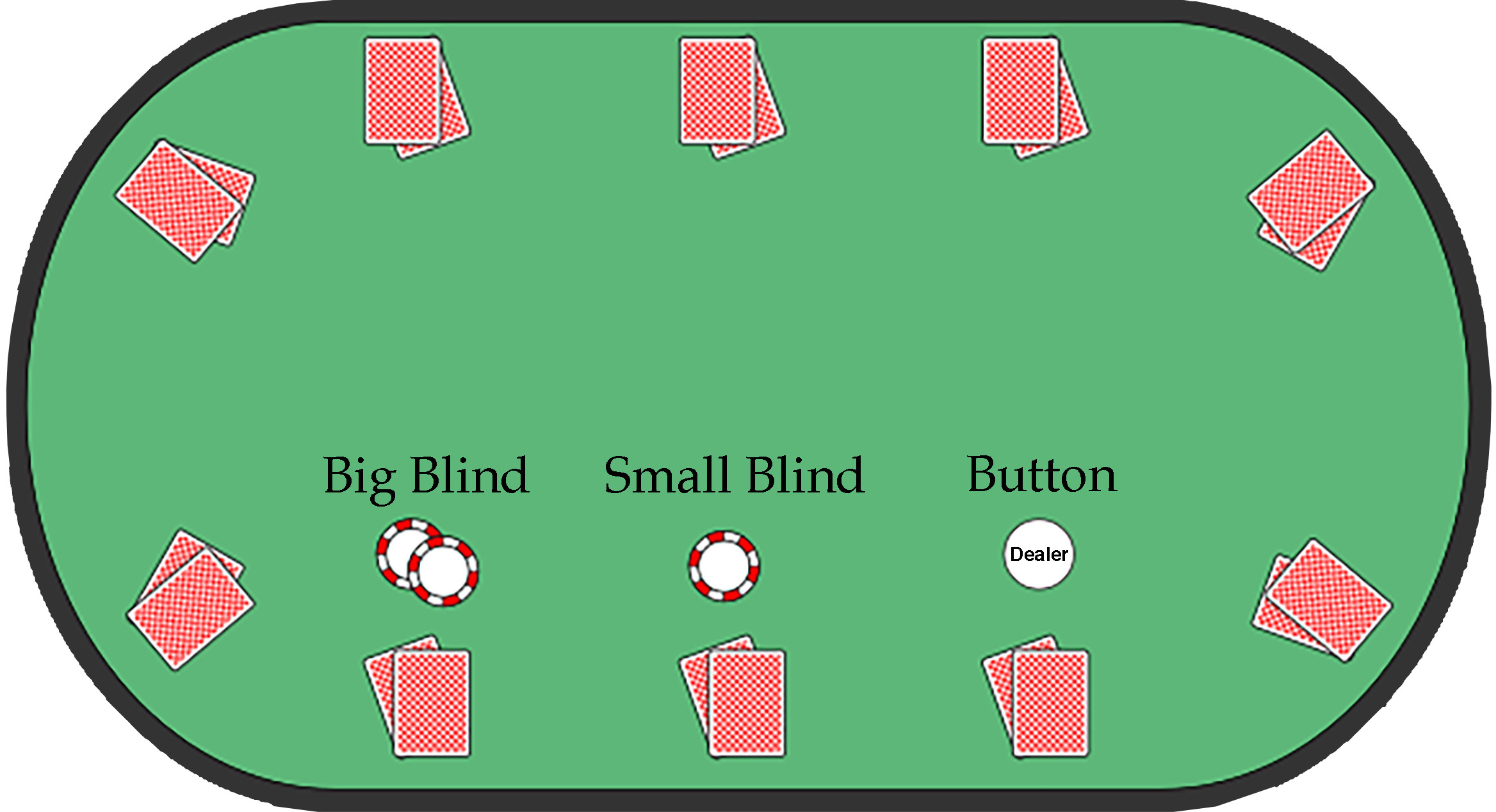There are a variety of positions of the table (ignoring the dealer's), but the most important are three: button (BTN), small blind (SB) and big blind (BB). In most self-dealt games where there is no specific person serving as a dealer, the button also serves as the dealer, which is why you can see the "dealer" chip at the same position. The blinds are forced contributions and are paid before the pocket cards (the two cards for each player at the beginning of the game, see figure above): SB pays first and is asked half the money of BB, then BB pays his forced bet and finally the dealer gives away all the pocket cards in a clockwise manner starting from SB. Therefore, BTN would be the last one to receive his pocket cards and also to act (bet or fold, see "betting" below).

Besides BTN, SB and BB, we also usually call the first player to the left of BB as under-the-gun (UTG), which vividly points out that he's the first to act on this table, as SB and BB are forced to pay their bets.

# Betting

There are four kinds of actions you can take each round:

• Fold: You can discard your pocket cards and forfeit interest in the current pot, i.e. quit the game. This is a valid option throughout the game.
• Bet/Raise: As the name indicates, you always have the right to wager your chips into the pot. It's called a bet when no one else has yet done so before you, and a raise if someone has bet some but you choose to put more.
• Call: To call is to match a bet or raise. A betting round ends when all active players have bet an equal amount or everyone folds to a player's bet or raise. If no opponents call a player's bet or raise, the player wins the pot.
• Check: If no one else has yet opened a betting round, then you're allowed to just pass the round, which is equivalent to betting zero and/or to calling the current bet of zero.

# Play of the Hand

The hand begins with a pre-flop betting round, beginning with UTG and continuing clockwise. A round of betting continues until every player has folded, put in all of their chips, or matched the amount put in by all other active players.

After the pre-flop betting round, assuming there remain at least two players taking part in the hand, the dealer deals a flop: three face-up community cards. The flop is followed by a second betting round. This and all subsequent betting rounds begin with the player to the dealer's left and continue clockwise.

After the flop betting round ends, a single community card (called the turn or fourth street) is dealt, followed by a third betting round. A final single community card (called the river or fifth street) is then dealt, followed by a fourth betting round and the showdown, if necessary. In the third and fourth betting rounds, the stakes double.

To sum up, players have four rounds to make actions: pre-flop (given a pocket of two cards), flop (reveal three community cards), turn (reveal one more community card) and river (reveal the last one community card).

# Hand Values

The following table shows the possible hand values in ascending order.

NameDescriptionExample
High CardSimple value of the card. Lowest: deuce; highest: ace.As 4s 7h Td 2c
PairTwo cards with the same value.As 4s 7h Td Ac
Two PairsTwo pairs where each pair of cards have the same value.As 4s 4h Td Ac
Three of a KindThree cards with the same value.As 4s 4h 4d 2c
StraightFive cards in consecutive values (ace can precede deuce or follow up king).9s Ts Jh Qd Kc
FlushFive cards of the same suit.Ah 4h 7h Th 2h
Full HouseThree of a kind with the rest two making a pair.As 4s 4h 4d Ac
Four of a KindFour cards of the same value.As 4s 4h 4d 4c
Straight FlushStraight of the same suit.9h Th Jh Qh Kh
Royal FlushStraight flush from ten to ace.Th Jh Qh Kh Ah

A player may use any five cards out of the seven available cards, namely the two pocket cards and five community cards, to reach the highest hand value he may attain. The player with the highest hand value wins the pot unless all but one player folds before showdown (showing pocket cards after the last betting round).

]]>
<p><img src="/images/holdem-royalflush.jpg" width="100%"></p> <p>Starting from today, I'm gonna write a series of posts on Texas Hold 'em, one of world's most famous forms of poker. The game is rather complicated, especially considering its origin dating back to early 20th century. In this post, I will list the bare-bones of hold 'em. These concepts may sound boring to you if you are a veteran poker player, but I just want to make sure we're talking in the same language — or building using the same bricks.</p>
Random Projection and Its Expectation http://allenfrostline.com/2019/03/16/random-projection-and-expectation/ 2019-03-16T17:50:50.000Z 2019-03-16T23:26:30.494Z

A couple of months ago I was asked the following question during an interview (for propriatary concerns I'm not gonna disclose the industry or name of the company): $$\newcommand{R}{\mathbb{R}} \newcommand{E}{\text{E}} \newcommand{bs}{\boldsymbol} \newcommand{N}{\mathbb{N}}$$

Assume $$k$$, $$n\in\N$$ and $$k < n$$. For a uniformly chosen subspace $$\R^k\subsetneq\R^n$$ we define the orthogonal projection as $$P:\R^n\mapsto\R^n$$. Find $$\E[P(\bs{v})]$$ where $$\bs{v}\in\R^n$$ is given.

It's an interesting question and also a totally novel one to me at that time. How do we define a "uniformly" chosen subspace and its corresponding projection? What are the possible intuitions in this simple piece of question? Despite the busy schoolwork and student projects, these thoughts persist in my mind and drive me digging this question from time to time. Curiosity has been aroused and an appetite is meant to be satisfied.

# Problem Re-formulation

In order to solve this problem, we need to fully understand what's been asked. So now we've got two non-negative integers $$k<n$$ and two spaces, namely $$\R^n$$ and $$\R^k$$. We know $$\R^k$$ is somehow randomly selected as a subspace of $$\R^n$$ and this randomness is uniform. For each of such selection, we can make an orthogonal projection of the given point $$\bs{v}$$ onto $$\R^k$$. Note here the projection is defined from $$\R^n$$ to $$\R^n$$, which means we're not interested in the projected value on $$\R^k$$ but the projection itself. In other words, we're focusing on the projected vector's behavior in the same space as $$\bs{v}$$ here.

# Simple Example

The simplest example (well, it's in fact not THE simplest as we could always project $$\bs{v}$$ onto $$\R^0$$ and the resulting expectation would be a zero vector) would be $$n=2$$ and $$k=1$$. For any given $$\bs{v}$$, we can always draw a graph as below.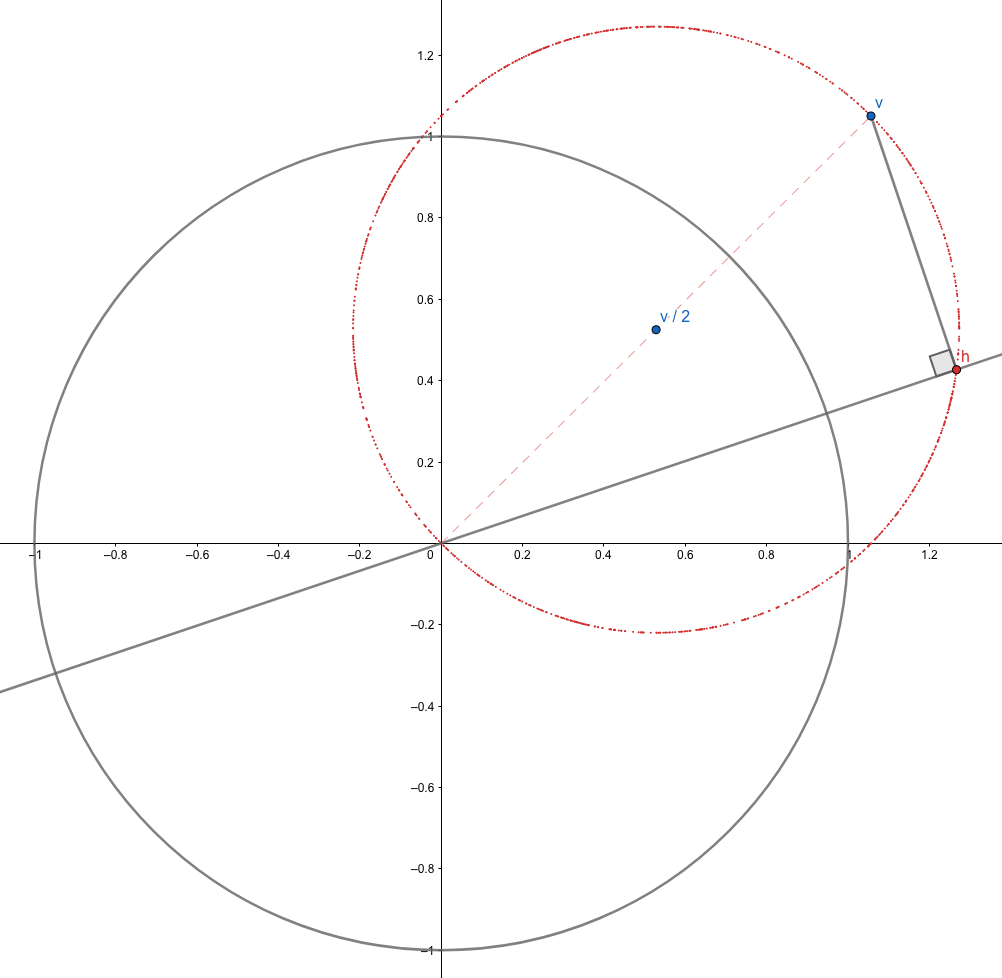The random subspace in this case is illustrated by the straight gray line, which determines the projection $$P(\bs{v})=\bs{h}$$ as in the graph. We know we're now uniformly selecting this subspace when we rotate this line centered at the origin accordingly. This means the angle between $$\bs{v}$$ and $$\bs{h}$$, denoted by $$\theta$$, is a uniform random variable on $$[0, 2\pi)$$. Further, simple geometry tells us the angle between $$\bs{v}$$ and $$\bs{h} - \bs{v}/2$$ is merely $$2\theta$$, which is therefore, also uniformly distributed on $$[0, 4\pi)$$. Now that we know $$\bs{h}$$ uniformly lies on the red circle, we conclude the expected projection, in this particular case, is $$\bs{v}/2$$.

While it gets geometrically difficult to imagine, not to mention to draw, the case of larger $$n$$ and $$k$$, this example has given us a pretty nice guess:

$\E[P(v)] = \frac{k}{n}\bs{v}.$

Can we prove it in higher dimensions and general cases?

# Rigorous Proof

Proof. Now we try to prove that our previous statement is true. For any set of orthogonal bases $$\bs{e}=(\bs{e}_1,\bs{e}_2,\dots,\bs{e}_n)\in\R^{n\times n}$$, we uniformly choose a subset $$(\bs{e}_{n_1}, \bs{e}_{n_2},\dots,\bs{e}_{n_k})$$ and define a subspace $$\R^k$$ on them. The projected value on any basis $$\bs{e}_j$$ is $$\bs{e}_j'\bs{v}$$ and the corresponding vector component would be $$\bs{e}_j\bs{e}_j'\bs{v}$$. Therefore, the orthogonal projection of $$\bs{v}$$ is given by

$P(\bs{v}) = \sum_{j=1}^{k}\bs{e}_{n_j}\bs{e}_{n_j}'\bs{v} = \bs{eDe'v} \in \R^n$

where we define the random matrix $$\bs{D}$$ to be a diagonal matrix with $$k$$ ones and $$(n-k)$$ zeros on its diagonal. The diagonal entries are not independent, but the expectation of each entry would be the same, namely $$k/n$$. The expectation of the projection, therefore, is

$\E[P(\bs{v})] = \E[\bs{eDe'v}] = \E\{\E[\bs{eDe'v}\mid \bs{e}]\} = \E\{\bs{e}\E[\bs{D}]\bs{e'v}\} = \frac{k}{n}\E[\bs{ee'}]\bs{v}.$

where we used the tower rule. Now, notice for any $$\bs{e}$$ it always holds that $$\bs{e'e}=\bs{I}$$, we have

$(\bs{ee'})^2 = \bs{ee'ee'} = \bs{e(e'e)e'} = \bs{eIe'} = \bs{ee'} \Rightarrow \bs{ee'} = \bs{I}$

and thus we may finally conclude

$\E[P(\bs{v})] = \frac{k}{n}\E[\bs{ee'}]\bs{v} = \frac{k}{n}\bs{v}$

which exactly coincides with our previous guess.Q.E.D.

# Intuitive Implications

TBD. May concern dimensional reduction, etc.

1. 1.Points and vectors are denoted in bold and defined as column vectors unless otherwise stated. ↩︎
2. 2.When we say $$\bs{a}=(a_1,a_2,\dots,a_n)$$, we're talking about a row vector of $$n$$ entries. ↩︎
3. 3.For any r.v. $$X$$, we claim $$\E[X] = \E\{\E[X\mid Y]\}$$. Also known as the law of total expectation. ↩︎
]]>
<p>A couple of months ago I was asked the following question during an interview (for propriatary concerns I'm not gonna disclose the industry or name of the company): <span class="math inline">$$\newcommand{R}{\mathbb{R}} \newcommand{E}{\text{E}} \newcommand{bs}{\boldsymbol} \newcommand{N}{\mathbb{N}}$$</span></p> <blockquote> <p>Assume <span class="math inline">$$k$$</span>, <span class="math inline">$$n\in\N$$</span> and <span class="math inline">$$k &lt; n$$</span>. For a uniformly chosen subspace <span class="math inline">$$\R^k\subsetneq\R^n$$</span> we define the orthogonal projection as <span class="math inline">$$P:\R^n\mapsto\R^n$$</span>. Find <span class="math inline">$$\E[P(\bs{v})]$$</span> where <span class="math inline">$$\bs{v}\in\R^n$$</span> is given.</p> </blockquote> <p>It's an interesting question and also a totally novel one to me at that time. How do we define a "uniformly" chosen subspace and its corresponding projection? What are the possible intuitions in this simple piece of question? Despite the busy schoolwork and student projects, these thoughts persist in my mind and drive me digging this question from time to time. Curiosity has been aroused and an appetite is meant to be satisfied.</p>
Notes on Foreign Exchange http://allenfrostline.com/2019/02/21/notes-on-foreign-exchange/ 2019-02-22T05:08:49.000Z 2019-03-17T22:46:06.737Z

These are the lecture notes on foreign exchange market and theories. $$\newcommand{\E}{\text{E}} \newcommand{\P}{\text{P}} \newcommand{\Q}{\text{Q}} \newcommand{\F}{\mathcal{F}} \newcommand{\d}{\text{d}} \newcommand{\N}{\mathcal{N}} \newcommand{\eeq}{\ \!=\mathrel{\mkern-3mu}=\ \!} \newcommand{\eeeq}{\ \!=\mathrel{\mkern-3mu}=\mathrel{\mkern-3mu}=\ \!} \newcommand{\MGF}{\text{MGF}}$$

# Pricing in Theory

### Notations

• $$d$$ $$\equiv$$ superscript for domestic currency
• $$f$$ $$\equiv$$ superscript for foreign currency
• $$t$$ $$\equiv$$ current time
• $$T$$ $$\equiv$$ delivery time
• $$S_t$$ $$\equiv$$ spot price of a foreign currency in domestic currency
• $$N$$ $$\equiv$$ number of units in transaction
• $$R$$ $$\equiv$$ fixed contract rate
• $$r$$ $$\equiv$$ interest rate
• $$PV$$ $$\equiv$$ present value
• $$P$$ $$\equiv$$ PV of a zero-coupon bond
• $$D$$ $$\equiv$$ currency deposit (money market account)

### FX Spot Price

The spot price of a foreign currency is (LHS as units of foreign currency, RHS as of domestic currency) $$1 = S_t$$. Which is equivalent to $$1/S_t = 1$$. We say $$S_t$$ is a price in domestic terms.

### FX Spot Contract

Selling domestic currency to buy foreign currencies.

### Value of a Spot Contract

Value for the buyer is (in domestic currency) $$PV=(S_t - R)N$$. This is because of the two cash flows:

• $$+N$$ (foreign currency)
• $$-N\cdot R$$ (domestic currency).

### Zero-Coupon Bonds

• Domestic: $$P^d(t,T) =\exp[-(T-t)r^d(t, T)]$$
• Domestic: $$P^f(t,T) =\exp[-(T-t)r^f(t, T)]$$

### FX Forward Contract

Executing a spot contract at time $$T$$ with given contract rate $$R$$.

### Value of a Forward Contract

Value for the buyer is (in domestic currency) $$PV=(S_t\cdot P^f - R\cdot P^d)N$$. This is because of the two cash flows at time $$T$$:

• $$+N$$ (foreign currency)
• $$-N\cdot R$$ (domestic currency)

which has present values at time $$t$$

• $$+N\cdot P^f$$ (foreign currency)
• $$-N\cdot R\cdot P^d$$ (domestic currency)

### Forward Rate

We set $$PV=0$$ for the forward contract and get $$F\equiv R=S_t\cdot P^f /\ P^d=S_t\exp[(r^d - r^f)\cdot(T-t)]$$. Therefore, we also have $$F-S_t\approx S_t(r^d - r^f)\cdot(T-t)$$.

### Covered Interest Rate Parity (CIP)

In order to replicate a forward contract, we can execute a spot contract, borrow domestic and lend foreign. Namely, we have cash flows at time $$t$$:

• $$+1-1\equiv 0$$ (foreign currency)
• $$+S_t-S_t\equiv 0$$ (domestic currency)

and at time $$T$$:

• $$+1/ P^f$$ (foreign currency)
• $$-S_t / P^d$$ (domestic currency)

This yields $$S_t/P^dF=F\cdot 1 / P^f$$, or $$F=S_t\cdot P^f / P^d$$, which is what we call the CIP. This means higher interest rate currencies will be "weaker" on a forward basis.

### Implied Yields

From the CIP we have $$P^f = P^d \cdot F/S_t$$, which gives $$r^f = r^d - \log(F/S_t) / (T-t)$$.

### FX Swap Contract

Swapping a forward contract ($$T_1$$, $$R_1$$) for another ($$T_2$$, $$R_2$$).

### Value of a Swap Contract

Value for the buyer is (in domestic currency) \begin{align*}PV&=(S_t\cdot P^{f1} - R_1\cdot P^{d1} - S_t\cdot P^{f2} + R_2\cdot P^{d2})\\&=\left\{S_t\left[\exp(-r^{f1}(T_1-t)) - \exp(-r^{f2}(T_2-t))\right] - R_1\exp(r^{d1}(T_1-t)) + R_1\exp(r^{d1}(T_1-t))\right\}\cdot N\end{align*} which is rather insensitive w.r.t. the spot rate: $PV_S = \frac{\partial PV}{\partial S} = (P^{f1} - P^{f2})N = \left[\exp(-r^{f1}(T_1-t)) - \exp(-r^{f2}(T_2-t))\right]\cdot N\approx N r^f(T_2 -T_1)$ compared with that of a forward contract: $PV_S = P^f\cdot N = \exp[-r^f(T-t)]\cdot N \approx N.$

### FX Option Contract

The right (but not obligation) to exhcange $$N$$ units of foreign currency for $$N\cdot K$$ units of domestic currency at time $$T$$. This is to say, we call the right to buy foreign currency as a foreign call, but in the meantime, also a domestic put.

### Value of an Option Contract

We have the put-call parity as $$C-P=P^d(F-K)$$ and the payoff of a foreign call option, $$\max(0, S_T-K)$$. We assume $$\{S_t\}_{0\le t\le T}$$ follows GBM $$\d S = \mu S \d t + \sigma S \d W$$ which, according to Itô's lemma, gives $\d V = \left(\frac{1}{2}\sigma^2S^2V_{SS} + V_t\right) \d t + V_S\d S$ where $$V$$ is any derivative w.r.t. $$S$$ (remark: remember that all subscript $$t$$ here denote derivatives w.r.t. $$t$$, not time). Now, noticing the hedged portfolio $$\Pi = \{+1 \text{ unit of }V; -V_S \text{ units of } D^f\}$$ has dynamics \begin{align*}\d\Pi &= \d V - V_S\d (S\cdot D^f) \\&= \left(\frac{1}{2}\sigma^2S^2V_{SS} + V_t\right) \d t + V_S\d S - V_S(D^f \d S + S\cdot r^f \d t) \\&= \left(\frac{1}{2}\sigma^2S^2V_{SS} + V_t - r^fV_S S\right) \d t\end{align*} where we used the fact that $$D^f(t)=1$$. Now under risk-neutral measure, we know $\left(\frac{1}{2}\sigma^2S^2V_{SS} + V_t - r^fV_S S\right)\d t = r^d(V - V_S S)\d t$ which gives the so-called Garman-Kohlhagen PDE: $\frac{1}{2}\sigma^2S^2V_{SS} + (r^d - r^f)V_S S - r^d V + V_t = 0$ with boundary conditions $$V(S_T,T)=(S_T-K)^+$$ and $$V(0,T)=0$$.

# Pricing in Practice

### FX Spot Contract: Dates

Trade date is when the terms of the transaction are agreed. Currency trading is a global, 24-hour market. The "trading day" ends at 5pm New York time. Value date is when cash flows occur, i.e., when currencies are delivered. Value date for spot transactions is "T+2" for most currency pairs. However, spot value date is "T+1" for USD versus CAD, RUB, TRY, PHP.

Trade terms are agreedTwo currency payments are delivered
Good day for CCY1 and CCY2 if non-USDGood day for CCY1 and CCY2
Can be a USD holidayCannot be a USD holiday

### Currency Pairs (CCY1/CCY2)

We usually call currency pairs (CCY1/CCY2, usually "/" is omitted) as any of the following:

CCY1CCY2
Base CurrencyTerms Currency
Fixed CurrencyVariable Currency
Home CurrencyOverseas Currency

When we say EURUSD $$= 1.1860$$, we mean $$1$$ EUR $$=$$ $$1.1860$$ USD.

In the context of bid offer spreads, we denote the bid and offer prices as EURUSD $$=1.1859/1.1860$$ (or $$1.1859/60$$ as shorthand). These spreads may vary. The possible reasons may involve liquidity, volatility and cost of risk.

### Direct and Indirect Quotes

In terms of USD, the direct quotes are CCYUSD and the indirect quotes are USDCCY.

### LHS and RHS

• LHS (left-hand side) $$=$$ market-maker's bid
• RHD (right-hand side) $$=$$ market-maker's offer

### Cross Rates

Spot rates calculated from an indirect market, e.g. when EURUSD $$=1.1882$$ and USDJPY $$=109.14$$, then we have cross rate EURJPY $$=129.68$$, which does not necessarily coincides with the actual rate in the market.

### Day Count for Money Market

• For tenors of $$1$$ year and under: ACT/360 or ACT/365, where ACT $$=$$ actual (number of days)
• For tenors of years (e.g. bonds): 30/360

### Interest Rate used with FX Forwards

It's neither of the interest rates of the two currencies. Instead, people use the deposit rate in this case, specifically in terms of USD, it's the Eurodollar deposit rate, or LIBOR.

### FX Forward Contract

Contracts with any delivery date (a.k.a. value date) other than spot are considered forward. Standard delivery dates may be in weeks or months, and otherwise called "broken". Specifically, we call the contract "cash" if its delivery date is today, and "tom" if it's tomorrow. FX forwards are OTC (over-the-counter).

### Forward Point

We define: $$\text{forward point} = \text{forward rate (outright)} - \text{spot rate}$$. The number is usually scaled by $$10^4$$.

### Covered Interest Rate Parity (CIP) with Interest Rates

We have $\text{forward} =\text{spot} \times \frac{1 + R_{\text{variable CCY}}\times\text{days}/ 360}{1 + R_{\text{fixed CCY}}\times\text{days}/360}$ where we use $$R$$ instead of $$P$$ here as it's more commonly given.

### Implied Yields with Interest Rates

Using the CIP above, we have $R^f = \frac{(S/F)\times(1 +R_d\times\text{days}/360) - 1}{\text{days}/360}$ where we assume the rates are not compounded.

### FX Swap Contract

Contracts that alter the value date on an existing trade by simultaneously executing two forward transactions.

### Risks For Spot, Forward and Swap

# of legsFX RiskIR Spread Risk
Spot1YesNo
Forward1YesYes
Swap2NoYes

### Swap Point

We define: $$\text{swap point} = \text{far rate} - \text{near rate}$$.

To be continued.

]]>
<p>These are the lecture notes on foreign exchange market and theories. <span class="math inline">$$\newcommand{\E}{\text{E}} \newcommand{\P}{\text{P}} \newcommand{\Q}{\text{Q}} \newcommand{\F}{\mathcal{F}} \newcommand{\d}{\text{d}} \newcommand{\N}{\mathcal{N}} \newcommand{\eeq}{\ \!=\mathrel{\mkern-3mu}=\ \!} \newcommand{\eeeq}{\ \!=\mathrel{\mkern-3mu}=\mathrel{\mkern-3mu}=\ \!} \newcommand{\MGF}{\text{MGF}}$$</span></p>
Notes on Stochastic Calculus http://allenfrostline.com/2019/02/21/notes-on-stochastic-calculus/ 2019-02-22T05:08:38.000Z 2019-03-13T22:20:40.643Z

This is a brief selection of my notes on the stochastic calculus course. Content may be updated at times. $$\newcommand{\E}{\text{E}} \newcommand{\P}{\text{P}} \newcommand{\Q}{\text{Q}} \newcommand{\F}{\mathcal{F}} \newcommand{\d}{\text{d}} \newcommand{\N}{\mathcal{N}} \newcommand{\sgn}{\text{sgn}} \newcommand{\tr}{\text{tr}} \newcommand{\bs}{\boldsymbol} \newcommand{\eeq}{\ \!=\mathrel{\mkern-3mu}=\ \!} \newcommand{\eeeq}{\ \!=\mathrel{\mkern-3mu}=\mathrel{\mkern-3mu}=\ \!} \newcommand{\R}{\mathbb{R}} \newcommand{\MGF}{\text{MGF}}$$

# MGF of Normal Distribution

For $$X\sim\N(\mu,\sigma^2)$$, we have $$\MGF(\theta)=\exp(\theta\mu + \theta^2\sigma^2/2)$$. We have $$\E(X^k) = \MGF^{\ (k)}(0)$$.

# Truncated Normal Distribution

Consider a two-sided truncation $$(a,b)$$ on $$\N(\mu,\sigma^2)$$, then $\E[X\mid a < X < b] = \mu - \sigma\frac{\phi(\alpha) - \phi(\beta)}{\Phi(\alpha) - \Phi(\beta)}$ where $$\alpha:=(a-\mu)/\sigma$$ and $$\beta:=(b-\mu)/\sigma$$.

# Doob's Identity

Let $$X$$ be a MG and $$T$$ a stopping time, then $$\E X_{T\wedge n} = \E X_0$$ for any $$n$$.

# Matingale Transform

Define $$(Z\cdot X)_n:=\sum_{i=1}^n Z_i(X_i - X_{i-1})$$ where $$X$$ is MG with $$X_0=0$$ and $$Z_n$$ is predictable and bounded, then $$(Z\cdot X)$$ is MG. If $$X$$ is sub-MG, then also is $$(Z\cdot X)$$. Furthermore, if $$Z\in[0,1]$$, then $$\E(Z\cdot X)\le \E X$$.

# Common MGs

• $$S_n:=\sum_{i=1}^n X_i$$ for $$X\sim(0,\sigma^2)$$ (this is called symmetric RW)
• $$S_n^2 - n\sigma^2$$ for symmetric RW $$S_n$$
• $$\exp(\theta S_n)\ /\ \MGF_X(\theta)$$ for symmetric RW $$S_n$$
• $$(q/p)^{S_n}$$ for assymetric RW $$S_n$$ ($$P(X=1)=p$$, $$P(X=-1)=q=1-p$$)
• $$S_n - n (p-q)$$ for assymetric RW $$S_n$$
• $$B_t^2 - t$$ for standard BM $$B_t$$
• $$\exp(\theta B_t - \theta^2 t / 2)$$ for standard BM $$B_t$$
• $$\exp(-2\mu B_t)$$ for $$B_t = W_t + \mu t$$ where $$W_t$$ is standard BM

# Convex Mapping

If $$X$$ is MG and $$\phi(\cdot)$$ is a convex function, then $$\phi(X)$$ is sub-MG.

# $$L^p$$ and $$L^p$$ Boundedness

• $$X$$ is an $$L^p$$ MG if $$\E X_n^p$$ is finite for all $$n$$.
• $$X$$ is an $$L^p$$-bounded MG if $$\sup_{n\ge 0}\E X_n^2$$ is finite.

# Doob's Maximal Ineq.

• $$L^1$$: if $$X$$ is a non-negative sub-MG with $$X_0=0$$, then $$\P(\max_{i\le n} X_i\ge \alpha)\le\E X_n / \alpha$$.
• $$L^2$$: if $$X$$ is an $$L^2$$ MG with $$X_0=0$$, then $$\P(\max_{i\le n} X_i\ge \alpha)\le \E X_n^2 / \alpha^2$$.
• $$L^2$$-bounded: if $$X$$ is $$L^2$$-bounded, then $$\P(\sup_{n\ge 0}|X_n|\ge \alpha) \le \sup_{n\ge 0}\E X_n^2 / \alpha^2$$.

# MG Convergence Theorem

• $$L^1$$-bounded: $$\exists X_{\infty}\in L^1$$ s.t. $$\lim_{n\to\infty}X_n \overset{\text{a.s.}}{\eeq} X_{\infty}$$.
• $$L^2$$-bounded: $$\exists X_{\infty}\in L^2$$ s.t. $$\lim_{n\to\infty}X_n \overset{\text{a.s.}}{\eeq} X_{\infty}$$, $$\lim_{n\to\infty}\E(X_n - X_{\infty})^2=0$$ and $$\lim_{n\to\infty}\E X_n^2 = E X_{\infty}^2$$.

# Change of Measure

Given $$\P$$-measure, we define the likelihood ratio $$Z:=\d\Q / \d\P$$ for another measure $$\Q$$. Then we have

• $$\E_{\P} Z = 1$$.
• $$\E_{\Q} Y = \E_{\P} (ZY)$$ for all $$Y$$. Specifically, for $$Y=\textbf{1}_{\omega}$$ we have $$\Q(\omega) = \E_{\P}(Z\textbf{1}_{\omega}) \overset{\text{discr.}}{\eeeq} \P(\omega)Z(\omega)$$.
• Example (changing numeraire from CASH $$\P$$- to STOCK $$\Q$$-measure): $$Z(\omega) = (\d\Q/\d\P)(\omega) = S_N(\omega) / S_0$$.
• Example (importance sampling): $$\P_{.5}(S_{100} > 80) = \E_{.8}\left(\textbf{1}_{S_{100} > 80}\cdot \frac{.5^{100}}{.8^{S}.2^{100-S}}\right)$$.
• Example (foreign exchange): $$\d\Q_A/\d\Q_B = A_TY_T/B_TY_0$$ where $$Y_t$$ is the exchange rate, i.e. the number of currency $$B$$ in exchange for $$1$$ unit of currency $$A$$.

# Cameron-Martin

• Theorem: given $$B$$ is standard BM under $$\P_0$$ measure, then $$\exists \P_{\theta}$$ s.t. $$Z_T^{\theta} := \d\P_{\theta}/\d\P_0 = \exp(\theta B_T - \theta^2 T / 2)$$, under which $$\{B_t\}_{t\le T}$$ is a BM with drift $$\theta$$ and variance $$1$$.
• Corollary: given any two drift $$\theta$$ and $$\eta$$, define similarly $$Z_T:=\d\P_{\theta}/\d\P_{\eta}$$ and then for any stopping time $$\tau$$, we have $$\P_{\theta}(\tau\le T) = \E_{\eta}(\textbf{1}_{\tau\le T}Z_T)$$.
• Example: given $$B$$ is a BM with drift $$-b < 0$$, now define $$T=\tau(a)$$ for some $$a > 0$$, then we have $$\P_{-b}(T < \infty) = \E_{+b}[\exp(-2b B_T) \textbf{1}_{T < \infty}] = \exp(-2ab)$$.

# Strong Markov Property

If $$B$$ is a BM and $$T=\tau(\cdot)$$ is a stopping time, then $$\{B_{t+T} - B_T\}_{t\ge T}$$ is a BM indep. of $$\{B_t\}_{t\le T}$$.

# Orthogonal Transform

If $$B$$ is a standard $$k$$-BM and $$U\in\mathbb{R}^{k\times k}$$ is orthogonal, then $$UB$$ is also a standard $$k$$-BM.

# Doob's Decomposition

For any sub-MG $$X$$, we have unique decomposition $$X=M+A$$ where $$M_n:=X_0 + \sum_{i=1}^n [X_i - \E(X_i\mid \F_{i-1})]$$ is a martingale and $$A_n:=\sum_{i=1}^n[\E(X_i\mid \F_{i-1}) - X_{i-1}]$$ is a non-decreasing predictable sequence.

# Gambler's Ruin

• Symmetric: for (discrete- or continuous-time) MG $$S$$ with $$S_0=0$$, define stopping time $$T=\min\{\tau(-A), \tau(B)\}$$ then $$\P(S_T=B)=\frac{A}{A+B}$$, $$\P(\tau(B)<\infty)=\lim_{A\to\infty}\P(S_T=B)=1$$ and $$\E T = AB$$.
• Assymetric ($$p<q$$): for RW $$S_n:=S_0 + \sum_{i=1}^{n}X_i$$ with $$S_0=0$$ and $$\P(X=+1)=p$$, $$\P(X=-1)=q=1-p$$, define similarly $$T$$, then $$\P(S_T=B)=\frac{1-(q/p)^{-A}}{(q/p)^{B} - (q/p)^{-A}}$$, $$\P(\tau(B)<\infty)=\lim_{A\to\infty}\P(S_T=B)=\left(p/q\right)^{B}$$ and $$\E T = \frac{\E S_T}{q-p}$$.

# Reflection Principle

For BM $$B$$ and stopping time $$T=\tau(a)$$, define $$B^*$$ s.t. $$B_t^*=B_t$$ for all $$t\le T$$ and $$B_t^* = 2a - B_t$$ for all $$t>T$$, then $$B^*$$ is also a BM.

# First Passage Time $$T:=\tau(a)$$

• CDF: $$\P(T \le t) = 2\P(B_t > a) = 2\Phi(-a / \sqrt{t})$$.
• PDF: follows from CDF
• $$\E T$$: $$X_t:=\exp(\theta B_t - \theta^2 t / 2)$$ is a MG, we know $$\E(X_T)=X_0 = 1$$, from which expectation is calculated.

# Joint Distribution of BM and its Maximum

$$\P(\max_{s\le t}B_s > x\text{ and }B_t < y) = \Phi\!\left(\frac{y-2x}{\sqrt{t}}\right)$$.

# $$2$$-BM Stopped on 1 Boundary

Let $$X$$ and $$Y$$ be indep. BM. Note that for all $$t\ge 0$$, from exponential MG we know $$\E[\exp(i\theta X_t)]=\exp(-\theta^2 t/2)$$. Now define $$T=\tau(a)$$ for $$Y$$ and we have $$\E[\exp(i\theta X_T)] = \E[\exp(-\theta^2 T /2)]=\exp(-|\theta| a)$$, which is the Fourier transform of the Cauchy density $$f_a(x)=\frac{1}{\pi}\frac{a}{a^2+x^2}$$.

# Itô Integral

We define Itô integral $$I_t(X) := \int_0^t\! X_s\d W_s$$ where $$W_t$$ is a standard Brownian process and $$X_t$$ is adapted.

# Martingality of Itô Integral

• $$I_t(X)$$ is a martingale
• $$I_t(X)^2 - [I(X), I(X)]_t$$ is a martingale, where $$[I(X), I(X)]_t := \int_0^t\! X_s^2\d s$$

# Itô Isometry

This is the direct result from the second martingality property above. Let $$X_t$$ be nonrandom and continuously differentiable, then $\E\!\left[\!\left(\int_0^t X_t\d W_t\right)^{\!\!2}\right] = \E\!\left[\int_0^t X_t^2\d t\right].$

# Itô Formula - $$f(W_t)$$

Let $$W_t$$ be a standard Brownian motion and let $$f:\R\mapsto\R$$ be a twice-continously differentiable function s.t. $$f$$, $$f'$$ and $$f''$$ are all bounded, then for all $$t>0$$ we have $\d f(W_t) = f'(W_t)\d W_t + \frac{1}{2}f''(W_t) \d t.$

# Itô Formula - $$f(t,W_t)$$

Let $$W_t$$ be a standard Brownian motion and let $$f:[0,\infty)\times\R\mapsto\R$$ be a twice-continously differentiable function s.t. its partial derivatives are all bounded, then for all $$t>0$$ we have $\d f(t, W_t) = f_x\d W_t + \left(f_t + \frac{1}{2}f_{xx}\right) \d t.$

# Wiener Integral

The Wiener integral is a special case of Itô integral where $$f(t)$$ is here a nonrandom function of $$t$$. Variance of a Wiener integral can be derived using Itô isometry.

# Itô Process

We say $$X_t$$ is an Itô process if it satisfies $\d X_t = Y_t\d W_t + Z_t\d t$ where $$Y_t$$ and $$Z_t$$ are adapted and $$\forall t$$ $\int_0^t\! \E Y_s^2\d s < \infty\quad\text{and}\quad\int_0^t\! \E|Z_s|\d s < \infty.$ The quadratic variation of $$X_t$$ is $[X,X]_t = \int_0^t\! Y_s^2\d s.$

# Itô Product and Quotient

Assume $$X_t$$ and $$Y_t$$ are two Itô processes, then $\frac{\d (XY)}{XY} = \frac{\d X}{X} + \frac{\d Y}{Y} + \frac{\d X\d Y}{XY}$ and $\frac{\d (X/Y)}{X/Y} = \frac{\d X}{X} - \frac{\d Y}{Y} + \left(\frac{\d Y}{Y}\right)^{\!2} - \frac{\d X\d Y}{XY}.$

# Brownian Bridge

A Brownian bridge is a continuous-time stochastic process $$X_t$$ with both ends pinned: $$X_0=X_T=0$$. The SDE is $\d X_t = -\frac{X_t}{1-t}\d t + \d W_t$ which solves to $X_t = W_t - \frac{t}{T}W_T.$

# Itô Formula - $$u(t, X_t)$$

Let $$X_t$$ be an Itô process. Let $$u(t,x)$$ be a twice-continuously differentiable function with $$u$$ and its partial derivatives bounded, then $\d u(t, X_t) =\frac{\partial u}{\partial t}(t, X_t)\d t +\frac{\partial u}{\partial x}(t, X_t)\d X_t +\frac{1}{2}\frac{\partial^2 u}{\partial x^2}(t, X_t)\d [X,X]_t.$

# The Ornstein-Uhlenbeck Process

The OU process describes a stochastic process that has a tendency to return to an "equilibrium" position $$0$$, with returning velocity proportional to its distance from the origin. It's given by SDE $\d X_t = -\alpha X_t \d t + \d W_t \Rightarrow\d [\exp(\alpha t)X_t] = \exp(\alpha t)\d W_t$ which solves to $X_t = \exp(-\alpha t)\left[X_0 + \int_0^t\! \exp(as)\d W_s\right].$

Remark: In finance, the OU process is often called the Vasicek model.

# Diffusion Process

The SDE for general diffusion process is $$\d X_t = \mu(X_t)\d t + \sigma(X_t)\d W_t$$.

# Hitting Probability for Diffusion Processes

In order to find $$\P(X_T=B)$$ where we define $$T=\inf\{t\ge 0: X_t=A\text{ or }B\}$$, we consider a harmonic function $$f(x)$$ s.t. $$f(X_t)$$ is a MG. This gives ODE $f'(x)\mu(x) + f''(x)\sigma^2(x)/2 = 0\Rightarrowf(x) = \int_A^x C_1\exp\left\{-\!\int_A^z\frac{2\mu(y)}{\sigma^2(y)}\d y\right\}\d z + C_2$ where $$C_{1,2}$$ are constants. Then since $$f(X_{T\wedge t})$$ is a bounded MG, by Doob's identity we have $\P(X_T=B) = \frac{f(X_0) - f(A)}{f(B) - f(A)}.$

# Multivariable Itô Formula - $$u(\bs{W}_t)$$

Let $$\bs{W_t}$$ be a $$K$$-dimensional standard Brownian motion. Let $$u:\R^K\mapsto \R$$ be a $$C^2$$ function with bounded first and second partial derivatives. Then $\d u(\bs{W}_t) = \nabla u(\bs{W}_t)\cdot \d \bs{W}_t + \frac{1}{2}\tr[\Delta u(\bs{W}_t)] \d t$ where the gradient operator $$\nabla$$ gives the vector of all first order partial derivatives, and the Laplace operator (or Laplacian) $$\Delta\equiv\nabla^2$$ gives the vector of all second order partial derivatives.

# Dynkin's Formula

If $$T$$ is a stopping time for $$\bs{W_t}$$, then for any fixed $$t$$ we have $\E[u(\bs{W}_{T\wedge t})] = u(\bs{0}) + \frac{1}{2}\E\!\left[\int_0^{T\wedge t}\!\!\Delta u(\bs{W}_s)\d s\right].$

# Harmonic Functions

A $$C^2$$ function $$u:\R^k\mapsto\R$$ is said to be harmonic in a region $$\mathcal{U}$$ if $$\Delta u(x) = 0$$ for all $$x\in \mathcal{U}$$. Examples are $$u(x,y)=2\log(r)$$ and $$u(x,y,z)=1/r$$ where $$r$$ is defined as the norm.

Remark: $$f$$ being a harmonic function is equivalent to $$f(X_t)$$ being a MG, i.e. $$f'(x)\mu(x) + f''(x)\sigma^2(x)/2 = 0$$ for a diffusion process $$X_t$$.

# Harmonic Corollary of Dynkin

Let $$u$$ be harmonic in the an open region $$\mathcal{U}$$ with compact support, and assume that $$u$$ and its partials extend continuously to the boundary $$\partial \mathcal{U}$$. Define $$T$$ to be the first exit time of Brownian motion from $$\mathcal{U}$$. for any $$\bs{x}\in\mathcal{U}$$, let $$\E^{\bs{x}}$$ be the expectation under measure $$\P^{\bs{x}}$$ s.t. $$\bs{W}_t - \bs{x}$$ is a $$K$$-dimensional standard BM. Then

• $$u(\bs{W}_{T\wedge t})$$ is a MT.
• $$\E_{\bs{x}}[u(\bs{W}_T)] = u(\bs{x})$$.

# Multivariate Itô Process

A multivariate Itô process is a continuous-time stochastic process $$X_t\in\R$$ of the form $X_t = X_0 + \int_0^t\! M_s \d s + \int_0^t\! \bs{N}_s\cdot \d \bs{W}_s$ where $$\bs{N}_t$$ is an adapted $$\R^K$$−valued process and $$\bs{W}_t$$ is a $$K$$−dimensional standard BM.

# General Multivariable Itô Formula - $$u(\bs{X}_t)$$

Let $$\bs{W}_t\in\R^K$$ be a standard $$K$$−dimensional BM, and let $$\bs{X}_t\in\R^m$$ be a vector of $$m$$ multivariate Itô processes satisfying $\d X_t^i = M_t^i\d t + \bs{N}_t^i\cdot \d \bs{W}_t.$ Then for any $$C^2$$ function $$u:\R^m\mapsto\R$$ with bounded first and second partial derivatives $\d u(\bs{X}_t) = \nabla u(\bs{X}_t)\cdot \d \bs{X}_t + \frac{1}{2}\tr[\Delta u(\bs{X}_t)\cdot \d [\bs{X},\bs{X}]_t].$

# Knight's Theorem

Let $$\bs{W}_t$$ be a standard $$K$$−dimensional BM, and let $$\bs{U}_t$$ be an adapted $$K$$−dimensional process satisfying $|{\bs{U}_t}| = 1\quad\forall t\ge 0.$ Then we know the following $$1$$-dimensional Itô process is a standard BM: $X_t := \int_0^t\!\! \bs{U}_s\cdot \d W_s.$

Let $$\bs{W}_t$$ be a standard $$K$$−dimensional BM, and let $$R_t=|\bs{W}_t|$$ be the corresponding radial process, then $$R_t$$ is a Bessel process with parameter $$(K-1)$$ given by $\d R_t = \frac{K-1}{R_t}\d t + \d W_t^{\sgn}$ where we define $$\d W_t^{\sgn} := \sgn(\bs{W}_t)\cdot \d \bs{W}_t$$.

A Bessel process with parameter $$a$$ is a stochastic process $$X_t$$ given by $\d X_t = \frac{a}{X_t}\d t+ \d W_t.$ Since this is just a special case of diffusion processes, we know the corresponding harmonic function is $$f(x)=C_1x^{-2a+1} + C_2$$, and the hitting probability is $\P(X_T=B) = \frac{f(X_0) - f(A)}{f(B) - f(A)} =\begin{cases}1 & \text{if }a > 1/2,\$$x/B)^{1-2a} & \text{otherwise}.\end{cases}$ # Itô's Representation Theorem Let \(W_t$$ be a standard $$1$$-dimensional Brownian motion and let $$\F_t$$ be the $$\sigma$$−algebra of all events determined by the path $$\{W_s\}_{s\le t}$$. If $$Y$$ is any r.v. with mean $$0$$ and finite variance that is measurable with respect to $$\F_t$$, then for some $$t > 0$$ $Y = \int_0^t\! A_s\d W_s$ for some adapted process $$A_t$$ that satisfies $\E(Y^2) = \int_0^t\! \E(A_s^2)\d s.$ This theorem is of importance in finance because it implies that in the Black-Sholes setting, every contingent CLAIM can be hedged.

Special case: let $$Y_t=f(W_t)$$ be any mean $$0$$ r.v. with $$f\in C^2$$. Let $$u(s,x):=\E[f(W_t)\mid W_s = x]$$, then $Y_t = f(W_t) = \int_0^t\! u_x(s,W_s)\d W_s.$

# Assumptions of the Black-Scholes Model

• No arbitrage
• Riskless asset CASH with non-random rate of return $$r_t$$
• Risky asset STOCK with share price $$S_t$$ such that $$\d S_t = S_t(\mu_t \d t + \sigma \d W_t)$$

# Black-Scholes Model

Under a risk-neutral measure $$\P$$, the discounted share price $$S_t / M_t$$ is a martingale and thus $\frac{S_t}{M_t} = \frac{S_0}{M_0}\exp\left\{\sigma W_t - \frac{\sigma^2t}{2}\right\}$ where we used the fact that $$\mu_t = r_t$$ by the Fundamental Theorem.

# Contingent Claims

A European contingent CLAIM with expiration date $$T > 0$$ and payoff function $$f:\R\mapsto\R$$ is a tradeable asset that pays $$f(S_T)$$ at time $$T$$. By the Fundamental Theorem we know the discounted share price of this CLAIM at any $$t\le T$$ is $$\E[f(S_T)/M_T\mid \F_t]$$. In order to calculate this conditional expectation, let $$g(W_t):= f(S_t)/M_t$$, then by the Markov property of BM we know $$\E[g(W_T)\mid \F_t] = \E[g(W_t + W_{T-t}^*)\mid \F_t]$$ where $$W_t$$ is adapted in $$\F_t$$ and independent of $$W_t^*$$.

# Black-Scholes Formula

The discounted time−$$t$$ price of a European contingent CLAIM with expiration date $$T$$ and payoff function $$f$$ is $\E[f(S_T)/M_T\mid \F_t] = \frac{1}{M_T}\E\!\left[f\!\left(S_t\exp\!\left\{\sigma W_{T-t}^* - \frac{\sigma^2(T-t)}{2} + R_T - R_t\right\}\right)\middle|\F_t\right]$ where $$S_t$$ is adapted in $$\F_t$$ and independent of $$W_t^*$$. The expectation is calculated using normal. Note here $$R_t = \int_0^t r_s\d s$$ is the log-compound interest rate.

# Black-Scholes PDE

Under risk-neutral probability measure, the discounted share price of CLAIM is a martingale, i.e. it has no drift term. So we can differentiate $$M_t^{-1}u(t,S_t)$$ by Itô and derive the following PDE $u_t(t,S_t) + r_t S_tu_x(t,S_t) + \frac{\sigma^2S_t^2}{2}u_{xx}(t,S_t) = r_t u(t,S_t)$ with terminal condition $$u(T,S_T)=f(S_T)$$. Note here everything is under the BS model.

# Hedging in Continuous Time

A replicating portfolio for a contingent CLAIM in STOCK and CASH is given by $V_t = \alpha_t M_t + \beta_t S_t$ where $$\alpha_t = [u(t,S_t) - S_t u_x(t,S_t)]/M_t$$ and $$\beta_t = u_x(t,S_t)$$.

# Barrier Option

A barrier option pays $$\1$$ at time $$T$$ if $$\max_{t\le T} S_t \ge AS_0$$ and $$\0$$ otherwise. This is a simple example of a path-dependent option. Other commonly used examples are knock-ins, knock-outs, lookbacks and Asian options.

The time-$$0$$ price of such barrier options is calculated from \begin{align*}V_0 &= \exp(-rT)\P\!\left(\max_{t\le T} S_t \ge AS_0\right)= \exp(-rT)\P\!\left(\max_{t\le T} W_t + \mu t \ge a\right)\\&= \exp(-rT)\P_{\mu}\!\left(\max_{t\le T} W_t \ge a\right)\end{align*} where $$\mu=r\sigma^{-1} - \sigma/2$$ and $$a = \sigma^{-1}\log A$$. Now, by Cameron-Martin we know \begin{align*}\P_{\mu}\!\left(\max_{t\le T} W_t \ge a\right) &=\E_0[Z_T\cdot \mathbf{1}_{\{\max_{t\le T} W_t\ge a\}}] =\E_0[\exp(\mu W_T - \mu^2 T / 2)\cdot \mathbf{1}_{\{\max_{t\le T} W_t\ge a\}}] \\ &=\exp(- \mu^2 T / 2)\cdot \E_0[\exp(\mu W_T)\cdot \mathbf{1}_{\{\max_{t\le T} W_t\ge a\}}]\end{align*} and by reflection principle we have \begin{align*}\E_0[\exp(\mu W_T)\cdot \mathbf{1}_{\{\max_{t\le T} W_t\ge a\}}] &=e^{\mu a}\int_0^{\infty} (e^{\mu y} + e^{-\mu y}) \P(W_T - a \in \d y) \\&=\Phi(\mu\sqrt{T} - a/\sqrt{T}) + e^{2\mu a}\Phi(-\mu\sqrt{T}-a/\sqrt{T}).\end{align*}

# Exponential Process

The exponential process $Z_t = \exp\!\left\{\int_0^t\! Y_s\d W_s - \frac{1}{2}\int_0^t\! Y_s^2\d s\right\}$ is a positive MG given $\E\!\left[\int_0^t\! Z_s^2Y_s^2\d s\right] < \infty.$ Specifically, the exponential martingale is given by the SDE $$\d X_t = \theta X_t \d W_t$$.

# Girsanov's Theorem

Assume that under the probability measure $$\P$$ the exponential process $$Z_t(Y)$$ is a MG and $$W_t$$ is a standard BM. Define the absolutely continuous probability measure $$Q$$ on $$\F_t$$ with likelihood ratio $$Z_t$$, i.e. $$(\d\Q/\d\P)_{\F_t} = Z_t$$, then under $$Q$$ the process $W_t^* := W_t - \int_0^t\! Y_s\d s$ is a standard BM. Girsanov's Theorem shows that drift can be added or removed by change of measure.

# Novikov's Theorem

The exponential process $Z_t = \exp\!\left\{\int_0^t\! Y_s \d W_s - \frac{1}{2}\!\int_0^t\! Y_s^2 \d s\right\}$ is a MG given $\E\left[\exp\!\left\{\frac{1}{2}\!\int_0^t\! Y_s^2\d s\right\}\right] < \infty.$ This theorem gives another way to show whether an exponential process is a MG.

# Standard BM $$\to$$ OU Process

Assume $$W_t$$ is a standard BM under $$\P$$, define likelihood ratio $$Z_t = (\d\Q/\d\P)_{\F_t}$$ as above where $$Y_t = -\alpha W_t$$, then by Girsanov $$W_t$$ under $$\Q$$ is an OU process.

# Fundamental Principle of Statistical Mechanics

If a system can be in one of a collection of states $$\{\omega_i\}_{i\in\mathcal{I}}$$, the probability of finding it in a particular state $$\omega_i$$ is proportional to $$\exp\{-H(\omega_i)/kT\}$$ where $$k$$ is Boltzmann's constant, $$T$$ is temperature and $$H(\cdot)$$ is energy.

# Conditioned Brownian Motion

If $$W_t$$ is standard BM with $$W_0 = x \in (0, A)$$, how does $$W_t$$ behave conditional on the event that it hits $$A$$ before $$0$$? Define

• $$\P^x$$ is a measure under which $$W_0=x$$
• $$\Q^x$$ is a measure under which $$W_0=x$$ and $$W_T=A$$ where $$T=\inf\{t\ge 0: W_t=A\text{ or }0\}$$

Then the likelihood ratios are $\left(\frac{\d\Q^x}{\d\P^x}\right)_{\!\F_T} \!= \frac{\mathbf{1}_{\{W_T=A\}}}{\P^x\{W_T=x\}} \Rightarrow\left(\frac{\d\Q^x}{\d\P^x}\right)_{\!\F_{T\wedge t}} \!= \E\!\left[\left(\frac{\d\Q^x}{\d\P^x}\right)_{\!\F_T}\middle|\F_{T\wedge t}\right] = \frac{W_{T\wedge t}}{x}.$ Notice \begin{align*}\frac{W_{T\wedge t}}{x} &=\exp\left\{\log W_{T\wedge t}\right\} / x \overset{\text{Itô}}{\eeq}\exp\left\{\log W_0 + \int_0^{T\wedge t}W_s^{-1}\d W_s - \frac{1}{2}\int_0^{T\wedge t} W_s^{-2}\d s\right\} / x \\&=\exp\left\{\int_0^{T\wedge t}W_s^{-1}\d W_s - \frac{1}{2}\int_0^{T\wedge t} W_s^{-2}\d s\right\}\end{align*} which is a Girsanov likelihood ratio, so we conclude $$W_t$$ is a BM under $$\Q^x$$ with drift $$W_t^{-1}$$, or equivalently $W_t^* = W_t - \int_0^{T\wedge t}W_s^{-1}\d s$ is a standard BM with initial point $$W_0^* = x$$.

# Lévy Process

A one-dimensional Lévy process is a continuous-time random process $$\{X_t\}_{t\ge 0}$$ with $$X_0=0$$ and i.i.d. increments. Lévy processes are defined to be a.s. right continuous with left limits.

Remark: Brownian motion is the only Lévy process with continuous paths.

# First-Passage-Time Process

Let $$B_t$$ be a standard BM. Define the FPT process as $$\tau_x = \inf\{t\ge 0: B_t \ge x\}$$. Then $$\{\tau_{x}\}_{x\ge 0}$$ is a Lévy process called the one-sided stable-$$1/2$$ process. Specifically, the sample paths $$x\mapsto \tau_x$$ is non-decreasing in $$x$$. Such Lévy processes with non-decreasing paths are called subordinators.

# Poisson Process

A Poisson process with rate (or intensity) $$\lambda > 0$$ is a Lévy process $$N_t$$ such that for any $$t\ge 0$$ the distribution of the random variable $$N_t$$ is the Poisson distribution with mean $$\lambda t$$. Thus, for any $$k=0,1,2,\cdots$$ we have $$\P(N_t=k) = (\lambda t)^k\exp(-\lambda t)\ /\ k!$$ for all $$t > 0$$.

Remark 1: (Superposition Theorem) If $$N_t$$ and $$M_t$$ are independent Poisson processes of rates $$\lambda$$ and $$\mu$$ respectively, then the superposition $$N_t + M_t$$ is a Poisson process of rate $$\lambda+\mu$$.

Remark 2: (Exponential Interval) Successive intervals are i.i.d. exponential r.v.s. with common mean $$1/\lambda$$.

Remark 3: (Thinning Property) Bernoulli-$$p$$ r.v.s. by Poisson-$$\lambda$$ compounding is again Poisson with rate $$\lambda p$$.

Remark 4: (Compounding) Every compound Poisson process is a Lévy process. We call the $$\lambda F$$ the Lévy measure where $$F$$ is the compounding distribution.

# MGF of Poisson

For $$N\sim\text{Pois}(\lambda)$$, we have $$\MGF(\theta)=\exp[\lambda (e^{\theta}-1)]$$.

For $$X_t=\sum_{i=1}^{N_t}\!Y_i$$ where $$N_t\sim\text{Pois}(\lambda t)$$ and $$\MGF_Y(\theta) = \psi(\theta) < \infty$$, then $$\MGF_{X_t}(\theta)=\exp[\lambda t (\psi(\theta) - 1)]$$.

# Law of Small Numbers

Binomial-$$(n,p_n)$$ distribution, where $$n\to\infty$$ and $$p_n\to 0$$ s.t. $$np_n\to\lambda > 0$$, converges to Poisson-$$\lambda$$ distribution.

# Poisson-Exponential Martingale

If $$N_t$$ is a Poisson process with rate $$\lambda$$, then $$Z_t=\exp[\theta N_t - (e^{\theta} - 1) \lambda t]$$ is a martingale for any $$\theta\in\R$$.

Remark: Similar to Cameron-Martin, let $$N_t$$ be a Poisson process with rate $$\lambda$$ under $$\P$$, let $$\Q$$ be the measure s.t. the likelihood ratio $$(\d\Q/\d\P)_{\F_t}=Z_t$$ is defined as above, then $$N_t$$ under $$\Q$$ is a Poisson process with rate $$\lambda e^{\theta}$$.

If $$X_t$$ is a compound Poisson process with Lévy measure $$\lambda F$$. Let the MGF of compounding distribution $$F$$ be $$\psi(\theta)$$, then $$Z_t=\exp[\theta X_t - (\psi(\theta) - 1)\lambda t]$$ is a martingale for any $$\theta\in\R$$.

# Vector Lévy Process

A $$K$$-dimensional Lévy process is a continuous-time random process $$\{\bs{X}_t\}_{t\ge 0}$$ with $$\bs{X}_0=\bs{0}$$ and i.i.d. increments. Like the one-dimensional version, vector Lévy processes are defined to be a.s. right continuous with left limits.

Remark: Given non-random linear transform $$F:\R^K\mapsto \R^M$$ and a $$K$$-dimensional Lévy process $$\{\bs{X}_t\}_{t\ge 0}$$, then $$\{F(\bs{X}_t)\}_{t\ge 0}$$ is a Lévy process on $$\R^M$$.

]]>
<p>This is a brief selection of my notes on the stochastic calculus course. Content may be updated at times. <span class="math inline">$$\newcommand{\E}{\text{E}} \newcommand{\P}{\text{P}} \newcommand{\Q}{\text{Q}} \newcommand{\F}{\mathcal{F}} \newcommand{\d}{\text{d}} \newcommand{\N}{\mathcal{N}} \newcommand{\sgn}{\text{sgn}} \newcommand{\tr}{\text{tr}} \newcommand{\bs}{\boldsymbol} \newcommand{\eeq}{\ \!=\mathrel{\mkern-3mu}=\ \!} \newcommand{\eeeq}{\ \!=\mathrel{\mkern-3mu}=\mathrel{\mkern-3mu}=\ \!} \newcommand{\R}{\mathbb{R}} \newcommand{\MGF}{\text{MGF}}$$</span></p>
Billiard Tournament: Martingale, Kelly Criterion and More http://allenfrostline.com/2019/01/14/billiard-tournament-martingale/ 2019-01-14T06:00:00.000Z 2019-01-15T22:39:29.542Z

I am recently playing a billiard game where you can play a series of exciting tournaments. In each tournament, you pay an entrance fee of, for example, $$\500$$, to potentially win a prize of, say, $$\2500$$. There are various kinds of tournaments with different entrance fees ranging from $$\100$$ up to over $$\10000$$. After hundreds of games, my winning rate stablized around $$58\%$$, which is actually pretty good as it significantly beats random draws. A natural concept therefore came into my mind: Is there an optimal strategy?

Well, I think so. I'll list two strategies below and try to explore any potential optimality. We can reasonably model these tournaments as repetitive betting with certain fixed physical probability $$p$$ of winning and odds of $$(d-1)$$:$$1$$ against ourselves. Given that there are sufficiently sparse tournament entrance fees, we may model these fees as a real variable $$x\in\mathbb{R}_+$$ to maximize our long run profitability. Without loss of generality, let's assume an initial balance of $$M_0=0$$ and that money in this world is infinitely divisible. The problem then becomes determination of the optimal $$x\in[0,1]$$ s.t. the expected return is maximized. Nonetheless, regarding different interpretations of this problem we have several solutions. Some are intriguing while others may be frustrating.

# Martingale

Let's first take a look at potential values of $$x$$ and the corresponding balance trajectories $$M_t$$. For any $$0 \le x \le 1$$, we have probability $$p$$ to get an $$x$$-fraction of our whole balance $$D$$-folded and $$1-p$$ to lose it, that is $\text{E}(M_{t+1}\mid\mathcal{F}_t) = (1-x)M_t + p\cdot xdM_t + (1-p)\cdot 0 =[1 + (pd-1)x] M_t$ which indicates $$M_t$$ is a sub-martingale as in this particular case, $$p=0.58$$, $$d=5$$ and thus $$pd=2.9 > 1$$. So the optimal fraction is $$x^* = 1$$, which is rather aggresive and yields a ruin probability of $$1-p^n$$ for the first $$n$$ bets. Simulation supports our worries: not once did we survived $$10$$ bets in this tournament, and the maximum we ever attained is less than a million.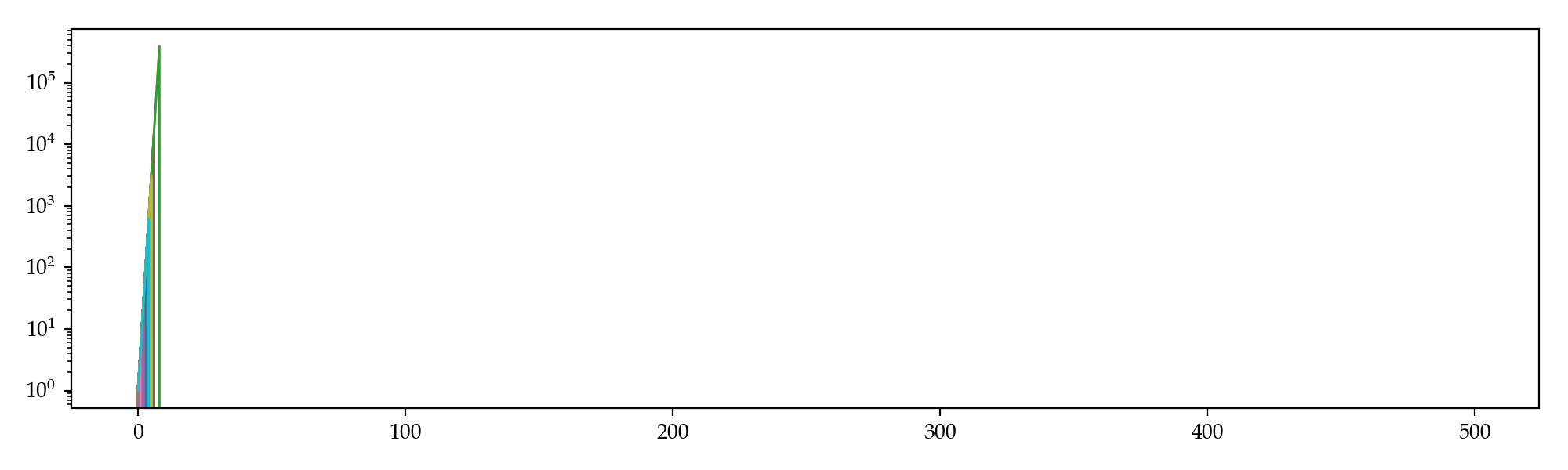# Kelly Criterion

If consider $$\log M_t$$ instead, then \begin{align*}\text{E}(\log M_t\mid \mathcal{F}_t) &=p\cdot \log[(1-x)M_t + xdM_t] +(1-p)\cdot \log[(1-x)M_t + 0]\\ &=p\cdot \log[(1-(1-d)x)M_t] +(1-p)\cdot \log[(1-x)M_t].\end{align*} The first order condition is $-\frac{\partial}{\partial x}\text{E}(\log M_t\mid \mathcal{F}_t) =\frac{p(1-d)}{1-(1-d)x}+\frac{1-p}{1-x} = 0 \quad\Rightarrow\quadx^* = \frac{pd-1}{d-1}=0.475$ which is more conservative and therefore, should survive longer than the previous strategy. Simulation gives the following trajectories: even the worst sim beat the best we got when $$x=1$$.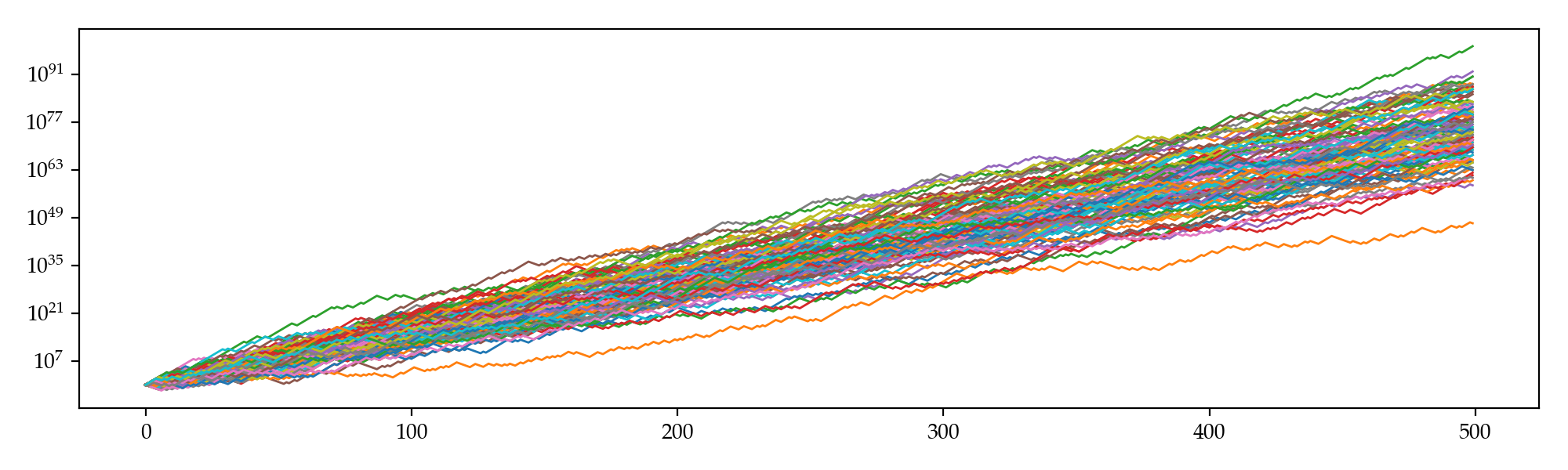# Further Thoughts

According to Doob's martingale inequality, the probability of our balance ever attaining a value no less than $$C = 1\times10^{60}$$ in $$T=500$$ steps is $\text{P}\left(\sup_{t \le T}M_t\ge C\right) \le \frac{\text{E}(M_T)}{C} = \frac{M_0}{C} \prod_{t=0}^{T-1}\frac{\text{E}(M_{t+1}\mid\mathcal{F}_t)}{M_t} =\frac{[1+(pd-1)x]^T}{C} \approx 4.6\times10^{139} \gg 1.$ This implies the superior limit of the probability that our balance exceeds $$1\times10^{60}$$ within $$500$$ steps is one (instead of what simulation gave us, which is around $$0.31$$). To put it differently, we actually might be able to find a certain strategy that is even significantly better than the one given by the Kelly criterion.

What is it, then? Or, does it actually exist? I don't have an idea yet, but perhaps exploratory algorithms like machine learning will give us some hints, and perhaps the strategy is not static but rather dynamic.

1. 1.In a game with odds $$(d-1)$$:$$1$$, for each dollar you bet, you either win $$d$$ dollars or lose all. ↩︎
2. 2.$$M_t$$ is a sub-martingale iff. $$\text{E}(M_{t+1}\mid \mathcal{F}_t) \ge M_t$$ for all $$t \ge 0$$. ↩︎
3. 3.Let $$X_t$$ be a non-negative sub-martingale, then $$\text{P}(\sup_{t\le T} X_t \ge C)\le \text{E}(X_T) / C$$. ↩︎
]]>
<p>I am recently playing a billiard game where you can play a series of exciting tournaments. In each tournament, you pay an entrance fee of, for example, <span class="math inline">$$\500$$</span>, to potentially win a prize of, say, <span class="math inline">$$\2500$$</span>. There are various kinds of tournaments with different entrance fees ranging from <span class="math inline">$$\100$$</span> up to over <span class="math inline">$$\10000$$</span>. After hundreds of games, my winning rate stablized around <span class="math inline">$$58\%$$</span>, which is actually pretty good as it significantly beats random draws. A natural concept therefore came into my mind: <strong>Is there an optimal strategy?</strong></p>
Deep Learning on MacOS with AMD eGPU? http://allenfrostline.com/2019/01/07/deep-learning-amd-gpu/ 2019-01-07T08:05:00.000Z 2019-01-07T08:20:02.170Z

I've recently sold my Nvidia GTX 1080 eGPU after two month's waiting in vain for a compatible Nvidia video driver for MacOS 10.14 (Mojave). Either Apple's or Nvidia's fault, I don't care any more. Right away, I ordered an AMD Radeon RX Vega 64 on Newegg. The card arrived two days later and it looked sexy at first sight. It's plug-and-play as expected and performed just as good as its predecessor, regardless of serious gaming, video editing or whatever. I would have given it a 9.5/10 if not find another issue a couple of days later — wow, there is no CUDA on this card!

Of course there isn't. Cause CUDA was developed by Nvidia who's been paying great efforts on making a more user-friendly deep-learning environment. Compared with that, AMD (yes!) used to intentionally avoid a head-to-head competition against world's largest GPU factory and instead keep making gaming cards with better cost-to-performance ratios. ROCm, which is an open-source HPC/Hyperscale-class platform for GPU computing that allows cards other than Nvidia's, does make this gap much narrower than before. However, ROCm is still publicly not supporting MacOS and you have to run a Linux bootcamp to utilize the computational benefits of your AMD card, even though you can already game smoothly on you Mac. Sad it is, AMD 😰.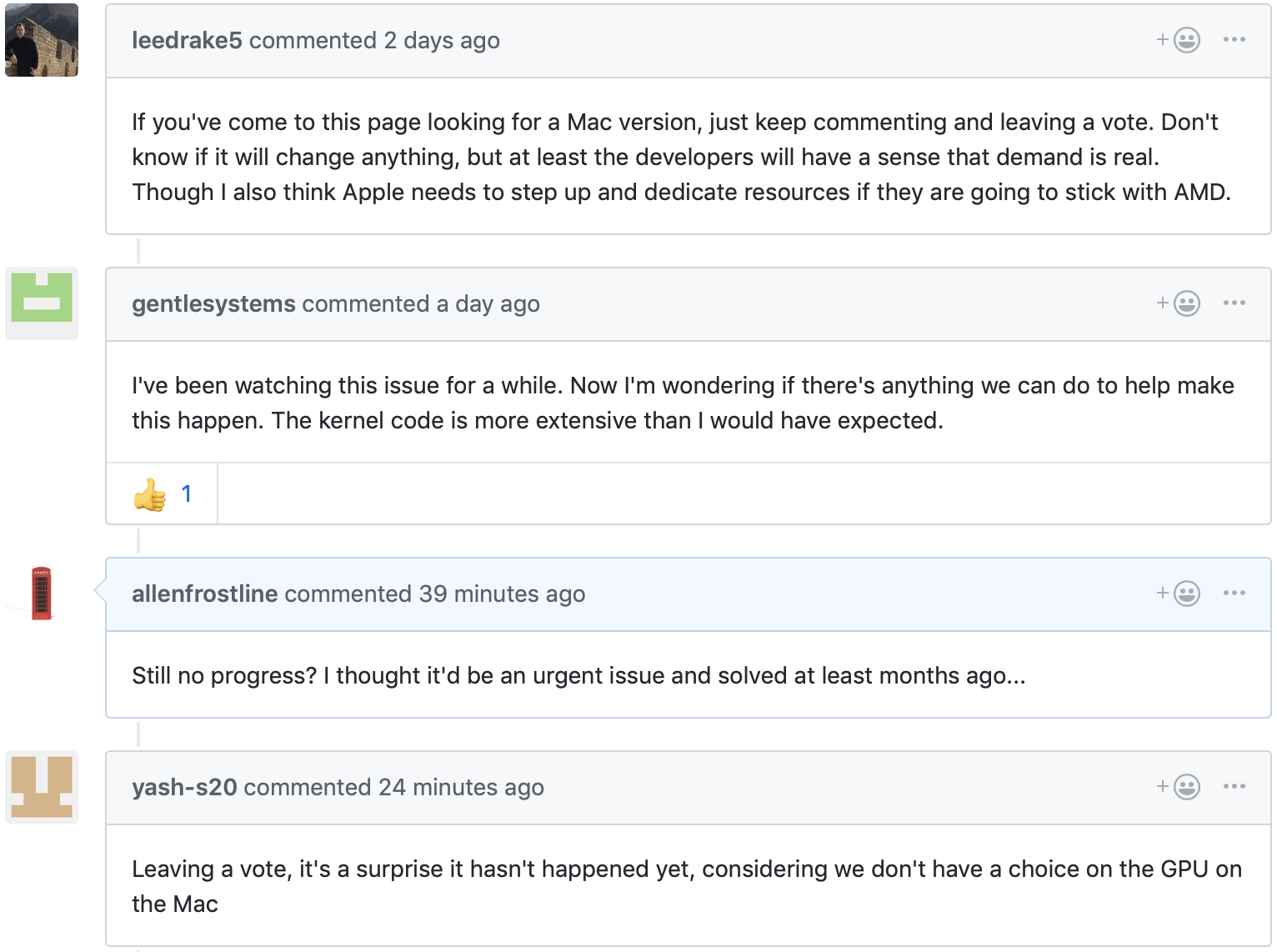There are, however, several solutions if you're people just like me who really have to run your code on a Mac and would like to accelerate those Renaissance training times with a GPU. The method I adapted was by using a framework called PlaidML, and I'd like to walk you through how I installed, and configured my GPU with it.

After installation, we can set up the intended device for computing by running:

PlaidML Setup (0.3.5)Thanks for using PlaidML!Some Notes:  * Bugs and other issues: https://github.com/plaidml/plaidml  * Questions: https://stackoverflow.com/questions/tagged/plaidml  * Say hello: https://groups.google.com/forum/#!forum/plaidml-dev  * PlaidML is licensed under the GNU AGPLv3 Default Config Devices:   No devices.Experimental Config Devices:   llvm_cpu.0 : CPU (LLVM)   opencl_intel_intel(r)_iris(tm)_plus_graphics_655.0 : Intel Inc. Intel(R) Iris(TM) Plus Graphics 655 (OpenCL)   opencl_cpu.0 : Intel CPU (OpenCL)   opencl_amd_amd_radeon_rx_vega_64_compute_engine.0 : AMD AMD Radeon RX Vega 64 Compute Engine (OpenCL)   metal_intel(r)_iris(tm)_plus_graphics_655.0 : Intel(R) Iris(TM) Plus Graphics 655 (Metal)   metal_amd_radeon_rx_vega_64.0 : AMD Radeon RX Vega 64 (Metal)Using experimental devices can cause poor performance, crashes, and other nastiness.Enable experimental device support? (y,n)[n]:

Of course we enter y. Before I choose device 4 (OpenCL with AMD) or 6 (Metal with AMD), I'd like to benchmark on the default device, CPU (LLVM). The test script (on MobileNet as an example) is

and the result shows

Running 1024 examples with mobilenet, batch size 1INFO:plaidml:Opening device "llvm_cpu.0"Downloading data from https://github.com/fchollet/deep-learning-models/releases/download/v0.6/mobilenet_1_0_224_tf.h517227776/17225924 [==============================] - 2s 0us/stepModel loaded.Compiling network...Warming up ...Main timingExample finished, elapsed: 3.0688607692718506 (compile), 61.17863607406616 (execution), 0.059744761791080236 (execution per example)Correctness: PASS, max_error: 1.7511049009044655e-05, max_abs_error: 6.556510925292969e-07, fail_ratio: 0.0

Now we run the set-up again and choose 4 (OpenCL with AMD). The result is

Running 1024 examples with mobilenet, batch size 1INFO:plaidml:Opening device "opencl_amd_amd_radeon_rx_vega_64_compute_engine.0"Model loaded.Compiling network...Warming up ...Main timingExample finished, elapsed: 2.6935510635375977 (compile), 13.741217851638794 (execution), 0.01341915805824101 (execution per example)Correctness: PASS, max_error: 1.7511049009044655e-05, max_abs_error: 1.1995434761047363e-06, fail_ratio: 0.0

Finally we run the test against the expected most powerful device, i.e. device 6 (Metal with AMD).

Running 1024 examples with mobilenet, batch size 1INFO:plaidml:Opening device "metal_amd_radeon_rx_vega_64.0"Model loaded.Compiling network...Warming up ...Main timingExample finished, elapsed: 2.243159055709839 (compile), 7.515545129776001 (execution), 0.007339399540796876 (execution per example)Correctness: PASS, max_error: 1.7974503862205893e-05, max_abs_error: 1.0952353477478027e-06, fail_ratio: 0.0

As a conclusion, by utilizing the Metal core on my Mac as well as the external AMD GPU, the training runtime was roughly 87.7% down and I'm personally quite satisfied with that.

1. 1.eGPU = external GPU. ↩︎
2. 2.You might have noticed an extra difference: it downloads the dataset on the first run. Doesn't really matter though. ↩︎
]]>
<p>I've recently sold my Nvidia GTX 1080 eGPU<sup id="fnref:1"><a href="#fn:1" rel="footnote"><span class="hint--top-right hint--error hint--large" data-aria-label="eGPU = external GPU. "></span></a></sup> after two month's waiting in vain for a compatible Nvidia video driver for MacOS 10.14 (Mojave). Either Apple's or Nvidia's fault, I don't care any more. Right away, I ordered an AMD Radeon RX Vega 64 on Newegg. The card arrived two days later and it looked sexy at first sight. It's plug-and-play as expected and performed just as good as its predecessor, regardless of serious gaming, video editing or whatever. I would have given it a 9.5/10 if not find another issue a couple of days later — wow, there is no CUDA on this card!</p>
To the Arctic Circle (Again)! http://allenfrostline.com/2019/01/04/to-the-arctic-circle-2/ 2019-01-04T16:27:40.000Z 2019-03-02T23:53:46.963Z

It's been more than two years since my last trip to the Arctic Circle when I was still studying in the Netherlands. Our adventurous hike in Abisko, in endless Northern European Mountains, was still a frequent dream of mine. This time we went to Fairbanks, Alaska, for Aurora and also, for another Arctic experience.]]>
<p>It's been more than two years since my last trip to the Arctic Circle when I was still studying in the Netherlands. Our adventurous hike in Abisko, in endless Northern European Mountains, was still a frequent dream of mine. This time we went to Fairbanks, Alaska, for Aurora and also, for another Arctic experience.</p>